Jekyll2022-03-20T17:38:47+01:00https://alexandrugris.github.io/feed.xmlFrom The Trenches - The CodeAlexandru Gris - Personal Blog Timeseries Analysis in Python2021-08-14T09:15:16+02:002021-08-14T09:15:16+02:00https://alexandrugris.github.io/programming/2021/08/14/timeseries-analysisThis post is about statistical models for timeseries analysis in Python. We will cover the ARIMA model to a certain depth.

### Linear Regression and Timeseries

Using a statistical tools such as linear regression with time series can be problematic. Linear regression assumes you have independently and normally distributed data, while, in time series data, points near in time tend to be strongly correlated with one another. This is precisely the property that makes timeseries analysis important as, if there aren’t temporal correlations, it would be impossible to perform tasks such as predicting the future or understanding temporal dynamics.

Linear regression can be used with timeseries when linear regression assumptions hold, for instance when the predicted variable is fully dependent on its predictors and the errors preserve the normality assumption with no autocorrelation. In such a case, the timeseries element is entirely embedded in one of the features.

### The Statistics Of Time Series

An excellent introduction on time series can be found here.

Timeseries bring several concepts of interest

• Stationarity: constant statistical properties of the timeseries (mean, variance, no seasonality)
• Self-correlation: correlation between subsequent values of a timeseries
• Seasonality: time-based patterns tha repeat at set intervals
• Spurious correlations: the propensity of timeseries to correlate with other unrelated timeseries especially when seasonality or trends are present.

A log transformation or a square root transformation are two usually good options for making a timeseries stationary,particularly in the case of changing variance over time.

Most of the timeseries have a trend, that is the mean is not constant - trend-stationarity and difference-stationarity. Removing a trend is most commonly done by differencing. Sometimes a series must be differenced more than once. However, if you find yourself differencing too much (more than two or three times) it is unlikely that you can fix your stationarity problem with differencing.

If `v(t_i+1) - v(t_i)` is random and stationary, then the process generating the series is a random walk, else a more refined model is required.

The test that is mainly used for testing stationarity is called the Augmented Dickey Fuller Test. It removes the autocorrelation and tests for equal mean and equal variance throughout the series. The null hypothesis is the non-stationarity.

The Dickey Fuller test assumes that the time series is an AR1 process (auto-regressive one), that is, it can be written as `y(t) = phi * y(t-1) + constant + error`. The DF test’s null hypothesis is `phi >= 1`. The alternate hypothesis is that `phi < 1`. This `phi == 1` is called a unit root. A good explanation of unit roots can be found here.

ADF extends the test to ARn series and this null hypothesis is that `sum(phi_i)>=1`. The difference between the basic DF test and the ADF test is that the latter makes is to account for more lags. The test of whether a series is stationary is a test of whether a series is integrated. An integrated series of order `n` is a series that must be differenced `n` times to become stationary.

``````import random

def gen_ts(start, coefs, count, error):
assert(len(start) == len(coefs))
assert(count > len(start))

lst = start +  * (count - len(start))

for i in range(len(start), count):
lst[i] = random.uniform(-0.5, 0.5) * error
for j in range (1, len(start)+1):
lst[i] += coefs[j-1] * lst[i-j]

return lst

v = gen_ts([0, 1, 2], [0.5, 0.2, 0.1], 100, 1.0) # sum of coefficients < 1
plt.plot(v)

# automatically test for the best lag to use
# AIC comes from https://en.wikipedia.org/wiki/Akaike_information_criterion
print(p_value)
``````

For detecting auto correlation, we introduce two measures:

• The ACF - the autocorrelation between the value at `t` and `t-n`, including the intermediary values, `(t-1 .. t-n-1)`. E.g. the effect of prices 2 months ago vs the prices today, including the effect of the prices 2 months ago on the prices 1 month ago and the prices from 1 month ago on today’s prices.
• The PACF - the autocorrelation between the value at `t` and `t-n` excluding the intermediary values.

The ACF is the Pearson correlation between the values `ti` and the lagged `ti-k` values. For the PACF we do a regression on the values of the timeseries at time `ti` on the `ti-k` of the form `ti=phi_1*ti_-1 + phi_2*ti_-2 + ... + phi_k*ti_-k + error_term` - autoregressive lag `k`. `phi_k` is our PACF(k).

To plot these a real dataset:

``````import pandas as pd

index_col=0,
names=['year', 'trappings'])
``````

And then transform it to time-annotated series:

``````lynx_ts = pd.Series(
lynx_df["trappings"].values,
pd.date_range('31/12/1821',
periods=len(lynx_df),
freq='A-DEC'))

lynx_ts.plot()
``````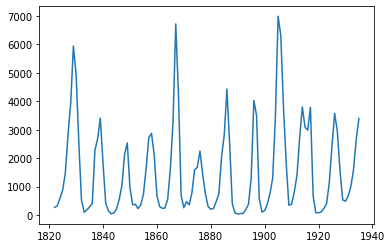``````from statsmodels.graphics.tsaplots import plot_acf, plot_pacf
plot_acf(lynx_ts, lags=100)
plot_pacf(lynx_ts, lags=10)
``````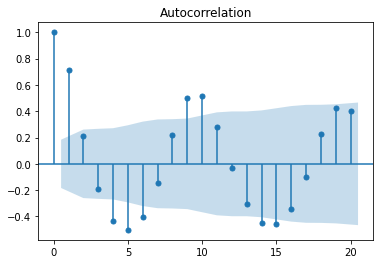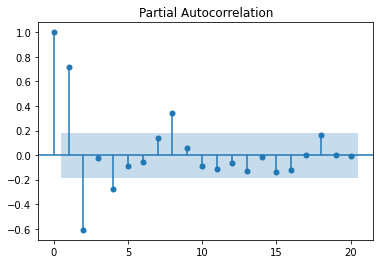We see in these charts that autocorrelation decreases as we look backwards in the series. This means that we are likely dealing with an auto-regressive process. If we are to build an auto-regressive model for this series we’ll probably consider the coefficients for the 1, 2, 4 and 6 lags. The blue bands are the error margin; everything within the bands are not statistically significant. The coefficient with index 0 is always 1, as it is the correlation of the timeseries with itself.

A series can be stationary, that is the mean is 0 and the variance constant with time, but with no lag auto-correlation, no matter the lag value. Such a series is called white noise and it is not predictable.

Let’s plot some generated time series to explore the PCF and PACF charts for various cases.

``````white_noise = np.random.normal(0, 10, size=100)
plot_acf(white_noise)
plt.show()
plot_pacf(white_noise)
plt.show()
``````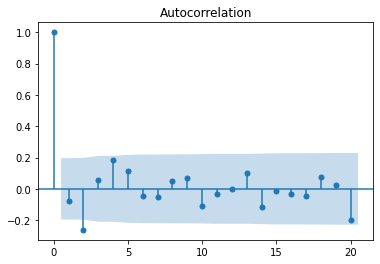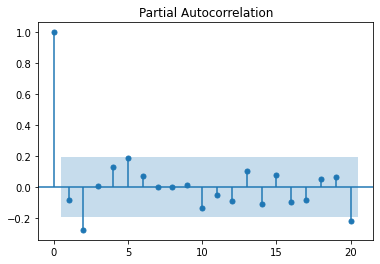Let’s plot a perfect AR(1) model, with no noise.

``````# ar(1) process
ts2 = [white_noise]
phi_0 = 10
phi_1 = 0.8
k = 0 # 0 = perfectly noiseless, 1 = very noisy

# Expected value of the timeseries (perfect timeseries converges to this value)
miu = phi_0 / (1-phi_1)
print("Expected value: ", miu)

for i in range(1, 100):
# note that without the error term this goes fast to the mean
# AR(1)
ts2.append(phi_0 + ts2[i-1] * phi_1 + k * white_noise[i])

ts2 = np.array(ts2)
plt.plot(ts2)

plot_acf(ts2, lags=40)
plt.show()
plot_pacf(ts2, lags=40)
plt.show()
``````

The time series converges to `miu=phi_0 / (1-phi_1) = 50`.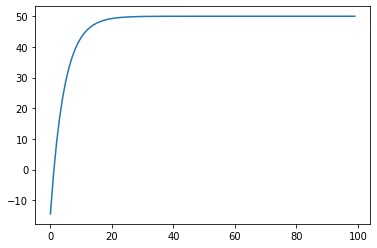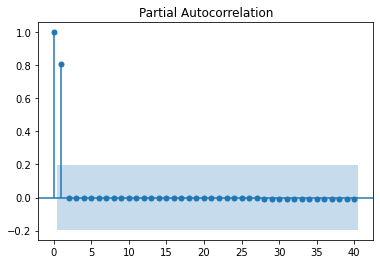The same expected value can be observed if we increase the noise: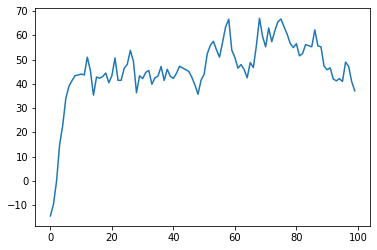Now, let’s plot an MA (moving average) process:

``````ts3 = [white_noise]
mean = 10
phi_1 = 0.8
for i in range(1, 100):
# MA(1) - coef applied to the previous error
ts3.append(mean + white_noise[i] + theta_1 * white_noise[i-1])

ts3 = np.array(ts3)
plt.plot(ts3)

plot_acf(ts3, lags=40)
plt.show()
plot_pacf(ts3, lags=40)
plt.show()
``````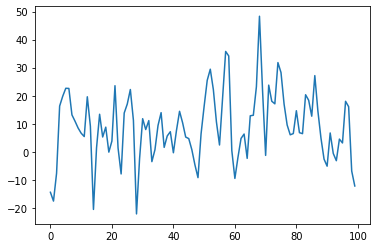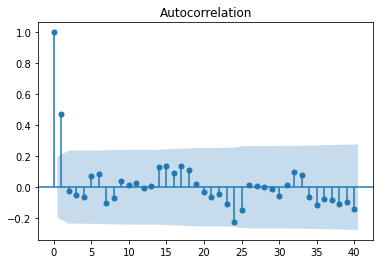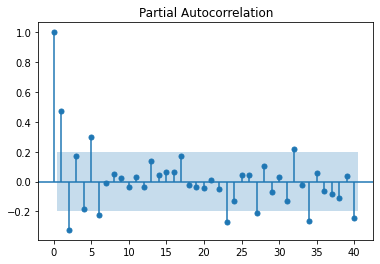Unlike an autoregressive process, which has a slowly decaying ACF, the definition of the MA process ensures a sharp cutoff of the ACF for any value greater than q, the order of the MA process. This is because an autoregressive process depends on previous terms, and they incorporate previous impulses to the system, whereas an MA model, incorporating the impulses directly through their value, has a mechanism to stop the impulse propagation from progressing indefinitely. This also means that, forecasting beyond the value lag value of q will only return the mean, since there is no more noise to incorporate.

To summarize, when trying to identify what kind of model we try to fit, we have the following rules:

• `AR(p)` - ACF falls off slowly, PACF has sharp drop after lag = p
• `MA(q)` - ACF has a sharp drop after lag = q, PACF falls off slowly
• `ARMA(p,q)` - No sharp cutoff, neither for ACF nor for PACF

### Fitting ARIMA Models

An ARIMA model has 3 parameters:

• `p` : the order of autoregression (the summation of the weighted lags) - `AR`
• `d` : the degree of differencing (used to make the dataset stationary if it is not) - `I`
• `q` : the order of moving average (the summation of the lags of the forecast errors) - `MA`

Examples (`ARIMA(p, d, q)`):

• `ARIMA (p=1, d=0, q=0) <=> Y(t) = coef + phi_1 * Y(t-1) + error(t)` - lag 1 autoregressive model
• `ARIMA (p=1, d=0, q=1) <=> Y(t) = coef + phi_1 * Y(t-1) + theta_1 * error(t-1) + error(t)` -
• `ARIMA (p=0, d=1, q=0) <=> Y(t) = coef + Y(t-1) + error(t)` is a random walk. The differencing equation, `Y(t) - Y(t-1) = coef + error(t)`, is needed so that the remaining `ARMA` model is applied on stationary data. A random walk is not stationary.
• `ARIMA(p=0, d=1, q=1)` is an exponential smoothing model

### ARIMA Model Parameter Selection

First step is to check for stationarity using the Augmented Dickey-Fuller test. If the data is not stationary, we need to set the `d` parameter.

The second step is to set the `p` and `q` parameters by inspecting the `ACF` and `PACF` plots, as described before.

To avoid over-fitting, a rule of thumb is to start the parameter selection with the plot that has the least amount of lags outside of the significance bands and then consider the lowest reasonable amount of lags. The ARIMA model is not necessary unique, as we will see in the following example where we start from a complex timeseries which can be approximated very well with a simpler model.

Let’s generate some data:

``````arma_series = [white_noise, white_noise]
m = 5
phi_1 = 0.4
phi_2 = 0.3
theta_1 = 0.2
theta_2 = 0.2

# AR(2) I(0) MA(2)
for i in range(2, 100):
arma_series.append( \
m + \
arma_series[i-1] * phi_1 + arma_series [i-2] * phi_2 + \
white_noise[i] + theta_1 * white_noise[i-1] + theta_2 * white_noise [i-2])

plt.plot(arma_series)
plt.show()

arma_series = np.array(arma_series)

# fit the model
plot_acf(arma_series)
plt.show()
plot_pacf(arma_series)
plt.show()
``````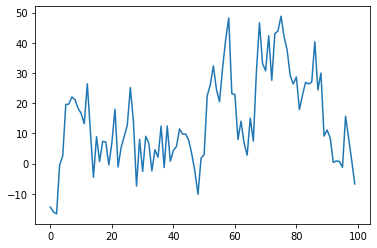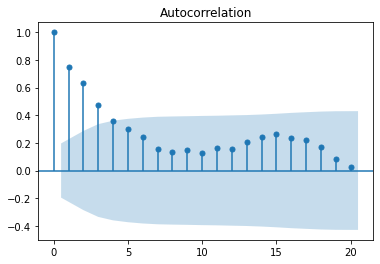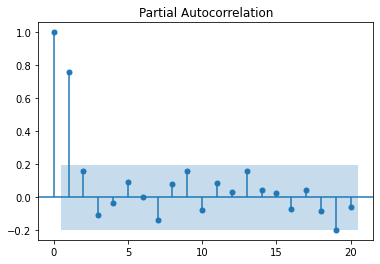We observe a sharp cutoff in the PACF after lag 1 and slow decay in the ACF. This leads to try to fit an `ARIMA(1, 0, 0)`.

``````from statsmodels.tsa.arima.model import ARIMA

m = ARIMA(arma_series, order=(1,0,0))
results = m.fit()
plt.plot(arma_series)
plt.plot(results.fittedvalues, color="orange")
print(results.arparams) # 0.78
``````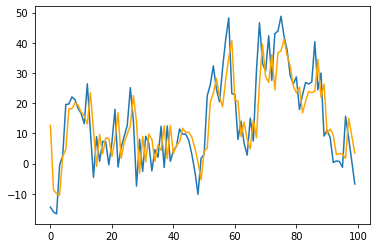A pretty good approximation of the initial complex model can be obtained with an AR(1) model. Let’s analyse the residuals to see how much information did we capture in our model and if there are autoregressive behaviors we have missed. In our case residuals are normally distributed as seen in the histogram and proven by the Shapiro test and in the ACF and PACF plots we do not see any autoregressive tendencies we might have missed.

``````resid = arma_series - results.fittedvalues
plt.hist(resid)

import scipy.stats as stats
# visual inspection of the residuals
plt.hist(resid)
# Shapiro test for normality
stats.shapiro(stats.zscore(resid))

# no autocorrelation
plot_acf(resid)
plt.show()
plot_pacf(resid)
plt.show()
``````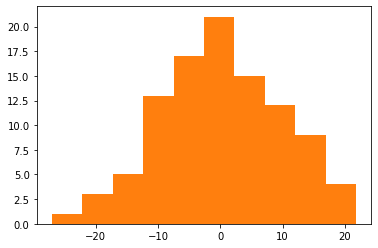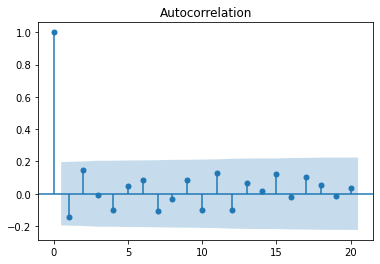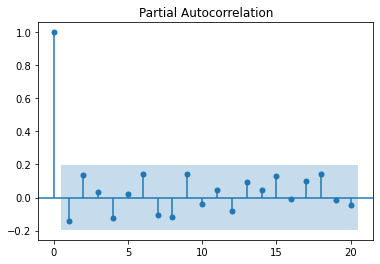Returning to our Lynx timeseries which was shown earlier, let’s train an ARIMA model and see how it fits.

``````from statsmodels.tsa.arima.model import ARIMA
m = ARIMA(lynx_ts, order=(, 0, 1))
results = m.fit()
plt.plot(lynx_ts)
plt.plot(results.fittedvalues, color="orange")
print(results.arparams)
``````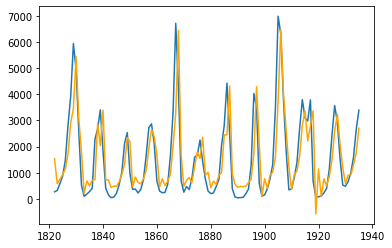Exported notebook is here

A good introductory video here

]]>
Simulated Annealing in Go2021-02-26T08:15:16+01:002021-02-26T08:15:16+01:00https://alexandrugris.github.io/programming/2021/02/26/golang-annealingAs Go is quickly becoming my favourite programming language, in this post we switch gears and implement an optimization algorithm - simulated annealing. We will solve the travelling salesman problem and, in the process, we will build a desktop app and a bare bones charting library.

### Simulated Annealing

Simulated annealing is an optimization algorithm used for solving complex problems where direct algorithmic solutions are hard to find. In cases where gradient descent cannot be used because the optimization function is not continuous we need a different approach, mostly based on trial and error. In such a situation, the solution is to make random moves in the solution space and only accept those moves that offer an optimization over the current state. However such moves can easily converge to a local optimum and get stuck. Therefore, a mechanism in needed to allow escape from the local optimum. Simulated annealing offers a solution to this problem allowing random non-optimal moves to be accepted with decreasing probability, based on a temperature schedule. The solution is borrowed from metalurgy where the steel is forged under slow cooling in order to allow for an optimal alignment of metal particles for increased durability.

For our problem the metric we want to optimize is the total length of the path. In the picture below you can see such a layout with a shortest path determined by the algorithm.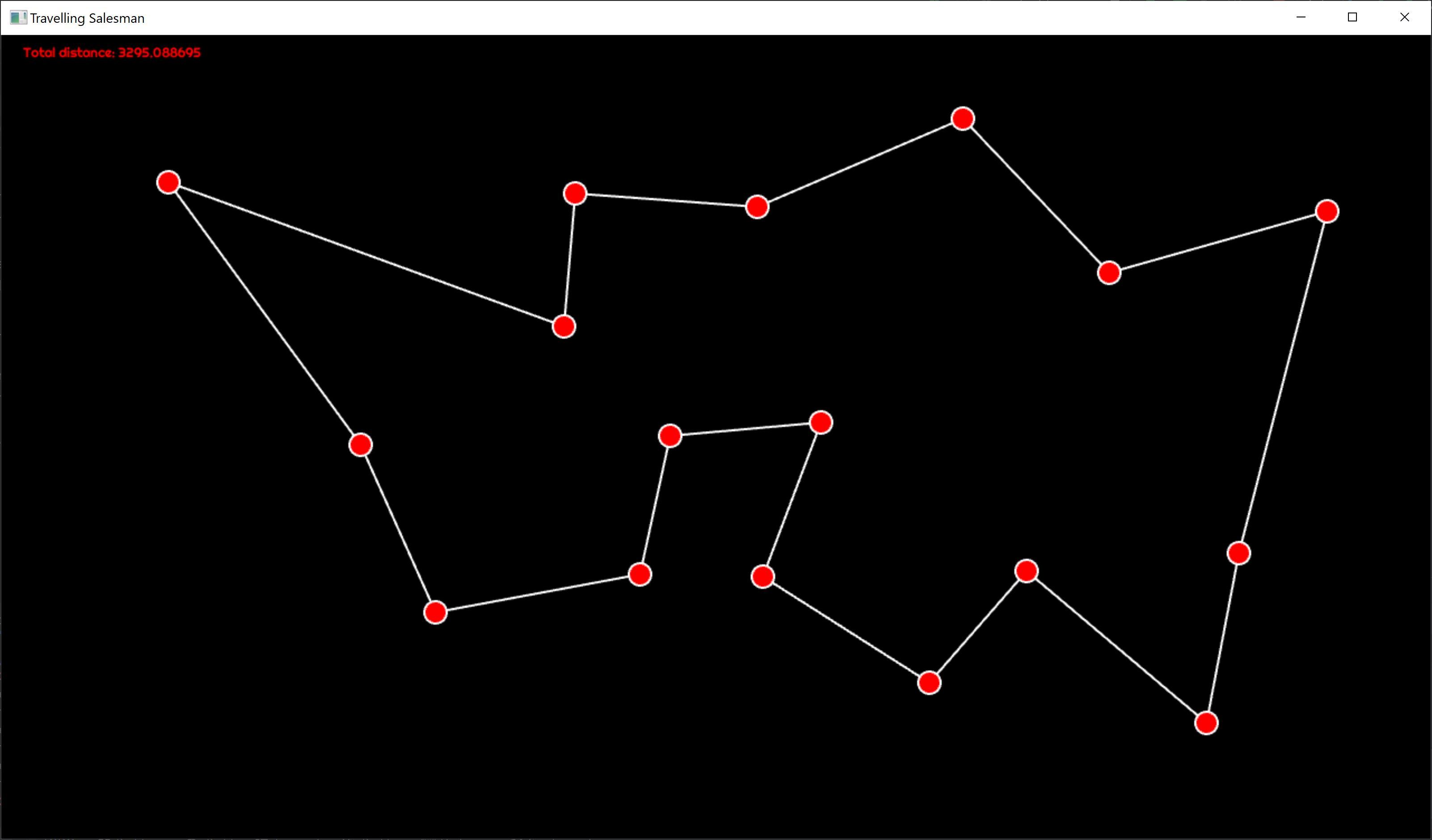For the very same configuration we see the total path length plotted for each iteration.It is interesting to see the inflection point where, after settling on a higher length equilibrium, an local optimum, and a random move, the total length resettles to another, global optimum. We also see how fewer and fewer random moves are accepted, with lower and lower distance increase.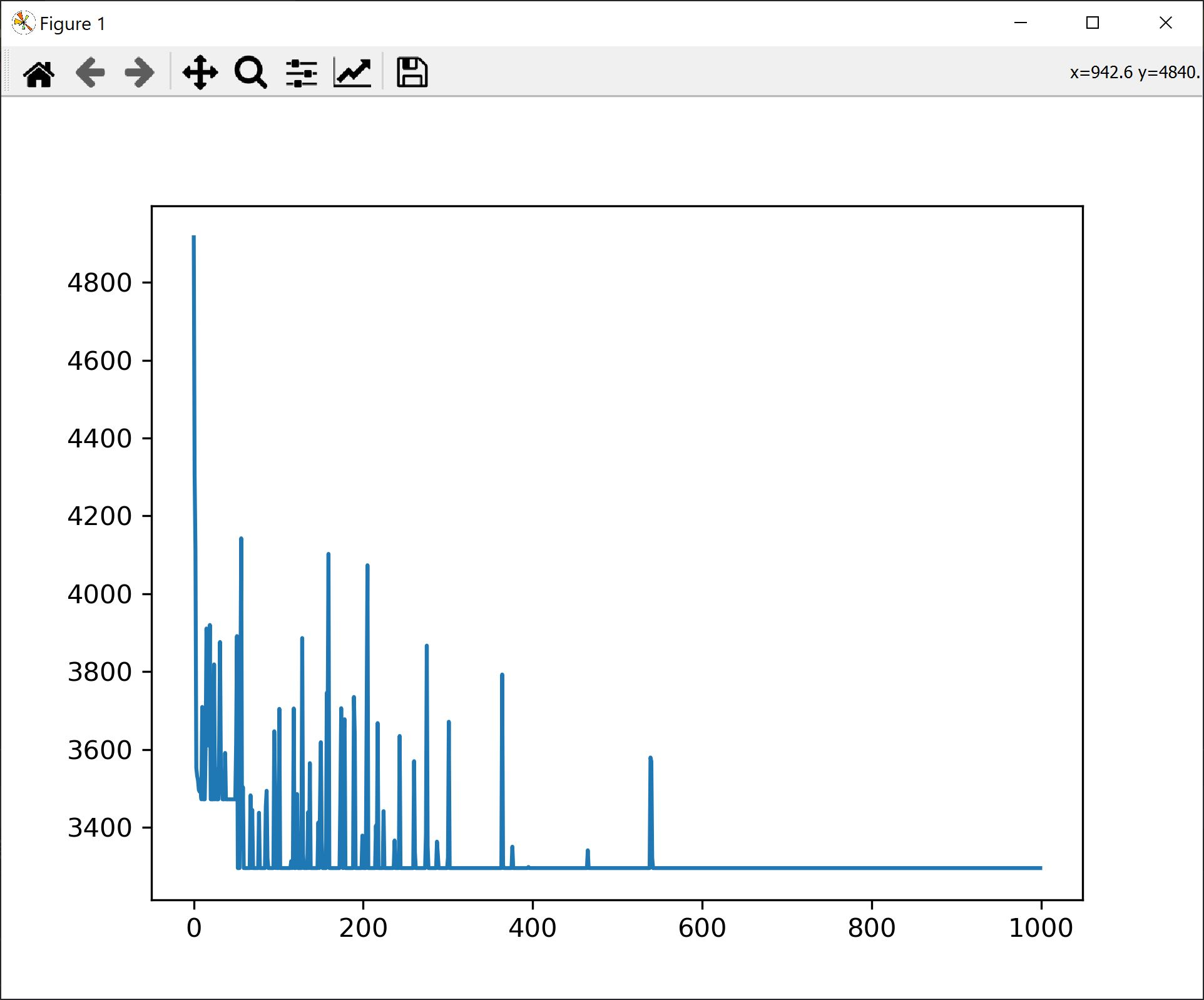### Solution Implementation

The full code is listed below. In short, we are computing the algorithm on a separate thread from the main rendering thread. The config can be reset by pressing `ESC`. You can add new points to the path by simply clicking somewhere on the screen. The distance evolution over each iteration can be displayed by pressing the `P` key.

The algorithm can be tuned by adjusting:

• The total number of iterations
• The temperature decay function
• The function for the probability of acceptance of a bad move

The algorithm has also a back step - if after accepting a bad move a better move is not found in a predefined number of steps, we backtrack to the best known configuration.

The move is made by randomly selecting two edges and switching their ends between themselves. Once the swap has been performed, in order fo maintain the path consistency, all edges between the two end points are inverted. This happens in the `ComputeNewPath` function.

``````package main

import (
"GoAI/plt"
"fmt"
"github.com/tfriedel6/canvas/sdlcanvas"
"math"
"math/rand"
)

type Point struct {
X float64
Y float64
}

type Connection struct {
Start int
End   int
}

func (p *Point) Subtract(other *Point) Point {
return Point{
X: p.X - other.X,
Y: p.Y - other.Y,
}
}

func (p *Point) DistanceTo(other *Point) float64 {
d := other.Subtract(p)
return math.Sqrt(d.X*d.X + d.Y*d.Y)
}

type ConnsCollection struct {
Points []Point
Conns  []Connection

// map ending to index in Conn
endsIn []int
}

func (cc *ConnsCollection) BuildEndsInMap() {

if cc.endsIn == nil || len(cc.endsIn) != len(cc.Conns) {
cc.endsIn = make([]int, len(cc.Conns))
}

for i, cn := range cc.Conns {
cc.endsIn[cn.End] = i
}
}

func (cc *ConnsCollection) ComputeDistance() (float64, bool) {
d := 0.0
for _, c := range cc.Conns {
if c.Start >= len(cc.Points) || c.End >= len(cc.Points) {
return -1, false
}
d += cc.Points[c.Start].DistanceTo(&cc.Points[c.End])
}
return d, true
}

func (cc *ConnsCollection) DuplicateConnectionsTo(other **ConnsCollection) {

if *other == nil {
*other = &ConnsCollection{
Points: cc.Points,
Conns:  make([]Connection, len(cc.Conns)),
endsIn: make([]int, len(cc.endsIn)),
}
}

copy((*other).Conns, cc.Conns)
copy((*other).endsIn, cc.endsIn)

}

func (cc *ConnsCollection) ComputeNewPath() float64 {

conns := cc.Conns

if len(conns) <= 1 {
return 0.0
}

i1 := rand.Int() % len(conns)
i2 := rand.Int() % len(conns)
if i1 == i2 {
i2++
if i2 == len(conns) {
i2 = 0
}
}

p1 := &conns[i1]
p2 := &conns[i2]

// swap edges
p1.End, p2.Start = p2.Start, p1.End

for idx := p1.End; idx != p2.Start; {
c := &conns[cc.endsIn[idx]]
c.Start, c.End = c.End, c.Start
idx = c.End
}

d, _ := cc.ComputeDistance()
return d
}

func InitConnectionsFromPoints(points []Point) *ConnsCollection {

c := ConnsCollection{
Points: points,
Conns:  make([]Connection, 0, 20),
}

// crate a path where each point is travelled only once
for i := range c.Points {

s := i
e := i + 1

if e == len(c.Points) {
e = 0
}

c.Conns = append(c.Conns, Connection{
Start: s,
End:   e,
})
}

c.BuildEndsInMap()

return &c
}

func TravellingSalesman(in <-chan []Point, out chan<- *ConnsCollection) {

for {

// read all points and only start the computation once I finished points
points := <-in
for len(in) > 0 {
points = <-in
}

var conns, conns2, resetPoint *ConnsCollection
conns = InitConnectionsFromPoints(points)
conns.DuplicateConnectionsTo(&conns2)
conns.DuplicateConnectionsTo(&resetPoint)

d, _ := conns.ComputeDistance()
dReset := d
MaxDriftFromGlobalMinimum := 10 * len(points)
countSinceReset := MaxDriftFromGlobalMinimum

MaxIterations := 100000
distanceEvolution := make([]float64, MaxIterations)

for i := 0; i < MaxIterations; i++ {

temperature := 0.1 * float64(MaxIterations-i) / float64(MaxIterations)
temperature = math.Pow(temperature, 5)

// switch two nodes
d2 := conns2.ComputeNewPath()

// found a better move
// or the temperature is high enough to accept other moves
if d2 < d || (d2-d)*temperature > rand.Float64() {

if d2 > d && i > (MaxIterations/100)*50 {
fmt.Printf("Accepted bad move: iter: %v, temp: %v, distance: %v\n", i, temperature, d2-d)
}

conns2.BuildEndsInMap()
conns2.DuplicateConnectionsTo(&conns)
d = d2

if d < dReset {
dReset = d
countSinceReset = MaxDriftFromGlobalMinimum
conns2.DuplicateConnectionsTo(&resetPoint)
}

} else if countSinceReset < 0 {
d = dReset
countSinceReset = MaxDriftFromGlobalMinimum
resetPoint.DuplicateConnectionsTo(&conns)
resetPoint.DuplicateConnectionsTo(&conns2)
//fmt.Println("Reset")
} else {
conns.DuplicateConnectionsTo(&conns2) // re-init conns2
}

countSinceReset--

// save for analysis
distanceEvolution[i] = d
}

plt.Reset()
plt.LinePlot(distanceEvolution, "Distance Evolution", 1000)

if d > dReset {
out <- resetPoint
} else {
out <- conns
}
}
}

func main() {
wnd, cv, err := sdlcanvas.CreateWindow(1280, 720, "Travelling Salesman")
if err != nil {
panic(err)
}
defer wnd.Destroy()

points := make([]Point, 0, 10)
connections := make([]Connection, 0, 10)
distance := 0.0

submitPoints := make(chan []Point, 100)

wnd.MouseDown = func(btn int, x int, y int) {
// on mouse down add new points
points = append(points, Point{
X: float64(x),
Y: float64(y),
})

// send the points to be computed
submitPoints <- points
}

wnd.KeyDown = func(scancode int, rn rune, name string) {
switch name {
case "Escape":
points = make([]Point, 0, 10)
connections = make([]Connection, 0, 10)
distance = 0.0
case "KeyP":
plt.Execute() // show plot only when key is pressed
}
}

wnd.MainLoop(func() {

select {

if dd, ok := cc.ComputeDistance(); ok {
distance = dd
connections = cc.Conns
fmt.Printf("New paths with distance %f\n", distance)
}

default:

}

// background
w, h := float64(cv.Width()), float64(cv.Height())
cv.SetFillStyle("#000")
cv.FillRect(0, 0, w, h)

// circles
cv.SetStrokeStyle("#FFF")
cv.SetLineWidth(2)
cv.SetFillStyle(255, 0, 0)

for _, c := range connections {
cv.BeginPath()
cv.MoveTo(points[c.Start].X, points[c.Start].Y)
cv.LineTo(points[c.End].X, points[c.End].Y)
cv.Stroke()
}

for _, p := range points {
cv.BeginPath()
cv.Arc(p.X, p.Y, 10, 0, math.Pi*2, false)
cv.ClosePath()
cv.Fill()
cv.Stroke()
}

cv.SetFont("Righteous-Regular.ttf", 12)
cv.FillText(fmt.Sprintf("Total distance: %f", distance), 20, 20)

})
}

``````

### Easy Charting From Go

Since I couldn’t find any charting library that met my needs, to be easy to use from a desktop application, I’ve decided to build my own. It relies on the excellent matplotlib library from python. The solution is simple: it generates a python script containing all the values I need to plot and, then, it launches that script in a separate process with its own window. For this we are using golang text templates to generate the script. I will extend the library for future use with other types of charts. Below you can see the code:

The template:

``````import matplotlib.pyplot as plt

values=
plt.plot(values)

plt.show()
``````

The code for generating the template:

``````package plt

import (
"fmt"
"io/ioutil"
"log"
"os"
"os/exec"
"strings"
"text/template"
)

type Plot struct {
Type   string
Values [][]float64
Name   string
Count  int
}

var plots []Plot = nil
var tmpl *template.Template = nil

func min(a int, b int) int {
if a < b {
return a
} else {
return b
}
}

func compressByMean(count int, arr []float64) []float64 {

ret := make([]float64, count)
intvLen := len(arr) / count
cnt := float64(intvLen)

for i := 0; i < count-1; i++ {

upperLimit := (i + 1) * intvLen
lowerLimit := i * intvLen

ret[i] = arr[lowerLimit] / cnt

for j := lowerLimit + 1; j < upperLimit; j++ {
ret[i] += arr[j] / cnt
}
}

// last one is the last value - a hack for the simulated annealing problem
ret[count-1] = arr[len(arr)-1]
return ret
}

func toPythonArray(arr []float64) string {
sb := strings.Builder{}
sb.WriteString("[")

for i, v := range arr {
sb.WriteString(fmt.Sprintf("%f", v))
if i < len(arr) {
sb.WriteString(", ")
}
}

sb.WriteString("]")
return sb.String()
}

func init() {

log.Println(os.Getwd())

fn := template.FuncMap{
"CompressByMean": compressByMean,
"ToPythonArray":  toPythonArray,
}

tmpl = template.Must(template.New("chart_template.gopy").Funcs(fn).ParseFiles("chart_template.gopy"))
}

func LinePlot(arr []float64, name string, count int) {

v := Plot{
Type:   "line",
Values: make([][]float64, 1),
Name:   name,
Count:  count,
}

v.Values = arr
plots = append(plots, v)
}

func Reset() {
// clear the plots
plots = nil
}

func Execute() {

var fileName string

func(fn *string) {
f, err := ioutil.TempFile("./plots", "plt*.py")

if err != nil {
fmt.Println(err)
return
}

defer f.Close()
*fn = f.Name()

if err := tmpl.Execute(f, plots); err != nil {
log.Panic(err)
}

Reset()

}(&fileName)

go func(fileName string) {
if out, err := exec.Command("python", fileName).Output(); err != nil {
log.Println(err)
log.Println(out)
} else {
log.Println(out)
}
}(fileName)

}
``````
]]>
First Steps In Go - WebSockets2021-01-23T08:15:16+01:002021-01-23T08:15:16+01:00https://alexandrugris.github.io/programming/2021/01/23/golang-first-websocketsThese are my first steps in Go, this time learning how to extend my previous web service with WebSockets. The brower subscribes to changes to a set of products by sending one or more Subscribe or Unsubscribe JSON messages to the service, through a WebSocket connection. Each message contains a series of product IDs. The server maintains the map connection - subscriptions and listens to notifications on product changes from a Postgres database. The post also touches HTTPS, HTTP/2 and server push.

### Testing

We will be building on the foundations laid in the previous blogpost. The code is on GitHub, here

To open and send commands to our WebSocket server, in Javascript Console, in any browser, you can do:

``````// connect to our websocket endpoint
let ret = new WebSocket("ws://localhost:8080/websocket")

// subscribe to changes to the first 1024 product IDs
req.send(JSON.stringify({command: "subscribe", productIDs: [...Array(1024).keys()]}))
req.onmessage = (msg) => console.log(msg)
``````

Later, when we want to test the connection closing, do

``````req.close()
``````

Meanwhile, from a different console, we will be performing `POST`, `PUT` and `DELETE` requests to change the products in the database. These requests are similar to the following:

``````POST http://localhost:8080/products
Content-Type: application/json

{
"productId": 0,
"manufacturer": "Apple",
"pricePerUnit": "500EUR",
"unitsAvailable": 6,
"productName": "MacBook Pro"
}
``````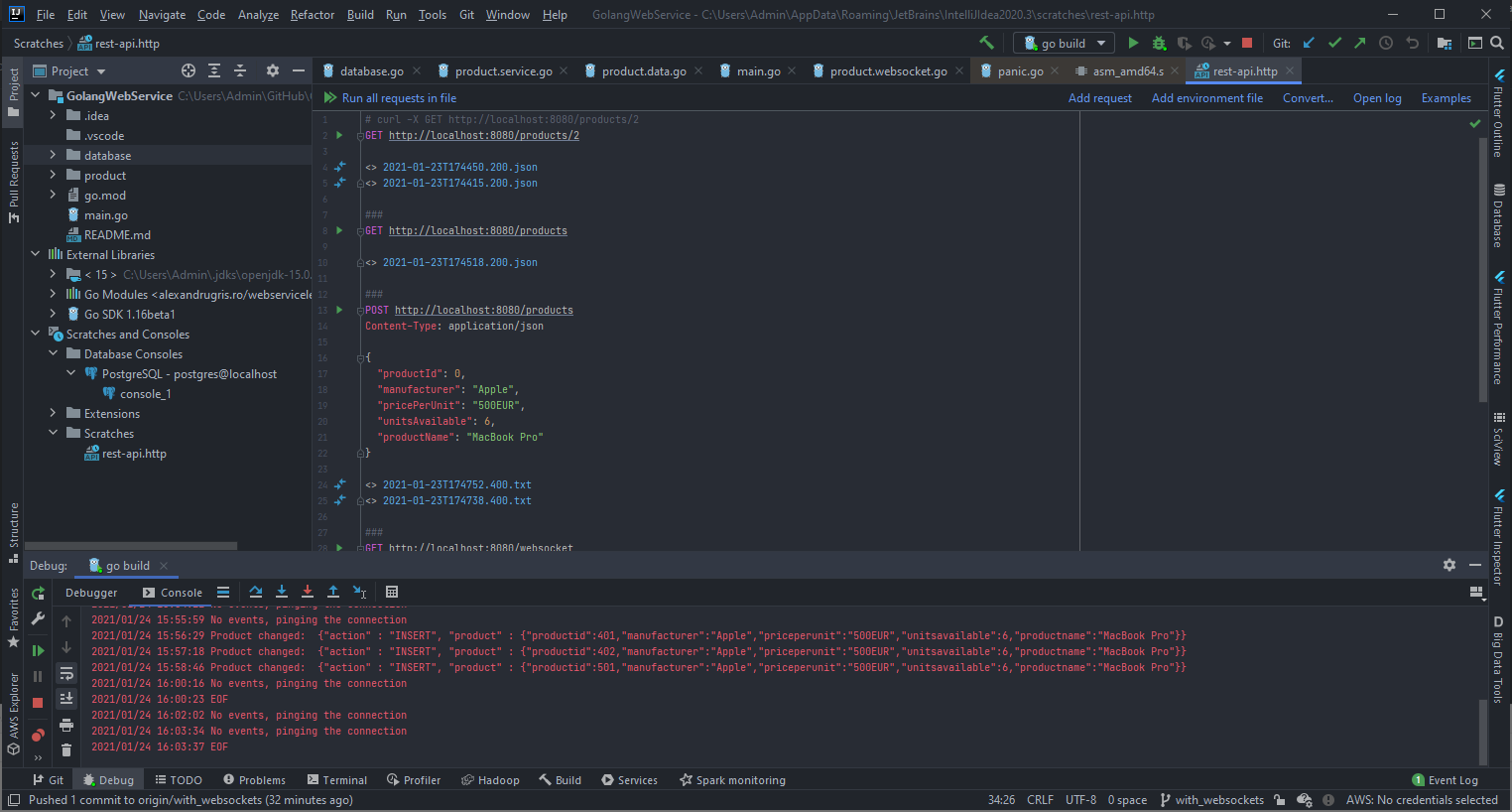### Database Changes

In order to be able to listen to changes in the database, we will use the `LISTEN` / `NOTIFY` system from Postgresql. We are going to create a trigger which sends `JSON` messages whenever an update to the Products table occurs and we are going to initialize a listener in our service code to such events.

The trigger procedure below:

``````CREATE OR REPLACE FUNCTION notify_event_on_products_update() RETURNS TRIGGER AS \$\$
DECLARE
data json;
notif json;
BEGIN
IF (TG_OP = 'DELETE') THEN
data = row_to_json(OLD);
ELSE
data = row_to_json(NEW);
END IF;

notif = json_build_object(
'action', TG_OP,
'product', data
);
PERFORM pg_notify('product_change', notif::text);

RETURN NULL;
END
\$\$ LANGUAGE plpgsql;

DROP TRIGGER IF EXISTS products_change_trigger ON products;

CREATE TRIGGER products_change_trigger AFTER INSERT OR UPDATE OR DELETE ON products
FOR EACH ROW EXECUTE PROCEDURE notify_event_on_products_update();
``````

Now, that we have this procedure, the code that listens to the emitted events, is listed below. It is invoked as a goroutine and acts as a backgorund service. It uses directly the `pq` package instead of the `sql` package because it relies on native Postgres functionality - the listen/notify mechanism.

``````// ListenForNotifications should be invoked as a goroutine
func ListenForNotifications(event string, notif func(json []byte)) error {

listener := pq.NewListener(ConnectionString, 1*time.Second, 10*time.Second,
func(ev pq.ListenerEventType, err error) {
log.Println(ev)
if err != nil {
log.Println(err)
}
})

if err := listener.Listen(event); err != nil {
return err
}

for {
select {
case n := <-listener.Notify:
notif([]byte(n.Extra))

case <-time.After(90 * time.Second):

log.Println("No events, pinging the connection")
if err := listener.Ping(); err != nil {
fmt.Println(err)
return err
}
}
}
}
``````

The snippent which launches listening is in the main function,

``````go func() {
log.Fatal(err)
}
}()
``````

### The WebSocket Endpoint

First, initialize the route. Please notice the `websocket` package instead of the `http` used above.

``````func GetHTTPHandlers() map[string]http.Handler {
return map[string]http.Handler{
"/products":  http.HandlerFunc(productsHandler),
"/products/": http.HandlerFunc(productHandler),
// new handler for websocket
// notice the websocket. package instead of the http.
"/websocket": websocket.Handler(productChangeWSHandler),
}
}
``````

And then the handler itself. Its structure is straight forward:

• Exit the function when the connection closes.
• Register a cleanup sequence for when the connection finished.
• Launch a goroutine to listen to incoming messages and EOF error, signifing the connection closing.
• Loop in the same goroutine to send the relevant data to the client.
``````func productChangeWSHandler(ws *websocket.Conn) {

// make the chan buffered so we can receive more messages until we process them
inMsgChan := make(chan inMessage, 1024)
inProductsUpdated := make(chan *Product, 1024)

defer func() {
Cmd:      "closeconn",
CommChan: inProductsUpdated,
}

// drain the channel
for range inProductsUpdated {
}

}()

go func(ws *websocket.Conn) {
for {
var msg inMessage

if err := websocket.JSON.Receive(ws, &msg); err != nil {
log.Println(err)
break
}
inMsgChan <- msg
}
close(inMsgChan)
}(ws)

for {
select {
case msg, ok := <-inMsgChan:
// subscribe - unsubscribe
if !ok {
return // connection close
} else {

Cmd:        msg.Cmd,
ProductIDs: msg.ProductIDs,
CommChan:   inProductsUpdated,
}

}
case product, ok := <-inProductsUpdated:
// updated products
if !ok {
return
}

if err := websocket.JSON.Send(ws, product); err != nil {
log.Println(err)
return
}
}
}
}
``````

### The Algorithm

The algorithm is straight forward. It keeps a map of productIDs - channels listening for updates. When an update comes for a specific product ID, all the channels are notified. The interesting part is the use of goroutines for synchronization between processes. The map is local to a goroutine, which is launched as a service when the application starts, and all communications with it happen over channels. There is no shared memory involved and no shared-memory-specific synchronization primitives. The commented ode below.

A notable mention is the fact that clearing the subscription on `connclose` event is very slow as the function has to iterate through all the registered products. In a production scenario, I’d keep another map, a reversed index, so that I the relationship channel -> productID is faster to navigate. In our case it would have only make the code longer and less readable.

``````// shared channel on which the listen-notify db mechanism sends the products
var prodChan = make(chan productNotification, 1024)

// shared channel on which subscriptions are added / removed

func handleDistributionGoroutine() {

// our map, product id -> channels
// the second map is used because there is no Set in go

for {

select {

case incomingProduct := <-prodChan:

if exists && notifChans != nil {
for k, v := range notifChans {
if v {
// this will block all threads in case of a single slow reader
// the chan will fill and it will not be possible to send other
// option is to do launch each as a separate goroutine
// but it will not guarantee order at the receiving side
go func() { k <- &incomingProduct.Product }()
}
}
}

switch subscription.Cmd {

case "subscribe":
for _, prd := range subscription.ProductIDs {
if !ok {
ret = make(map[chan *Product]bool)
}
ret[subscription.CommChan] = true
}
case "unsubscribe":
for _, prd := range subscription.ProductIDs {
}

case "closeconn":

// empty the rest
// we might wrap the following in a goroutine
// so we don't block futher incoming messages
emptyKeys := make([]int, 0, 100)

for k, v := range notifyUpdates {
delete(v, subscription.CommChan)
if len(v) == 0 {
emptyKeys = append(emptyKeys, k)
}
}
// clear the map of empty keys
for _, k := range emptyKeys {
}
close(subscription.CommChan)

default:
log.Printf("Unhandled command %v", subscription.Cmd)
}
}
}
}

// module init function to start the map service
func init() {
go handleDistributionGoroutine()
}
``````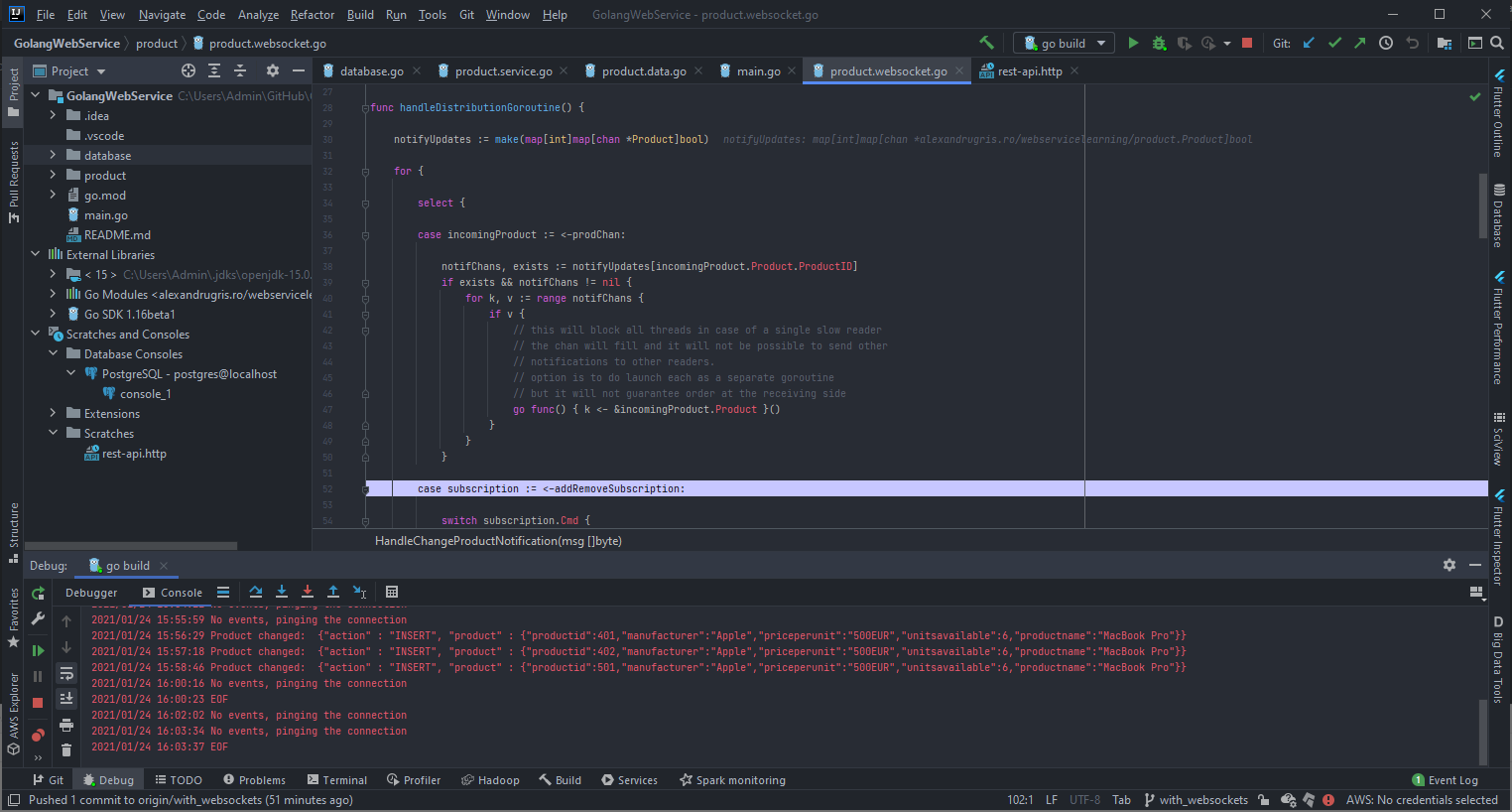### HTTPS and HTTP/2

Before we launch to production our service, we need to make sure we secure it. In order to accept connections over HTTPS, we need to change our `http.ListenAndServe` invocation to `http.ListenAndServeTLS` and provide the call a certificate. We are going to generate ourselves such a certificate using `generate_cert.go` utility from the `crypto/tls` package.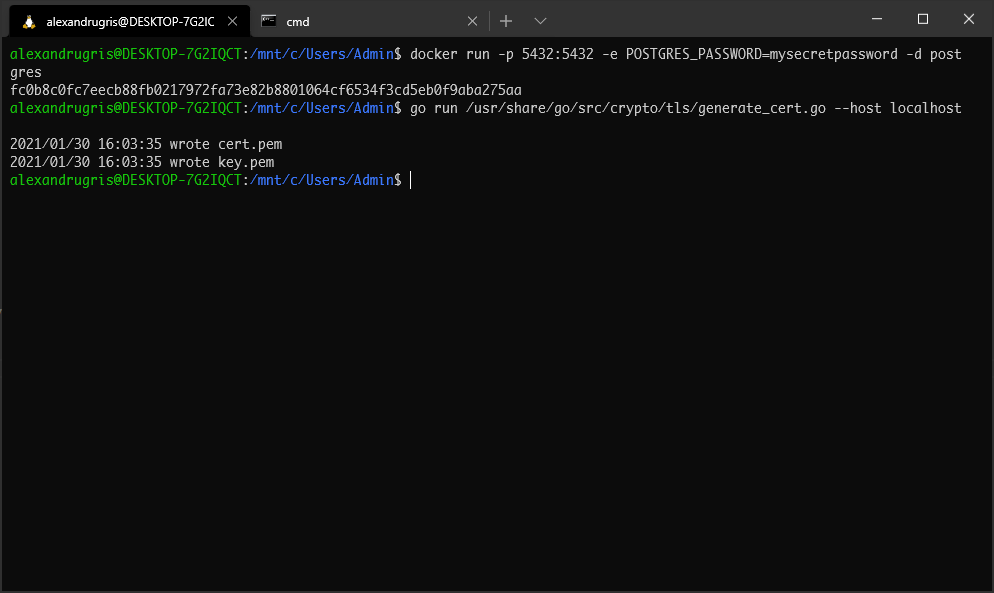The `cert.pem` file is the certificate with my public key inside while `key.pem` is my private key. When the session is established, a shared session key is generated by the client, signed with my public key. It is only I who can decode the shared key using my private key. The shared key is used to symmetrically encrypt messages

If I try to open now my service in Fireforx I get a security warning, but after I accept the warning I can access my service over https.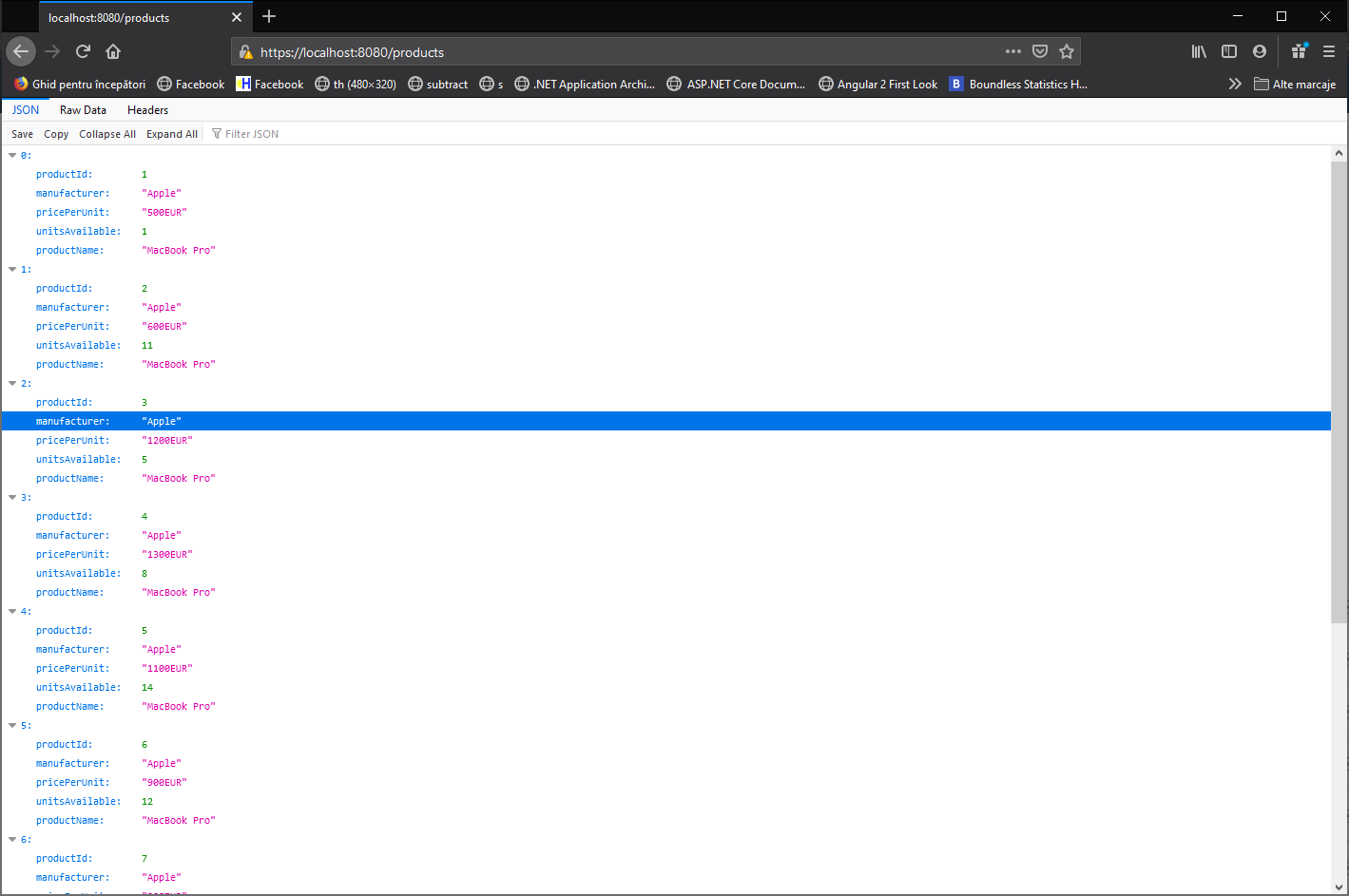The nice thing about go is that, once I upgrade to HTTPS, I automatically upgrade to HTTP/2. This change comes for free and includes out-of-the-box features such as:

• Request multiplexing
• Security by default, since it is running over HTTPS
• Server push

From these, we will discuss a bit server push. What server push does is to send to the client assets which were not previously requested before they are requested. An example is when the browser requires `index.html` and we know that it is styled with `main.css`, append to the request this file also. This saves loading times and browser roundripts. A problem arises when the asset is cached with `Cache-Control` in which condition it will get pushed anyway, increasing the size of the request. A simple solution to this issue is to set a cookie when the page is visited and, if the cookie is present, do not send the asset with server push. If the cookie is present we can safely assume the browser has the asset already cached and, if not, it will be requested anyway when it encounters it.

Since not all connections have the ability to do server push, we need to check for this capability. In our handler we do:

``````func mySeverPushRequest(w http.ResponseWriter, r *http.Request) {

// get the pusher interface out of our writer
if pusher, ok := w.(http.Pusher); ok {

// set cookie and cache control for one hour
pusher.Push("main.css", &http.PushOptions {
"Content-Type": []string{"text/css"} ,
"Cache-Control": []string{"max-age=3600"},
}
})

// 1h expiration time
Name: 		"assetspushed",
Value:		"true",
Expires:	expiration,
}
}
}

// continue serving files or executing templates
[.......................]
}
``````
]]>
First Steps In Go - Web Services2021-01-12T08:15:16+01:002021-01-12T08:15:16+01:00https://alexandrugris.github.io/programming/2021/01/12/golang-first-web-servicesThese are my first steps in Go, this time learning how to build web services. The post touches handling requests, json serialization, middleware, logging, database access and concurrency. Websockets and templates will be covered in a future post.

### Listening to Incoming Requests

For building HTTP services, golang comes with all batteries included. There’s no need to install any additional package, everything is already available in the standard library. The APIs are straight forward and the code is short and fast.

``````package main

import (
"log"
"net/http"
)

func customEndpoint(w http.ResponseWriter, r *http.Request) {
w.Write([]bytes("Hello World"))
log.Println("Served.")
}

func main() {

// a /custom endpoint
http.HandleFunc("/custom", customEndpoint)

// listen on localhost, port :8080
if err := http.ListenAndServe(":8080", nil); err != nil {
log.Fatal(err)
}

}
``````

### Handling JSON

If we want to export a field from a structure to JSON, its name has to start with a capital letter, making it a public symbol. Otherwise it will be considered as private and it will not appear in the output string.

We use annotations, which are accessible at runtime through reflection, to specify how the field will be serialized. There is no space between `json`, `:` and the name. If we skip the annotation, the structure will be serialized with its fields as JSON fields.

``````import "encoding/json"

type Product struct {
ProductID      int    `json:"productId"`
Manufacturer   string `json:"manufacturer"`
PricePerUnit   string `json:"pricePerUnit"`
UnitsAvailable int    `json:"unitsAvailable"`
ProductName    string `json:"productName"`
}
``````

To serialize JSON we do the following:

``````if bytes, err := json.Marshal(&Product{
ProductID:      0,
Manufacturer:   "Apple",
PricePerUnit:   "2500EUR",
UnitsAvailable: 15,
ProductName:    "MacBook Pro",
}); err == nil {
log.Println("Successfully serialized to JSON")
} else {
log.Println("Failed to serialize object")
}
``````

To deserialize, the following:

``````product := Product{}
err = json.Unmarshal(serializedJSONString, &product)

if err != nil {
log.Println("Could not unmarshal")
}
``````

### Handling of HTTP Verbs

A simple WebService, handling the GET method, returning a list of products from an in-memory structure.

``````package main

import (
"encoding/json"
"fmt"
"log"
"math/rand"
"net/http"
)

// Product type
type Product struct {
ProductID      int    `json:"productId"`
Manufacturer   string `json:"manufacturer"`
PricePerUnit   string `json:"pricePerUnit"`
UnitsAvailable int    `json:"unitsAvailable"`
ProductName    string `json:"productName"`
}

// some products stored in memory to play a bit
var products []*Product

// endpoint handler
func productsHandler(w http.ResponseWriter, r *http.Request) {

switch r.Method {

// handling the GET verb
case http.MethodGet:
jsonStr, err := json.Marshal(products)
if err != nil {
log.Println(err)
} else {
w.Write([]byte(jsonStr))
}
// everything else, not impemented
default:
}
}

func main() {

// init a few products in memory
products = []*Product{}

for i := 0; i < 10; i++ {
products = append(products, &Product{
ProductID:      i,
Manufacturer:   "Apple",
PricePerUnit:   fmt.Sprintf("%vEUR", (rand.Int()%10)*100+500),
UnitsAvailable: rand.Int() % 15,
ProductName:    "MacBook Pro",
})
}

// handler
http.HandleFunc("/products", productsHandler)

if err := http.ListenAndServe(":8080", nil); err != nil {
log.Fatal(err)
}
}
``````

And the output: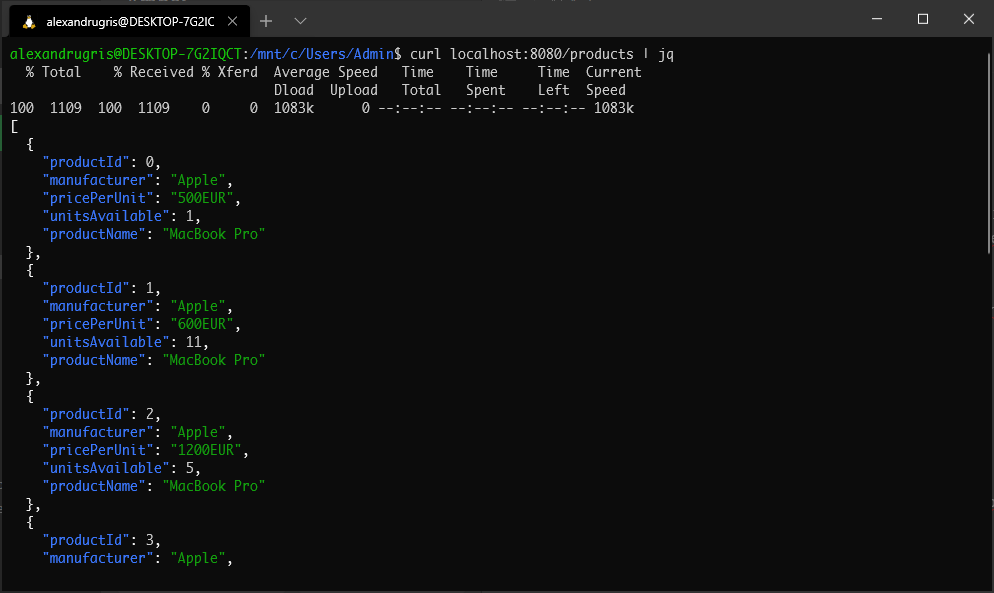To create a new product, we update the switch block from above with the following:

``````case http.MethodPost:
&io.LimitedReader{ // ensure we don't get DoS
R: r.Body,
N: 1024})

if err != nil {
log.Println(err)
return
}

product := Product{}
err = json.Unmarshal(body, &product)

if err != nil || product.ProductID != 0 {

if err == nil {
err = errors.New("ProductID should be 0 - if you know the ID, use PUT")
}

log.Println(err)
return
}

// give them an increment
// for now assume products are in incremental order, sorted
// ensure safe to this data structure
mtx.Lock()
defer mtx.Unlock()

if len(products) > 0 {
product.ProductID = products[len(products)-1].ProductID + 1
}

products = append(products, &product)
``````

To test the service we just do

``````\$curl -D - -X POST -H "Content-Type: application/json" -d '{"productId" : 0, "manufacturer": "Microsoft", "productName": "MS Surface"}' localhost:8080/products
``````

And we get the expected response back

``````HTTP/1.1 201 Created
Date: Sat, 16 Jan 2021 09:09:20 GMT
Content-Length: 0
``````

What we are going to do now is to implement `GET` for a specific product ID and `PUT` for updating a specific ID.

To do this, we need a new handler which we add to the main function. This will match the trailing `/`.

``````// handler for GET id and PUT id
http.HandleFunc("/products/", productHandler)
``````

The URLs that will go to this handler take the form `http://localhost:8080/products/id`. We are also going to structure a bit better the handler, so the error handling is factored out of the main function.

``````func productHandler(w http.ResponseWriter, r *http.Request) {

retCode := func(w http.ResponseWriter, r *http.Request) int {

pathSegments := strings.Split(r.URL.Path, "/products/")

if len(pathSegments) != 2 {
}

productID, err := strconv.Atoi(pathSegments[len(pathSegments)-1])

if err != nil {
}

product := findProductByID(productID)

if product == nil {
return http.StatusNotFound
}

switch r.Method {
case http.MethodGet:

mtx.RLock()
defer mtx.RUnlock()

jsonStr, err := json.Marshal(product)
if err != nil {
return http.StatusInternalServerError
}

w.Write([]byte(jsonStr))

return http.StatusOK

case http.MethodPut:

mtx.Lock()
defer mtx.Unlock()

R: r.Body,
N: 1024})

if err != nil || json.Unmarshal(body, &product) != nil {
}

// ensure ID stays the same
product.ProductID = productID
return http.StatusAccepted
default:
return http.StatusMethodNotAllowed
}

}(w, r)

log.Println(r.Method, r.URL.Path)

}
``````

Since we store the products in an array in memory, the find function is as simple as it gets.

``````
var mtx sync.RWMutex

func findProductByID(id int) *Product {

mtx.RLock()
defer mtx.RUnlock()

for _, p := range products {
if p != nil && p.ProductID == id {
return p
}
}
return nil
}
``````

We can test our code easily from the command line invoking

``````\$curl -D - -X GET http://localhost:8080/products/2
``````

and

``````\$curl -D - -X PUT -H "Content-Type: application/json" -d '{"productId": 0, "manufacturer": "Microsoft", "productName": "MS Surface"}' localhost:8080/products/2
``````

### Adding Middlewares - CORS Example

The http package allows for easy addition of middleware. Such middleware can do things like authentication, caching (memoizing), logging or session management. For this example, we will modify our code to add a CORS middleware.

``````func corsMiddleware(handler http.Handler) http.Handler {

return http.HandlerFunc(func(w http.ResponseWriter, r *http.Request) {

// before the handler

if r.Method == http.MethodOptions {
// the pre-flight request, make sure it is handled
return
}

// the actual handler
handler.ServeHTTP(w, r)

// after handler
})
}

func main() {

// handler for GET all and POST
http.Handle("/products", corsMiddleware(http.HandlerFunc(productsHandler)))
// handler for GET id and PUT
http.Handle("/products/", corsMiddleware(http.HandlerFunc(productHandler)))

if err := http.ListenAndServe(":8080", nil); err != nil {
log.Fatal(err)
}
}
``````

The full code, refactored with persitence in an in-memory map can be found here

### Database Access

First thing, we are going to install Postgres. Assuming docker is installed and running, we do

``````\$docker run -p 5432:5432 -e POSTGRES_PASSWORD=mysecretpassword -d postgres
``````

Now I am running the Postgres server portmapped on 5432, with usename `posgres` having the password `mysecretpassword`

To connect to the server we do

``````\$psql -p 5432 -h localhost -U postgres
``````

In the screenshot below, I have also created a database called `products` and connected to it using the `\c` command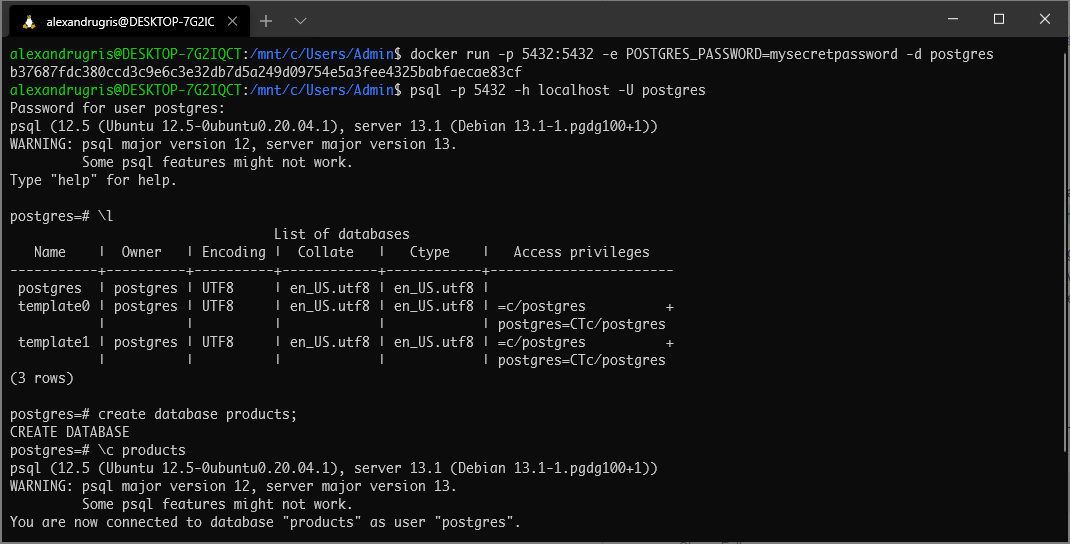The next thing to do is to get the Postgres Go driver.

``````\$go get github.com/lib/pq
``````

At the time of this writing, the recommended database driver for go is pgx. Its authors recommend to use its own API instead of the standard go SQL package due to higher performance in most Postgres-specific scenarios. For the purpose of this demo we will use the standard SQL package though, as it is portable across databases.

In a production scenario we’d also be using an external connection pooler, the recommended solution being pgbouncer. This is because for each new connection to the database server pgsql launches a new Postgres database backend, a new system process, with its launching system heavy and memory intensive.

Let’s dive into the code.

First step is to blank import the driver into our `main.go` file. That is because drivers need to register themselves with the SQL package in their `init function`(https://golang.org/doc/effective_go.html#init)

``````import _ "github.com/lib/pq"
``````

The next step is to declare a database connection pool and open it. The names are exported hence capitalized.

``````package database

import (
"database/sql"
"log"
)

// DbConn is our database connection pool
var DbConn *sql.DB

// Connect opens the connection to the database
func Connect() {
var err error
DbConn, err = sql.Open(
"postgres",
)

if err != nil {
log.Fatal(err)
}
}
``````

We are going to create the Products table and seed our database, but only if a `--dbinit` flag is sent to our executable. We add to our main function the following:

``````for _, v := range os.Args[1:] {
switch v {
case "--dbinit":
if err := database.Init(); err != nil {
log.Fatal(err)
}
}
}
``````

To create our database, we are going to play a bit with reflection and automatically discover the fields from our `Product` type. This discovery by reflection is something that all ORMs do. Since we are not going to build our own ORM here, this is the only place where we will play with reflection.

``````func Init() error {

if DbConn == nil {
errors.New("Database not opened")
}

query := "CREATE TABLE IF NOT EXISTS Products ("

t := reflect.TypeOf(product.Product{})

for i := 0; i < t.NumField(); i++ {
f := t.Field(i)
query += f.Name + " "

switch f.Type.Name() {
case "string":
query += "varchar (100)"
default:
query += f.Type.Name()
}

if i+1 < t.NumField() {
query += ", "
}
}
query += ");"
log.Println(query)

if _, err := DbConn.Exec(query); err != nil {
return err
}

if _, err := DbConn.Exec("DELETE FROM Products"); err != nil {
return err
}

if _, err := DbConn.Exec("ALTER TABLE Products ADD PRIMARY KEY (ProductID)"); err != nil {
return err
}

if _, err := DbConn.Exec(
`CREATE SEQUENCE IF NOT EXISTS pk_product
CACHE 100 OWNED BY Product.ProductID`); err != nil {
return err
}

return nil
}
``````

The next step is to implement the full `product.Map` interface and switch from an in-memory map to database calls. A better name would have been `product.Repository` but we will not refactor the code now. The full code can be found here and we are only going to exemplify in this blogpost how to create a new product.

``````func (m *mapInternal) CreateNew(p *Product) {

stmt, err := database.DbConn.Prepare(`INSERT INTO
Products(Manufacturer, PricePerUnit, UnitsAvailable, ProductName, ProductID)
VALUES (\$1, \$2, \$3, \$4, nextval('pk_product')) RETURNING ProductID`)

if stmt == nil || err != nil {
log.Fatal(err)
}

sqlRow := stmt.QueryRow(p.Manufacturer, p.PricePerUnit, p.UnitsAvailable, p.ProductName)

if err := sqlRow.Scan(&p.ProductID); err != nil {
log.Fatal(err)
}
}
``````

The full source code for this implementation can be found here.

### Contexts

If we want to setup a timeout for a query, golang provides the `Context` mechanism. Each database function has a `Context` method. The call to `cancel()` when the operation is completed successfully allows to end the context and release all associated resources.

An example below:

``````func (m *mapInternal) GetAll() []*Product {

// this will allow the queries to timeout
ctx, cancel := context.WithTimeout(context.Background(), 15*time.Second)
defer cancel()

results, err := database.DbConn.QueryContext(ctx, `
SELECT
ProductID,
Manufacturer,
PricePerUnit,
UnitsAvailable,
ProductName
FROM Products
`)

if err != nil {
log.Println(err)
return nil
}

defer results.Close()

ret := make([]*Product, 0, 100)

for results.Next() {
v := Product{}

results.Scan(
&v.ProductID,
&v.Manufacturer,
&v.PricePerUnit,
&v.UnitsAvailable,
&v.ProductName)

ret = append(ret, &v)
}

return ret
}
``````
]]>
First Steps In Go2021-01-09T08:15:16+01:002021-01-09T08:15:16+01:00https://alexandrugris.github.io/programming/2021/01/09/golang-first-stepsMy first steps in Go, largely based on the Golang tutorial and Internet side searches.

### Hello World

Building a very basic hello world project once the go tools are installed is straight forward:

• Create a folder named “helloworld”
• `cd ./helloworld`
• Create a file called `main.go`
• Add the following code into the file
• Save and at the command prompt type `go build`. An executable file called `helloworld` will be compiled into the folder.
``````package main

import (
"fmt"
)

func main() {
fmt.Println("Hello World")
}
``````

A good editor for `go` is Visual Studio Code.

### Language Basics

The most basic unit of organizing code in go is the function. Below is an example of a function with several parameters, one of each being a callback (function pointer).

``````// function receiving function as a parameter
func arrayOpScalar(array []float32, constant float32, operation func(float32, float32) float32) {
for i := range array {
array[i] = operation(array[i], constant)
}
}
``````

A function can be passed as a parameter, assigned to a variable or returned from another function.

``````// use the arrayOpScalar function defined above to
// create another function to double the values in an array
doubleFn := func(array []float32) {
arrayOpScalar(array, 2, func(x, y float32) float32 { return x * y })
}

doubleFn(p)
``````

But before we do that, let’s look a bit at arrays and slices. Allocating an array goes like this

``````primes := int{2, 3, 5, 7, 11, 13}
``````

The length is part of the array so it cannot be resized. Slices are views onto arrays, so when a value is modified on the slice it will automatically propagate to the backing array.

``````var s []int = primes[1:4]
``````

The internal structure of a slice is as follows:

``````type slice struct {
array *T,
len int,
cap int,
}
``````

Length and capacity can be accessed through `len()` and `cap()`. Therefore, in golang you can do very cool stuff such as converting from a struct to its underlying byte representation. Such operations are useful when, for instance, memory mapping files to arrays of a specified stucture without additional serialization / deseralization. Here for an extended thread

``````type Struct struct {
p1 int32
p2 int32
p3 uint16
p3 uint16
}

// read in a compile-time constant the size of the struct
const sz = int(unsafe.SizeOf(Struct{}))

// initialize convert the pointer to the struct to an array of bytes
// of the same size as the struct and take a slice to it.
var asByteSlice []byte = (*(*[sz]byte)(unsafe.Pointer(&struct_value)))[:]
``````

Slices can contain other slices.

``````mat3x3 := [][]float32{
[]float32{1.0, 0.0, 0.0},
[]float32{0.0, 1.0, 0.0},
[]float32{0.0, 0.0, 1.0},
}
// elements can be accessed
fmt.Println(board)
``````
``````// dynamic allocation of an array of 10 floats
p := make([]float32, 10)

// dynamically growing the array by appending
// 10 elements with spread operator
p = append(p, make([]float32, 10)...)

// looping over indexes in p
for i := range p {
p[i] = float32(i)
}
``````

Let’s look also at static initialization.

``````slice := []struct { // annonymous struct of two integers
i1 int
i2 int
}{ // statically initialized
{0, 0},
{1, 1},
{2, 2}, // comma at the end is mandatory
}

for _, x := range slice {
fmt.Printf("%v : %v\n", x.i1, x.i2)
}
``````

### Custom Types

In `go`, encapsulation is defined at the package level. Everything in a package is public. Exported symbols start with capital letter, everything starting with lowercase is private outside of the package. Let’s define a custom type.

``````type Vertex struct {
X float64
Y float64
Z float64
}
``````

Initializing a variable of such a type goes like this:

``````v := Vertex{0.1, 0.2, 0.3}
``````

or

``````v := Vertex {
X : 0.1,
Y : 0.2,
Z : 0.3,
}
``````

We can return a pointer of such a struct. By default the compiler will favor stack allocation, but it does perform escape analysis and, in case the lifetime of an object cannot be determined at compile time it will switch to allocating it on the heap.

``````func returnPointerToVertex() *Vertex {
return &Vertex{1.0, 2.0, 3.0}
}
``````

Now let’s add some methods to the type.

``````// Length computes the vector norm
func (v Vertex) Length() float64 {
return math.Sqrt(v.X*v.X + v.Y*v.Y + v.Z*v.Z)
}
``````

Methods with pointer receivers can modify the value to which the receiver points (as scale does here). ince methods often need to modify their receiver, pointer receivers are more common than value receivers. There are two reasons to use a pointer receiver:

• The first is so that the method can modify the value that its receiver points to.
• The second is to avoid copying the value on each method call

In general, all methods on a given type should have either value or pointer receivers, but not a mixture of both.

``````// Scale scales the vector by a float
func (v *Vertex) Scale(s float64) {

// unlike C++ where invoking a method on a nullptr usually results in a crash
// in golang this is perfectly acceptable
if v == nil {
return
}

v.X *= s
v.Y *= s
v.Z *= s
}
``````

Speaking of types, golang does not allow inheritance, but it does have the interface type.

``````type Scaler interface {
Scale(float64)
}
``````

Vector automatically implements this interface by simply implementing the respective methods. Now we can do

``````v := Vertex{X: 0.1, Y: 0.2, Z: 0.3}

var scaler Scaler = &v
scaler.Scale(10.0)
``````

Beside interfaces that have functions, go offers the empty interface as a method to hold a variable of any type. Any object can be assigned to the empty interface, including the scalar types. Here is an example:

``````// empty interface
var intf interface{} = "Hello World"

// querying the empty interface for the underlying type
if s, ok := intf.(string); ok {
fmt.Println(s)
}

// i := intf(float32) would panic
// need to test of OK
if i, ok := intf.(float32); ok {
fmt.Println(i)
}

// a better way is to test with a type switch
// interesting is that v is the value converted to the type, not the type
switch v := intf.(type) {
case string:
fmt.Println("It's a string!", v)
case int:
fmt.Println("It's an int!", v)
case float32:
fmt.Println("It's a float!", v)
}
``````

When it comes to interfaces, golang offers a very elegant solution to encapsulation and type aggregation. It reminds me of power of `IUnknown::QueryInterface()` from `COM`, but embedded in the language itself. It relies on type assertions and embedded types.

``````package main

/*Beautiful method for embedding types and exposing interfaces in Golang. */

import (
"fmt"
"unsafe"
)

type Writer interface{
Write(string)
}

}

Writer
}

type rwImplType struct {
str string
}

func (rw *rwImplType) Read() string {
// same underlying pointer
fmt.Println(unsafe.Pointer(rw))
return rw.str
}

func (rw* rwImplType) Write(msg string){
// same underlying pointer
fmt.Println(unsafe.Pointer(rw))
fmt.Printf("%v: %v\n", rw.str, msg)
}

func main() {

// Instatiante a concrete implementation
rwImpl := rwImplType{str: "Hello World"}

// expose it in an aggregate public interface which
// implements several interfaces
Writer: &rwImpl, // can be another implementation
}

var anon interface{} = rwIntf

// QueryInterface()
w := anon.(Writer)

// works like a charm :)
}
``````

Speaking of the `switch` construct, it is quite flexible:

``````// switch
// with declaration and condition
switch os := runtime.GOOS; os {
case "linux":
fallthrough
case "windows", "darwin":
fmt.Printf("Running on %v\n", os)
default:
fmt.Println("Unknown")
}

// with no condition
switch {
case time.Now().Weekday().String() == "Thursday":
fmt.Println("Today is Thursday")
default:
fmt.Println("Today is not Thursday")
}
``````

### Maps

Maps can be initialized as literals or created dinamically with `make`

``````
// dynamic instantiation
m := make(map[string]Vertex, 10)
m["Iasi"] = Vertex{1.0, 1.0, 1.0}

// check for existence of an element
if _, exists := m["Cluj"]; !exists {
fmt.Println("Cluj does not exist in the map")
}

fmt.Println(m["Iasi"].Length())

// literal instantiation
m1 := map[string]Vertex{
"Iasi":      {1.0, 1.0, 1.0}, // no need to specify Vertex
"Bucharest": {2.0, 2.0, 2.0},
}

// map can be increased
m1["Cluj"] = Vertex{3.0, 3.0, 3.0}

fmt.Println(m1)

// remove the element from the map
delete(m1, "Cluj")

// or also literal instantiation but with no elements
counts := map[string]int{}
``````

### Sample Programs

Fibonnaci - function returning a function

``````import "fmt"

// fibonacci is a function that returns
// a function that returns an int.
func fibonacci() func() int {

// declaration - initialization
first, second := 0, 1

return func() int {
ret := first + second
first, second = second, ret
return ret
}

}

func main() {
f := fibonacci()
for i := 0; i < 10; i++ {
fmt.Println(f())
}
}
``````

Error management and the Error interface:

``````package main

import (
"fmt"
"math"
)

type ErrNegativeSqrt float64

func (v ErrNegativeSqrt) Error() string {
if v < 0.0 {
return fmt.Sprintf("Negative sqrt %v", float64(v))
}
return ""
}

func Sqrt(x float64) (float64, error) {

if x < 0.0{
return 0.0, ErrNegativeSqrt(x)
}

z := 1.0
delta := z * z - x

for math.Abs(delta) > 1e-10{
z -= delta / (2.0 * z)
delta = z * z - x
}

return z, nil
}

func main() {
if v, err := Sqrt(-2); err == nil {
fmt.Println(v)
} else {
fmt.Println(err)
}
}
``````

Reader implementation. An in-memory stream obtained from a string can be created with `r := strings.NewReader("Hello, Reader!")`

``````package main

import (
"io"
"os"
"strings"
)

}

// returns the number of elements read
// and an error if an error occured
// the error can be io.EOF which signifies the end of the stream

for i := 0; i < n; i++{
switch {
case b[i] >= 'A' && b[i] <= 'Z':
b[i] = (b[i] - 'A' + 13) % 26 + 'A'
case b[i] >= 'a' && b[i] <= 'z':
b[i] = (b[i] - 'a' + 13) % 26 + 'a'
}
}

return n, err
}

func main() {
s := strings.NewReader("Lbh penpxrq gur pbqr!")
io.Copy(os.Stdout, &r)
}
``````

### Concurrency

Concurrency in go is achieved through goroutines. Goroutines are language constructs which maps M virtual threads to N CPU threads. The runtime has its own scheduler. The preferred way of of sharing state is through channels, although shared memory is also possible thanks to the `sync` standard package. Let’s look at two programs below.

The first program compares two BSTs.

``````package main

import (
"golang.org/x/tour/tree"
"fmt"
)

// Walk DFSes the tree t sending all values
// to the channel ch.
func Walk_(t *tree.Tree, ch chan int){

if t.Left != nil{
Walk_(t.Left, ch)
}

// send the current value to the channel
ch <- t.Value

if t.Right != nil {
Walk_(t.Right, ch)
}
}

func Walk(t *tree.Tree, ch chan int){
Walk_(t, ch)

// close the channel to signal the end of the tree
close(ch)
}

// Same determines whether the trees
// t1 and t2 contain the same values.

func Same(t1, t2 *tree.Tree) bool{

// make two channels
c1 := make(chan int)
c2 := make(chan int)

// launch the two walks in parallel
go Walk(t1, c1)
go Walk(t2, c2)

// Read one value at a time from each channel
// and compare them
for ok1, ok2 := true, true; ok1 && ok2;  {
var v1, v2 int

// when one channel is closed, its OK value is set to false
v1, ok1 = <- c1
v2, ok2 = <- c2

if ok1 != ok2 || v1 != v2{
return false
}

}
return true
}

func main() {
if Same(tree.New(1), tree.New(1)) {
fmt.Println("Same tree")
} else {
fmt.Println("Not the same tree")
}
}
``````

Notes:

• A channel cannot be closed twice
• A write from a closed channel results in a panic
• You can check on read if the channel is closed
• Channel operations are blocking. A channel can have a buffer, in which condition the operation becomes blocking only when the buffer is full
• A channel can be read with a `range` construct. The range finishes when the sender closes the channel

The second program, also part of the golang tour, introduces `sync.WaitGroups` to allow waiting for goroutines to finish as well as sending return channels through input channels for safe reply. To allow for concurrent access, the `Cache` is implemented as a process (actor) which is accessible only through its input and output channels.

We are going to send a pair to our cache service, `<string - key, return channel>`. The return channel solves a concurrency issue: assuming that we have more concurrent readers waiting, we want to ensure we return the result to the reader that sent the message. Since in our case we use a non-buffered write channel, all writes are blocked until a new read is performed and, since the cache is a single threaded, it will not make a new read until the result is communicated, we could have used a single return channel for all the cache requests. However, if we make the write channel buffered, thus allowing for multiple writes, the returns will be mixed.

``````package main

import (
"fmt"
"sync"
)

// the wait group is needed to allow all goroutines
// to signal when they finish execution
// and the main goroutine to wait for them
var wg sync.WaitGroup

type Fetcher interface {
Fetch(url string) (body string, urls []string, err error)
}
// message including the return channel
type CacheMsg struct {
str string
out chan bool
}

type Cache struct {
in chan CacheMsg
}

func (p *Cache) Init(){
p.in = make(chan CacheMsg)
go p.cache()
}

func (p *Cache) Test(s string) bool {

// create a new return channel for each service request
msg := CacheMsg {
str: s,
out: make(chan bool),
}

p.in <- msg
return <- msg.out
}

func (p *Cache) cache(){

// our cache map
cache := make(map[string]bool)

// read messages with range until the channel is closed
for msg := range p.in {
if _, exists := cache[msg.str]; exists {
msg.out <- true
} else {
cache[msg.str] = true
msg.out <- false
}
}
}

func Crawl(url string, depth int, fetcher Fetcher, cache *Cache) {

// ensure we call wg.Done() when the method exits
defer wg.Done()

if depth <= 0 {
return
}

// the url is already in the cache
if cache.Test(url) {
return
}

body, urls, err := fetcher.Fetch(url)
if err != nil {
fmt.Println(err)
return
}
fmt.Printf("found: %s %q\n", url, body)

// add N new goroutines to the WaitGroup
for _, u := range urls {

// launch crawl goroutines in parallel
go Crawl(u, depth-1, fetcher, cache)
}
return
}

func main() {

var cache Cache
cache.Init()

Crawl("https://golang.org/", 4, fetcher, &cache)
// wait for all goroutines to finish
wg.Wait()
}

// fakeFetcher is Fetcher that returns canned results.
type fakeFetcher map[string]*fakeResult

type fakeResult struct {
body string
urls []string
}

func (f fakeFetcher) Fetch(url string) (string, []string, error) {
if res, ok := f[url]; ok {
return res.body, res.urls, nil
}
}

// fetcher is a populated fakeFetcher.
var fetcher = fakeFetcher{
"https://golang.org/": &fakeResult{
"The Go Programming Language",
[]string{
"https://golang.org/pkg/",
"https://golang.org/cmd/",
},
},
"https://golang.org/pkg/": &fakeResult{
"Packages",
[]string{
"https://golang.org/",
"https://golang.org/cmd/",
"https://golang.org/pkg/fmt/",
"https://golang.org/pkg/os/",
},
},
"https://golang.org/pkg/fmt/": &fakeResult{
"Package fmt",
[]string{
"https://golang.org/",
"https://golang.org/pkg/",
},
},
"https://golang.org/pkg/os/": &fakeResult{
"Package os",
[]string{
"https://golang.org/",
"https://golang.org/pkg/",
},
},
}
``````

The implementation above is more generic as it can be used as a pattern for other kinds of services. In our case, a faster solution would have been to use shared memory protected through a `sync.Mutex`, `sync.RWMutex` or through a `sync.Map`, a concurrent map.

One thing to note - altough all IO operations in go are blocking the current goroutine, the are implemented as asyncio behind the scenes, in a similar manner to which the `cache.Test()` method above is blocking.

### Timers and select

Select allows to listen to multiple channels and block until one of them has data available. Timers in golang are implemented as channels. Signaling to a goroutine to finish its job can be done also though a channel.

``````package main

import (
"fmt"
"sync"
"time"
)

func main() {

seconds := time.NewTicker(time.Second)
minutes := time.NewTicker(time.Minute)

done := make(chan bool)

wg := sync.WaitGroup{}

go func() {
for {
select {
case <-done:
wg.Done()
return // exit the routine
case <-seconds.C:
fmt.Println("Tick")
case <-minutes.C:
fmt.Println("Tock")
}
}
}() // immediately invoked goroutine

time.Sleep(time.Minute * 3)
done <- true

wg.Wait()
fmt.Println("Done.")
}
``````

### Conclusion

Go is a very beautiful and performant language. It is low level enough to feel like you have power you have in C and it compiles to native code for super fast startup times, performance and interoperability. It is elegant as it does not have unnecessary constructs yet, though its constructs, it encourages at the language level clean code and excellent concurrency.

]]>
Programming Problems In C++2020-10-24T09:15:16+02:002020-10-24T09:15:16+02:00https://alexandrugris.github.io/programming/2020/10/24/programming-problems-cppThis post is a collection of several programming problems implemented in C++. It’s good to keep the edge sharp by solving some algorithmic problems from time to time.

Here are all the problems running in the same executable file: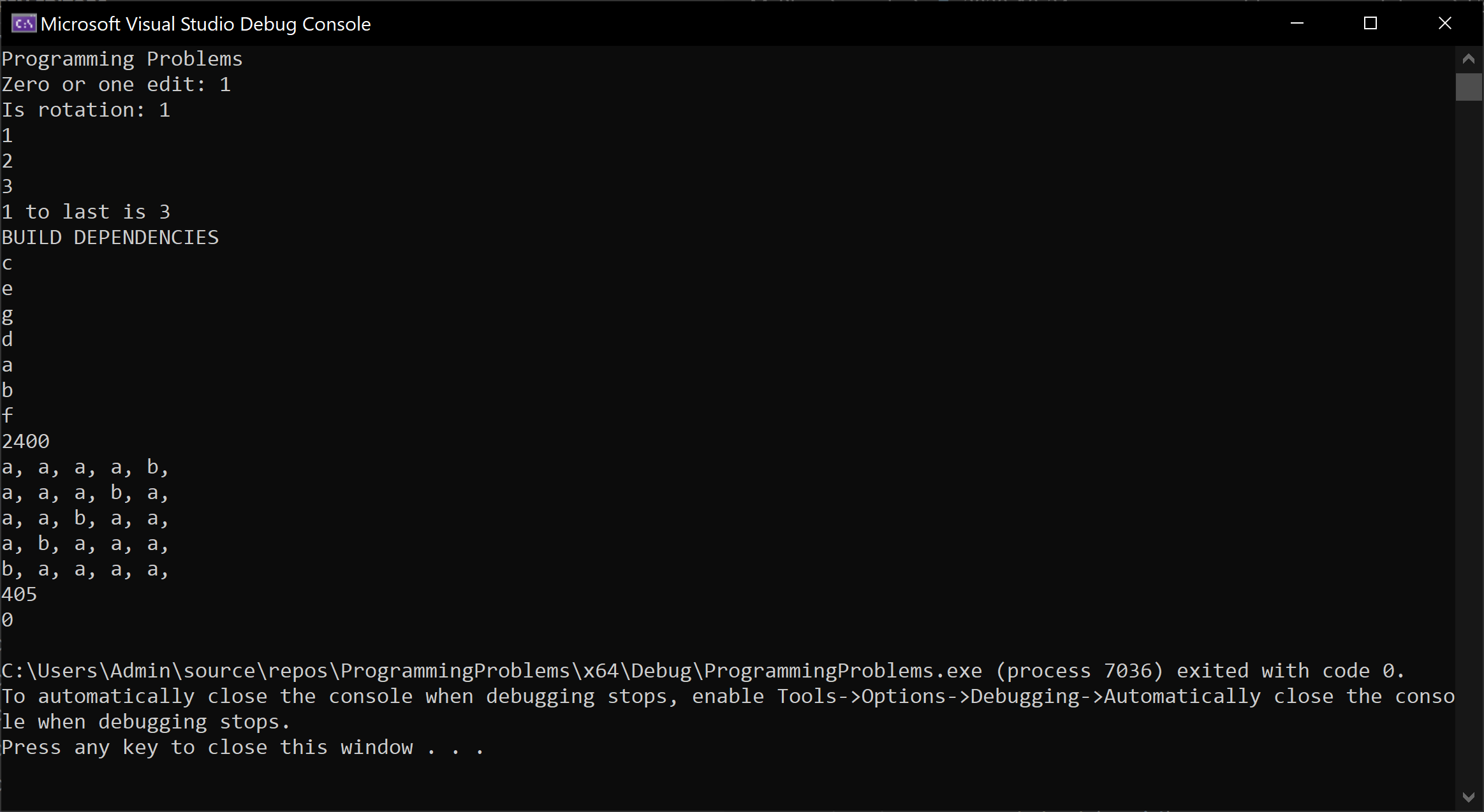### Problem 1 - Zero Or One Edits Away

Write an algorithm that will return true if a string is zero or one edits away from another string. An edit is a letter changed or deleted. The algorithm should be invoked as:

``````cout << "Zero or one edit: " << zero_one_edits_away("hello", "hello") << endl;
``````

or

``````cout << "Zero or one edit: " << zero_one_edits_away("helo", "hello") << endl;
``````

and both should return true.

The solution below:

``````using namespace std;

bool zero_one_edits_away(const string& s1, const string& s2) {

int l1 = (int)s1.length();
int l2 = (int)s2.length();

if (abs(l1 - l2) > 1)
return false;

int edit_count = 0;

for (int i = 0, j = 0; i < l1 && j < l2; i++, j++) {
if (s1[i] == s2[j])
continue;

if (edit_count == 1) return false;
else edit_count++;

if (l1 == l2) continue;
else if (l1 < l2) j++; // it can only be a delete
else i++;
}

return true;
}
``````

### Problem 2 - String Rotation

Write an algorithm that, given two strings, will return true if one string is a rotation of the other one and false otherwise. The algorithm should be invoked as:

``````cout << "Is rotation: " << is_rotation("wwaterbottleww", "bottlewwwwater") << endl;
``````

and in the case above should return true. The solution written below:

``````bool is_rotation(const string& s1, const string& s2) {

if (s1.length() != s2.length()) return false;

int start_of_match = -1;
unsigned i = 0;
unsigned j = 0;

for (j = 0; j < s2.length(); j++) {
if (s1[i] != s2[j]) {
if (start_of_match != -1) {
j -= (j - start_of_match);
}
start_of_match = -1;
i = 0;
continue;
}
else if (start_of_match == -1)
start_of_match = j;
i++;
}

// last part of the string matched
if (j == s2.length() && start_of_match == -1)
return false;

// check the first part
const char* cs1 = s1.c_str();
const char* cs2 = s2.c_str();

return strncmp(cs1 + i, cs2, start_of_match) == 0;
}
``````

### Problem 3 - Remove Duplicates

Given a list of of numbers, remove duplicates without breaking the order in the list. The algorithm should be invoked as:

``````auto lst = remove_duplicates({ 1, 1, 2, 2, 3, 3, 1, 1, 1, 2 });
for (auto l : lst) {
cout << l << ", ";
}
``````

and it should print `1, 2, 3`.

The constraint to not break the order does not allow us to sort the list before removal of the duplicates, which forces us into a `O(n^2)` solution. We are going to use the `std::list` structure which allows in-place removal of an element without breaking the iterators. Since `std::list` is doubly linked, another slightly lighter solution could involve the `std::forward_list`:

``````
auto remove_duplicates(const std::initializer_list<int>& l) {

list<int> lst = l;

for (auto i = lst.begin(); i != lst.end(); i++) {
auto j = i;
j++;
for (;j != lst.end();) {
auto tmp = j++;
if (*i == *tmp) {
lst.erase(tmp);
}
}
}
return lst;
}
``````

### Problem 4 - K-to-last

Write an algorithm which prints the k-to-last element in a singly linked list. Since we don’t know the number of elements in the list, nor can we traverse it backwards, we need to parse the whole list and keep two pointers at k elements apart. The algorithm should be invoked as:

``````int n = -1;
int k = 1;
if (k_to_last({ 1, 2, 3, 4 }, k, n)) {
cout << k << " to last is " << n << endl;
}
else {
cout << "k > length(array)" << endl;
}
``````

The solution:

``````bool k_to_last(const std::initializer_list<int>& l, const int k, int& ret) {
forward_list<int> lst = l;

auto it1 = lst.begin();
auto it2 = lst.begin();
const int k_upd = k + 1;

int i = 0;
for (; it1 != lst.end() && i < k_upd; i++, it1++);

if (it1 == lst.end() && i < k_upd)
return false; // not enough elements

for (; it1 != lst.end(); it1++, it2++);

ret = *it2;
return true;
}
``````

### Problem 5 - Build Dependencies

Given a list of builds, with dependencies between each other, write a program that finds the right compilation order such that each dependency is compiled before its dependents. Here is the example invocation, with first build depending on the builds sent as the second parameter.

``````cout << "BUILD DEPENDENCIES" << endl;

map<char, list<char>> dependencies;

dependencies.emplace('a', initializer_list<char>({ 'd' }));
dependencies.emplace('f', initializer_list<char>({ 'b', 'a', 'e'}));
dependencies.emplace('b', initializer_list<char>({ 'd' }));
dependencies.emplace('d', initializer_list<char>({ 'c' }));
dependencies.emplace('g', initializer_list<char>({ }));

make_builds(dependencies);

try {
for (auto l : build_dependencies()) {
cout << l << endl;
}
}
catch (const char* exx) {
cout << exx << endl;
}

clear_builds();
``````

In this case, the printed solution should be:

``````BUILD DEPENDENCIES
c
e
g
d
a
b
f
``````

The algorithm below:

``````enum BuildState {
NOT_TOUCHED = 0,
UNDER_CHECK = 1,
CAN_BE_BUILT = 2
};

struct build {

build(char _id) :
id(_id),
build_state(NOT_TOUCHED)
{
}

char id;
BuildState build_state;
list<build*> dependencies;

};

map<char, build*> builds;

void make_builds(const map<char, list<char>>& prjs) {

for (auto p : prjs) {
auto it = builds.find(p.first);
if (it == builds.end())
it = builds.emplace(p.first, new build(p.first)).first;

for (auto d : p.second) {

auto dps = builds.find(d);
if (dps == builds.end())
dps = builds.emplace(d, new build(d)).first;

it->second->dependencies.push_back(dps->second);

}
}
}

void clear_builds() {
for (auto b : builds) {
auto* tmp = b.second;
b.second = nullptr;
delete tmp;
}
builds.clear();
}

list<char> build_dependencies(const list<build*>& projects) {

list<char> ret;

// start building in parallel those that don't have dependencies
for (auto& p : projects) {
if (p->build_state == CAN_BE_BUILT)
continue;

if (p->build_state == UNDER_CHECK)
throw "cannot build - circular dependencies";

if (p->dependencies.size() == 0) {
p->build_state = CAN_BE_BUILT;
ret.push_back(p->id);
}
}

// finish with the rest of them
for (auto& p : projects) {

if (p->build_state == CAN_BE_BUILT)
continue;

if (p->build_state == UNDER_CHECK)
throw "cannot build - circular dependencies";

p->build_state = UNDER_CHECK;

for (auto& d : p->dependencies) {
auto lst = build_dependencies(p->dependencies);
copy(lst.begin(), lst.end(), back_inserter(ret));
}

p->build_state = CAN_BE_BUILT;
ret.push_back(p->id);

}

return ret;
}

list<char> build_dependencies() {

list<build*> prjs;
for (auto b : builds)
prjs.push_back(b.second);

return build_dependencies(prjs);
}
``````

### Problem 6 - Recursive Multiply

Write a program that performs multiplication without using the `*` symbol while minimizing the number of operations. The only allowed operators are `+`, `-`, `<<` and `>>`.

``````// recursive multiply without using *, just +, -, <<, >>
cout << recursive_multiply(100, 24);
``````

The solution below:

``````int recursive_multiply(int a, int b) {

// take the largest number to add
if (a < b)
swap(a, b);

int sum = a;
int b_first = b;
int i = 0;

for (; b > 1; b = b >> 1, i++)
sum += sum;

b = b_first - (1 << i);

if (b > 1)
sum += recursive_multiply(a, b);
else if (b == 1)
sum += a;

return sum;
}
``````

### Problem 7 - All Permutations, No Duplicates

Given a string, write all possible permutations of that string elimiating duplicates. For instance, for the following invocation,

``````// permutations without duplicates
all_permutations_no_duplicates("aaaab");
``````

the printed solution should be:

``````a, a, a, a, b,
a, a, a, b, a,
a, a, b, a, a,
a, b, a, a, a,
b, a, a, a, a,
``````

The solution involves keeping the count of each duplicated letter so we don’t include it as a different symbol for each permutation:

``````void all_permutations_no_duplicates(vector<char> &current,  vector<char>& str, vector<int> &counts) {
bool any = false;

// can be done with forward_list<> insert and erase @ it
for (int i = 0; i < str.size(); i++) {
if (counts[i] > 0) {
current.push_back(str[i]);
counts[i] --;
all_permutations_no_duplicates(current, str, counts);
counts[i] ++;
current.pop_back();
any = true;
}
}

if (!any) {
for (auto c : current)
cout << c << ", ";

cout << endl;
};
}

void all_permutations_no_duplicates(const string& str_) {
vector<char> v;
vector<int> cnts;
vector<char> c;

string str = str_;

sort(str.begin(), str.end());

// build counts
char prev_c = 0;
for (auto c : str) {
if (prev_c != c) {
v.push_back(c);
cnts.push_back(1);
}
else {
cnts[cnts.size() - 1] ++;
}
prev_c = c;
}

cout << endl;
all_permutations_no_duplicates(c, v, cnts);
}
``````

### Problem 8 - Stacks of Boxes

Given a list of boxes, find the highest tower that can be built by stacking boxes on top of each other. A box can be stacked on top of another box only if all its dimensions, width, depth and height, are smaller than those of the box below.

For solving the problem we will start by generating an array of 100 boxes, all with randomly generated dimensions.

The algorithm is started by:

``````cout << stack_of_boxes() << endl;
``````

The solution below:

``````struct Box {
Box(int w_, int l_, int h_) : w(w_), l(l_), h(h_){
}

int w, l, h;

bool sorter(const Box& b) const {
return w > b.w;
}

bool can_stack(const Box& b) const {
return w > b.w && h > b.h && l > b.l;
}
};

struct Stack {

Stack(int b, int h, shared_ptr<Stack> next_) : base_box(b), height(h), next(next_) {}

int base_box;
int height;
shared_ptr<Stack> next;
};

int stack_sorted_boxes(
list<shared_ptr<Stack>>& s,
const map<int,
vector<int>>& can_stack,
const vector<Box> &v) {

int max_height = 0;

// it is basically a deque
for (auto i = s.begin(); i != s.end();) {

auto stackables = can_stack.find((*i)->base_box);

// cannot stack anything on top, this is the end of the stack
if (stackables == can_stack.end()) {
if (max_height < (*i)->height)
max_height = (*i)->height;
}
else {
for (auto j : stackables->second) {
// here we don't really need the last parameter
// if we are only returning the stack height and not the stask itself
s.push_back(make_shared<Stack>(j, (*i)->height + v[j].h, *i));
}
}
i = s.erase(i); // I already put something on top of it

}
return max_height;
}

int stack_of_boxes() {

vector<Box> v;

// randomly generate 100 boxes
for (int i = 0; i < 100; i++) {
v.emplace_back(rand() % 100, rand() % 100, rand() % 100);
}

sort(v.begin(), v.end(), [](const Box& b1, const Box& b2) {
return b1.sorter(b2);
});

map<int, vector<int>> can_stack;

// generate a list of boxes that can be stacked upon each other
for(int i = 0; i < v.size(); i++)
for (int j = i; j < v.size(); j++) {
if (v[i].can_stack(v[j]))
can_stack[j].push_back(i);
}

list<shared_ptr<Stack>> stacks;

for (int i = 0; i < v.size(); i++) {
stacks.push_back(make_shared<Stack>(i, v[i].h, shared_ptr<Stack>()));
}

return stack_sorted_boxes(stacks, can_stack, v);
}
``````

### Problem 9 - Expression Equivalence

Given two expressions, `a * (b + c)` and `a * b + a * c`, write an algorithm that will determine if the two expressions are equivalent. In our case above, the two expressions are equivalent.

The solution implies expanding the parantheses and bringing the expression to a cannonical form, a sum of products. To shortcut the parsing, we will consider the expression is given in the form of an expression tree, `(left operand, operation, right operand)`. After the expression is brought to the cannonical form, each term is sorted alphabetically and then all all terms are again sorted. Then we simply compare the strings in order to decide whether the two expressions are equivalent.

The algorithm is started by defining expressions as follows:

``````ExprTree e1(
'*',
new ExprTree('*', new ExprTree('+', 'a', 'b'), new ExprTree('a')),
new ExprTree('*', new ExprTree('+', 'a', 'b'), new ExprTree('a'))
);

ExprTree e2(
'*',
new ExprTree('+', 'a', 'b'),
new ExprTree('+', 'a', 'b')
);

ExprTree e3(
'*',
new ExprTree(
'*',
new ExprTree('+', 'a', 'b'),
new ExprTree('+', 'a', 'b')
),
new ExprTree(
'*',
new ExprTree('+', 'a', 'b'),
new ExprTree('+', 'a', 'b')
)
);

// or creating a random tree for faster testing
ExprTree* e4 = ExprTree::make_random_tree();
cout << expression_equivalence(e4, &e3) << endl;

delete e4
``````

As a complication, the algorithm must not generate any memory leaks and must use C-style pointers.

Below is the full solution:

``````struct ExprTree {

ExprTree(char _op, ExprTree* _left = nullptr, ExprTree* _right=nullptr) :
op(_op), left(_left), right(_right){
}

ExprTree(char _op, char a, char b) {
op = _op;
left = new ExprTree(a);
right = new ExprTree(b);
}

~ExprTree() {
if(left != nullptr)
delete left;

if(right != nullptr)
delete right;
}

ExprTree* deep_copy() {

return new ExprTree(op,
left ? left->deep_copy() : nullptr,
right ? right->deep_copy() : nullptr);
}

// utility function to generate a random tree
// not part of the algorithm
static ExprTree* make_random_tree() {

float f = ((float)rand() / (float)RAND_MAX);

if(f < 0.3)
return new ExprTree('a');

if (f < 0.6)
return new ExprTree('b');

if (f < 0.8)
return new ExprTree('*', make_random_tree(), make_random_tree());

return new ExprTree('+', make_random_tree(), make_random_tree());

}

char op;
ExprTree* left;
ExprTree* right;
};

void expand_paranthesis(ExprTree* start) {

if (start->op != '+' && start->op != '*')
return; // terminal symbol

// to bubble up the +'es. we cannot have a * above a +
expand_paranthesis(start->left);
expand_paranthesis(start->right);

if (start->op == '*') {

auto tmpop = start->op;

// (a + b) * (a + b)
if (start->right->op == '+' && start->left->op == '+') {

start->op = '+';

auto tmp_left_a = start->left->left;
auto tmp_right_a = start->right->left;
auto tmp_left_b = start->left->right;
auto tmp_right_b = start->right->right;

start->left = new ExprTree('+',
new ExprTree('*', tmp_left_a, tmp_right_a),
new ExprTree('*', tmp_left_a->deep_copy(), tmp_right_b));

start->right = new ExprTree('+',
new ExprTree('*', tmp_left_b, tmp_right_a->deep_copy()),
new ExprTree('*', tmp_left_b->deep_copy(), tmp_right_b->deep_copy()));
}
// a * (b + c)
else if (start->right->op == '+') {
start->op = '+';
auto deep_copy_left = start->left->deep_copy();
start->left = new ExprTree('*', start->left, start->right->left);
start->right = new ExprTree('*', deep_copy_left, start->right->right);
}

//(b + c) * a
else if (start->left->op == '+') {
start->op = '+';
auto tmp = start->left;
auto start_right_copy = start->right->deep_copy();
start->left = new ExprTree('*', tmp->left, start->right);
start->right = new ExprTree('*', tmp->right, start_right_copy);
}

// what has been arranged before is
// no longer arranged because we changed the structure of the tree
if (tmpop != start->op) {
expand_paranthesis(start->left);
expand_paranthesis(start->right);
}
}
}

void get_set(ExprTree* e, vector<char>& s) {
if (e->op == '*') {
get_set(e->left, s);
get_set(e->right, s);
}
else {
s.push_back(e->op);
}
}

void to_sorted_vector(ExprTree* e, vector<string> & ret) {

if (e->op == '+') {
to_sorted_vector(e->left, ret);
to_sorted_vector(e->right, ret);
}
else if (e->op == '*') {
vector<char> v;

get_set(e->left, v);
get_set(e->right, v);

string s;
sort(v.begin(), v.end());
copy(v.begin(), v.end(), back_inserter(s));
ret.push_back(s);
}
}

bool expression_equivalence(ExprTree* e1, ExprTree* e2) {

expand_paranthesis(e1);
expand_paranthesis(e2);

// each term is sorted alphabetically
vector<string> e1_str;
to_sorted_vector(e1, e1_str);

vector<string> e2_str;
to_sorted_vector(e2, e2_str);

// all terms are sorted alphabetically
sort(e1_str.begin(), e1_str.end());
sort(e2_str.begin(), e2_str.end());

auto it1 = e1_str.begin();
auto it2 = e2_str.begin();

// simple pairwise comparison
for (; it1 != e1_str.end() && it2 != e2_str.end(); it1++, it2++) {
if (*it1 != *it2) return false;
}

return it1 == e1_str.end() && it2 == e2_str.end();
}
``````

Below you can see the algorithm running in Visual C++ with the expression expanded. You can notice that all `+`-es are above `*` in the reorganized expression tree.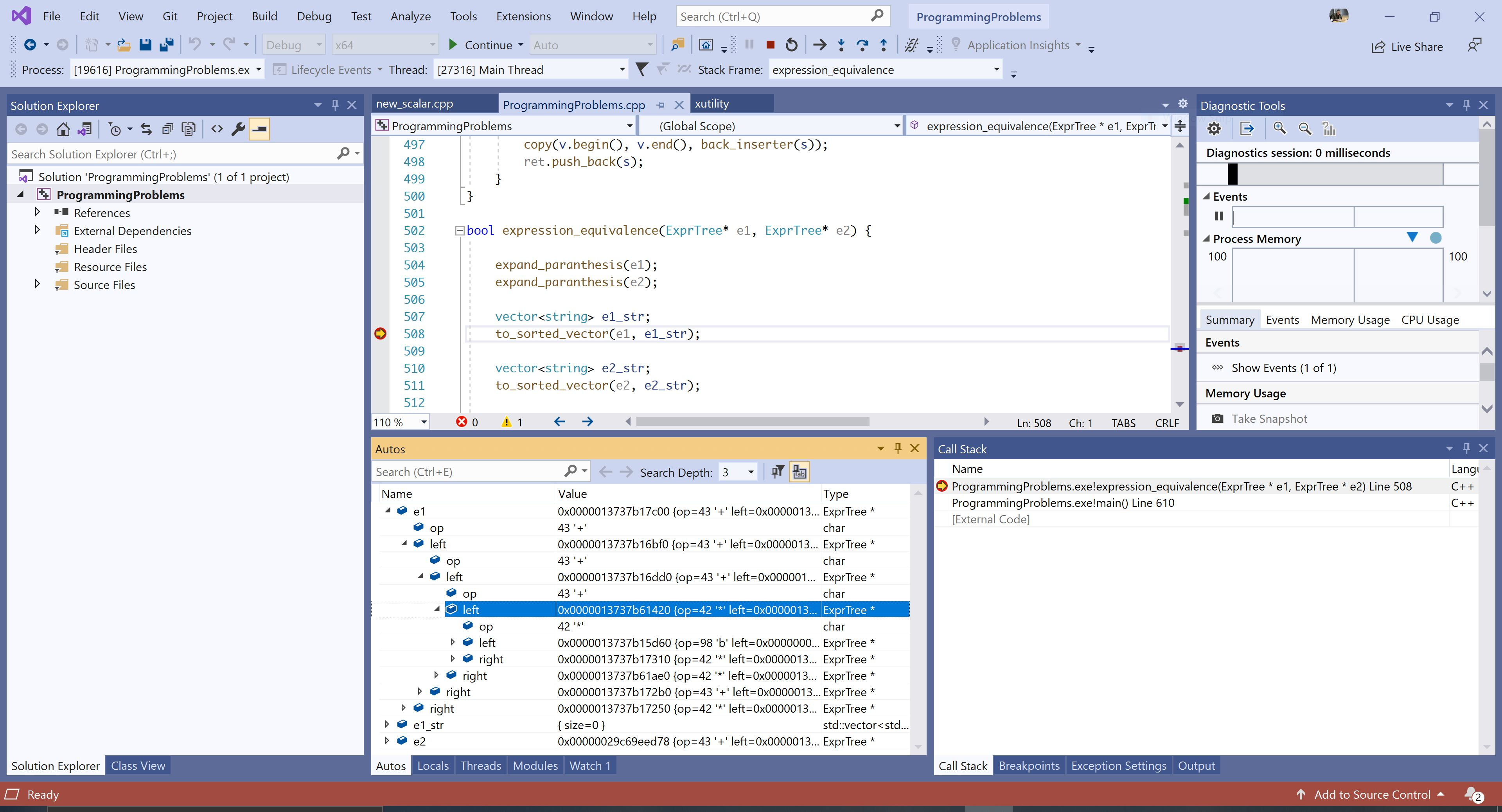]]>
WebGL Fun2020-04-15T09:15:16+02:002020-04-15T09:15:16+02:00https://alexandrugris.github.io/graphics/3d/2020/04/15/intro-webglA post about computer graphics, for the web mostly, with JavaScript, WebGL, ThreeJS and shaders. A little bit of maths also.

### ThreeJS Introduction

ThreeJS is a minimalistic 3D game engine for the web, with a very simple to use and very nicely designed API. It comes in the form of a javascript library, accompanied by a set of util libraries, a scene editor running on the web and lots and lots of examples an tutorials.

By the end of this blog post we will build this: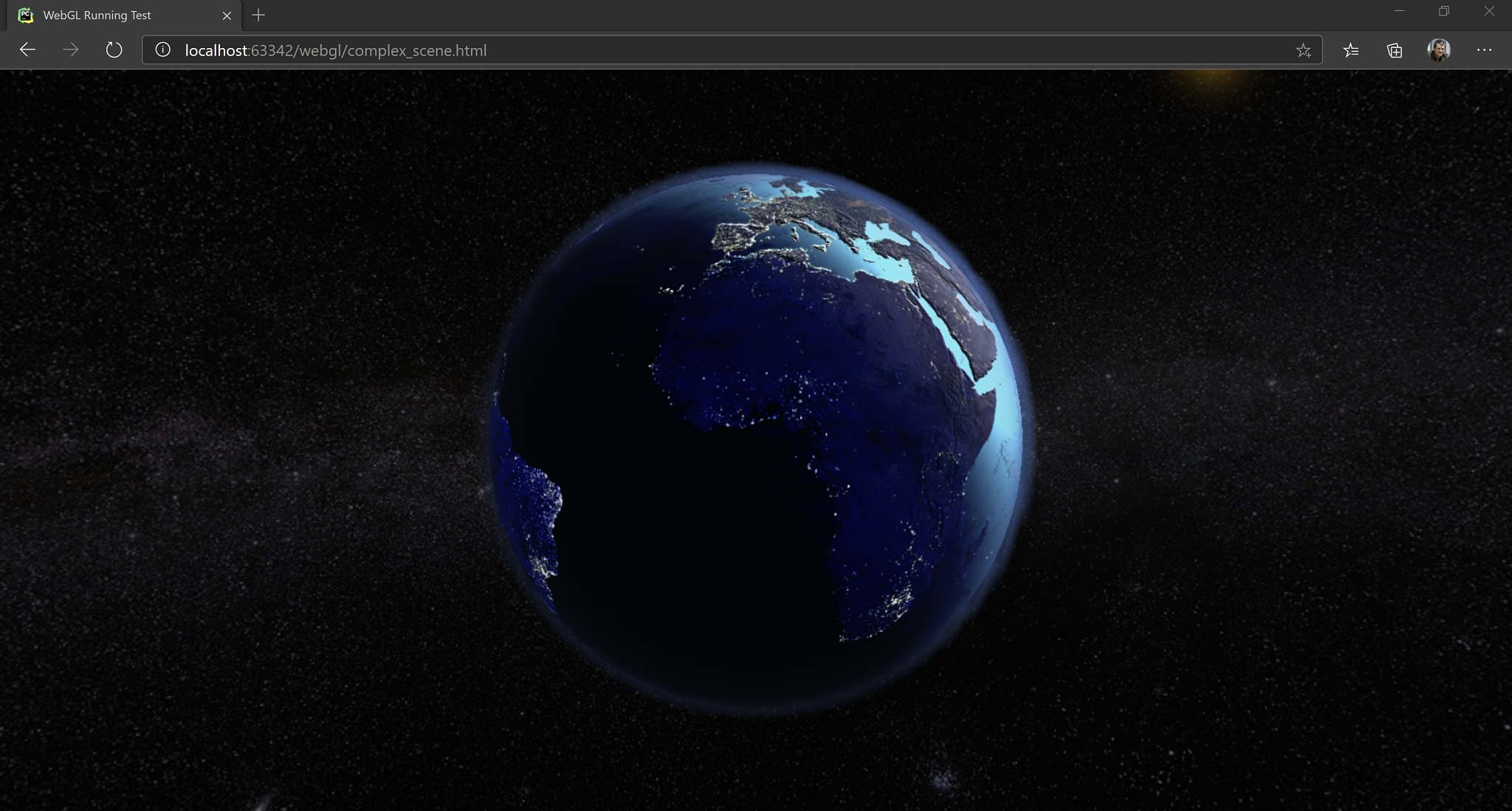By default, ThreeJS already has built in materials for most of the effects one might want to add to a scene. I addition to what is already built in, there are lots of samples and pre-made effects in the form of libraries on github. Therefore, for most work, it be used entirely from JavaScript. While the API is clean and short and performs as expected, a little bit of maths and graphics background will still be needed sooner or later in the project.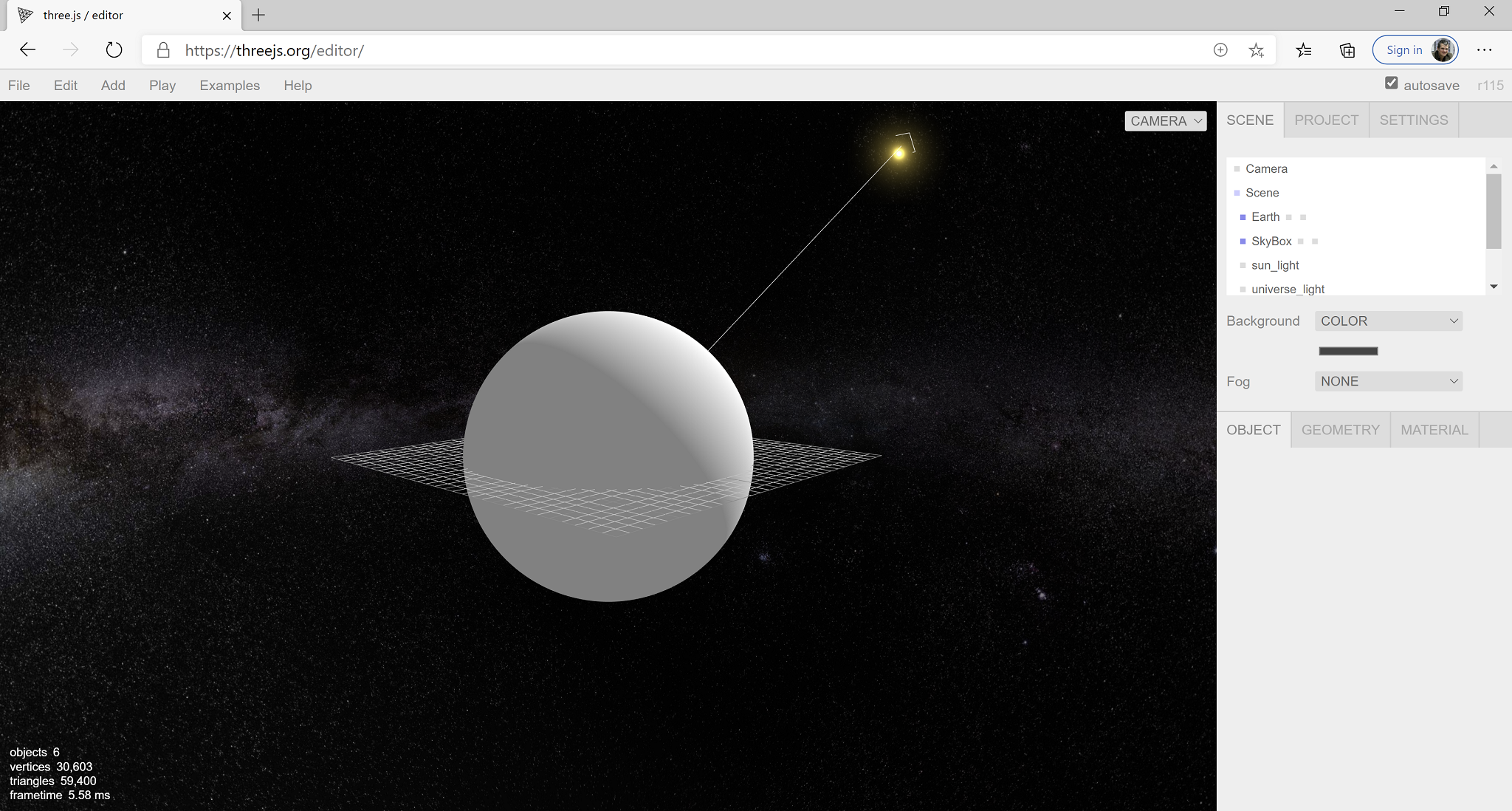### Initialization and The First Scene

The simplest way to run ThreeJS is to cover the full browser window. It goes like this:

``````<html>
<!-- Run everything as a module -->
<script type="module">

import {main, resize} from "./js/complex_scene.js";

window.onresize = resize;

</script>

<body>
<!-- Full screen, positioned top-left corner -->
<div id="webgl-container" style="position: absolute; top: 0; left:0 ; margin: 0"></div>

</body>

</html>
``````

Then, in the JavaScript file:

``````// downloaded beforehand in the libs folder
import * as THREE from "./libs/three.module.js"

// create new renderer
const renderer = new THREE.WebGLRenderer();

// util for variable frame rate
const clock = new THREE.Clock(true);

``````

We are also going to make use of the following two functions, as vectors are kept by reference in the ThreeJS code and, in many cases, we need copies to do transforms only on resulting vector, not on the source.

``````function newVector(v){
return new THREE.Vector3(v.x, v.y, v.z);
}

function copyVector(dest, src){
dest.x = src.x;
dest.y = src.y;
dest.z = src.z;
}
``````

Now we can proceed further to initialization

``````
function resize() {
renderer.setSize(window.innerWidth, window.innerHeight);

if(camera != null) {
camera.aspect = window.innerWidth / window.innerHeight;
camera.updateProjectionMatrix(); // DON'T FORGET!
}
}

async function main() {

// initialize the renderer
renderer.setSize(window.innerWidth, window.innerHeight);
document.getElementById("webgl-container").appendChild(renderer.domElement);

// for the first demo will we create scene programatically,
// for the second we load the scene as exported from the ThreeJS editor

// if objects are loaded from the network, initScene should be async / awaited
initScene();
renderScene();

}

export { main, resize }
``````

We are also going to use the following global variables:

``````// the scene object
const scene = new THREE.Scene();

// a light, must be added to the scene if we want to see something
const light = new THREE.AmbientLight(0xffffff);

// a camera
let camera = null;
``````

Initialize them, add them to the scene:

``````
function initScene(){

// 1. create the renderer and add its element to the scene
renderer.setSize(window.innerWidth, window.innerHeight);
document.getElementById("webgl-container").appendChild(renderer.domElement);

// 2. create the camera, position it in the world and add it to the scene
camera = new THREE.PerspectiveCamera(35, window.innerWidth/window.innerHeight, 1, 1000);
camera.position.z = 100;

// 3. add the light to the scene

// 4. Create an object. An object an item of the class THREE.Mesh()
// It has two constituents: a geometry and a material

// The geometry is created from the library of predefined geometries.
// Check the docs for other prefedined geometries

// The material is a single color (red) material, rendered in wireframe
// wireframe property is set in the constructor.
// ThreeJS predefines many materials for everyday use.
let box = new THREE.Mesh(
new THREE.BoxGeometry(20, 20, 20),
new THREE.MeshBasicMaterial(
{
color: 0xff0000,
wireframe: true
})
);

// 5. Give a name to my 3D object so we can find it in the scene later
box.name = "my-box";

// 6. A super useful helper for debugging, the AxesHelper, shows the orientation of my 3D object.
// This is added as a child to the box so it moves through the scene together with its parent.

// 7. Add the box to the scene

// the next two objects will be detailed later
// (a) how to create geometry programatically
// (b) how to modify geometry programatically

}
``````

Beside the `THREE.MeshBasicMaterial` shown above, which is a flat renderer, not affected by lights, ThreeJS comes with a powerful material library. Some of the classes discussed below:

• `LineMaterial` - allows drawing lines
• `LineDashMaterial` - allows drawing dashed lines
• `MeshLambertMaterial` - basic per-vertex lighting, no specular
• `MeshPhongMaterial` - per-pixel lighting, specular. Offers interesting properties for setting the diffuse texture, environment map, emissive, displacement map, bump map, light map, normal map, both object space and tangent space, etc.
• `MeshToonMaterial` - toon shading
• `MeshStandardMaterial` - physically-based rendering material
• `SpriteMaterial` - rendering sprites
• `DepthMaterial` - for rendering the depth buffer
• `ShaderMaterial` - for custom shaders written in GLSL. We will use this material later on.

The wide slection of materials available means that a lot can be done with just JavaScript, without touching any advanced rendering techniques or limiting custom rendering code to very special sections of your scene.

Redering the scene is super easy as well:

``````function render(){

// 1. optinally call an update method
update(clock.getDelta())

// 2. render the scene
renderer.render(scene, camera);
requestAnimationFrame(render);
}
``````

### Fun With Vertices

We mentioned two more objects in the scene initialization code above:

• Generating geometry
• Updating geometry

Here it is how it goes:

``````function createTriangleGeometry(size, singleColor = false){

// 1. Create a geometry object and push some vertices to it.
// In this case we create a triangle
let geom = new THREE.Geometry();
geom.vertices.push(new THREE.Vector3(-size * 0.5, 0, 0));
geom.vertices.push(new THREE.Vector3(size * 0.5, 0, 0));
geom.vertices.push(new THREE.Vector3(0, Math.sqrt((3.0 / 4.0) * size * size )), 0);

// 2. Set the indexes for each triangle constituting the geometry
// In our case, we have a single face since we draw a single triangle
// ThreeJS uses indexed geometries.
geom.faces.push(new THREE.Face3(0, 1, 2));

let mat = null;

// 3. Set the material properties
// in the `else` case we setup vertex colors which will be sent to the shaders as
// vertex color parameters.
if (singleColor) {
mat = new THREE.MeshBasicMaterial({color: 0x00ff00});
}
else{
mat = new THREE.MeshBasicMaterial({
side: THREE.DoubleSide,
vertexColors: THREE.VertexColors
})

geom.faces.vertexColors = new THREE.Color(0xff0000);
geom.faces.vertexColors = new THREE.Color(0x00ff00);
geom.faces.vertexColors = new THREE.Color(0x0000ff);

}

// 4. Return the mesh that can be added to the Scene
return new THREE.Mesh(geom, mat);

// 5. Check out ExtrudeGeometry and ShapeGeometry and GeometryUtils
// for different means and utilities for generating geometry in code
}
``````

And updating geometry on the fly:

``````class AnimatedPlaneGeometry extends THREE.Mesh{

constructor() {
// 1. initialize this geomerty as a plane
super(new THREE.PlaneGeometry(40, 40, 40, 40),
new THREE.MeshBasicMaterial({wireframe: true})) ;

// 2. give it a name so it can be accessed from the scene
this.name = "my-wave";
}

update(dt){

// 3. update the geometry, this is a sinosoidal wave
for(let i = 0; i < this.geometry.vertices.length; i++){
this.geometry.vertices[i].z = Math.sin(this.geometry.vertices[i].x + 0.05 * dt)
}

// 4.: must call the following to update the geometry.
// otherwise the buffers will not be updated
this.geometry.verticesNeedUpdate = true;
}
}
``````

If all went well, with an update function like the following,

``````function update(dt){
let box = scene.getObjectByName("my-box");
box.rotation.y += 0.1 * dt;

let wave = scene.getObjectByName("my-wave");
wave.update(dt);
}
``````

Something like the following scene should appear in the browser. The full code is in my github account, in the WebGL project.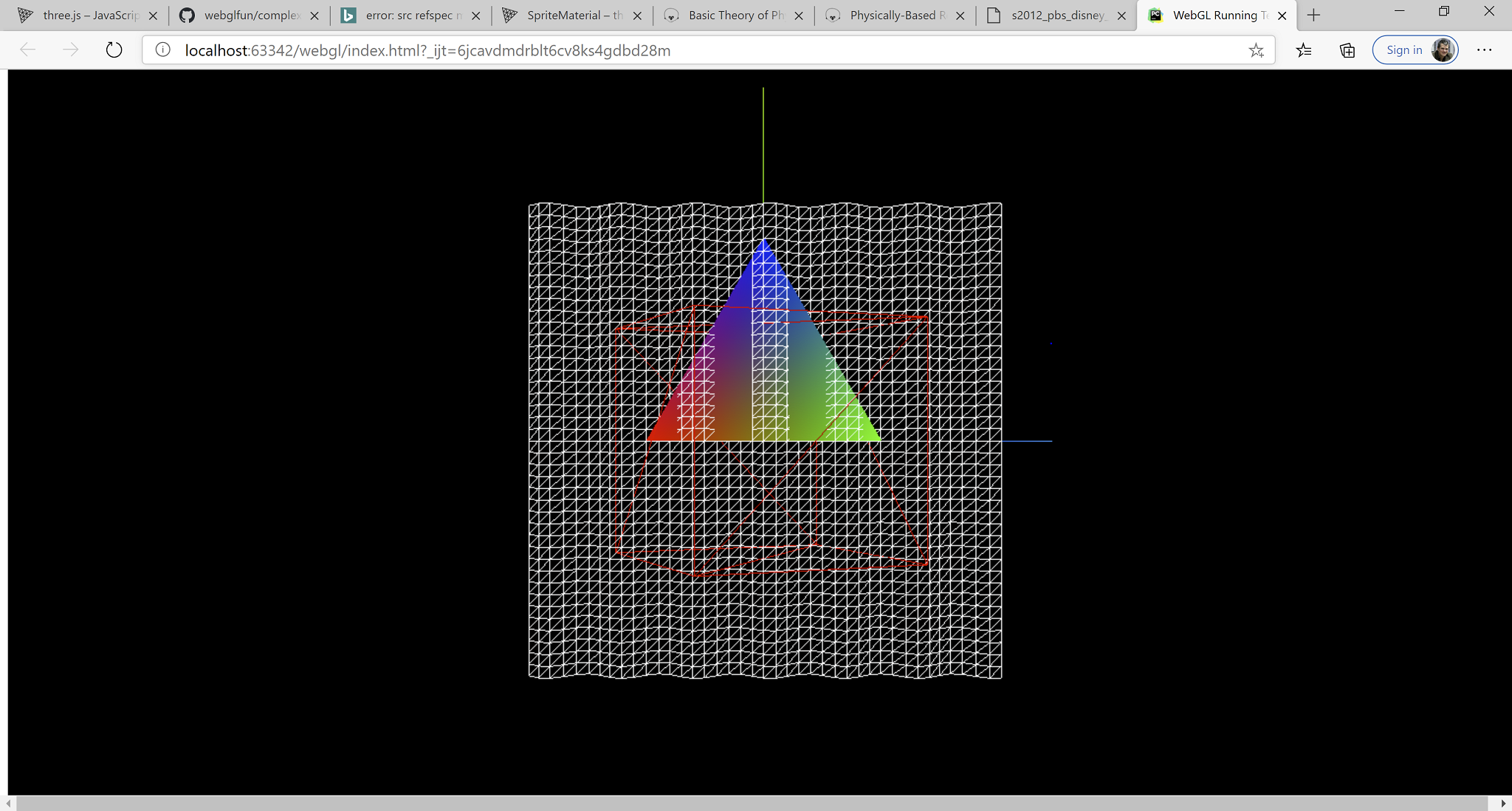The scene contains:

• The red wireframe cube rotating (`my-box`) with the `AxesHelper` added and rotating with its parent.
• The waving plane, updated geometry (`my-wave`).
• The colorful triangle created on the fly.

### Shaders and Rendering Of The Earth

Why the Earth? Because textures can be found free online, because rendering it requires some specific techniques, like skyboxes, normal mapping, lighting, atmosphere rendering and because the result is guaranteed to be beautiful.

A very good rendering of the Earth can be obtained by using materials already provided by the engine or by the community. However, since this was a pet project, we are doing many things from scratch. Also, being a pet project written among other things, the code is not production ready. I have not tested it on other computer except for my laptop which is quite powerful. Also, I have not optimized the code. It’s just the first thing that worked.

Unlike the previous example where we built the scene manually, here I created it in the ThreeJS editor and then exported it. The loading code goes like this:

``````async function main() {

//1. initialize the renderer
renderer.setSize(window.innerWidth, window.innerHeight);
document.getElementById("webgl-container").appendChild(renderer.domElement);

//2. load the scene from editor exported objects

//3. fix the camera, the camera has also been loaded from JSON, but its parameters
// neeed to be adjusted to our viewport
camera.aspect = window.innerWidth/window.innerHeight;
camera.updateProjectionMatrix();
camera.updateMatrixWorld();

//4. load the FlyControls library (premade) so we can move through the scene
cameraControls = new FlyControls(camera, renderer.domElement);
cameraControls.dragToLook = true;
cameraControls.movementSpeed = 4.0; // scene-units per second
cameraControls.rollSpeed = 0.1; // radians per second

//5. the skybox requires separate treatment, will be coved later in the post
// her we remove it from the scene
skybox = scene.getObjectByName('SkyBox');
scene.remove(skybox);

//6. we are also fixing the atmosphere and make it a child of the earth so the move together
earth = scene.getObjectByName("Earth");
atmosphere = scene.getObjectByName("Atmosphere");
scene.remove(atmosphere);

fixMaterials().then( () => {

// 8. since we have a skybox rendered as a separate step
// we don't want to renderer to erase the scene for us between rendering
// also part of the rendering of the skybox
renderer.autoClear = false;
scene.background = null;

// 9. start the renderign loop
render()
});
}
``````

For loading the scene and the textures we are going to use two functions:

``````async function loadObject(json){
}

// here we are intercepting the texture loader
// we want the textures to be as beautifully rendered as possible at the cost of performance
// therefore, we use the highest anisotropy level the renderer provides
// for my device, it is 16
// this makes the textures look sharp when seen from the side
// https://en.wikipedia.org/wiki/Texture_filtering#Anisotropic_filtering
tex.anisotropy = renderer.capabilities.getMaxAnisotropy();
resolve(tex);
}, null, reject))
}
``````

Setting up a shader is performed in the `fixMaterials` function. Its basic structure as as follows:

1. Define the set of uniforms and bind them to JS variables. Uniforms are the variables that are set in code and submitted on each rendering pass to the shading programs.
2. Create a `ShaderMaterial`
3. Set the uniforms and then load the vertex shader and the pixel shaders. In our case, we store these in our DOM tree, in the html file.

Let’s perform these steps to render the sky dome. In our case it’s a sphere, not box.

### SkyDome And Light

1. Setting up the uniforms:
``````async function fixMaterials() {

// first is the SkyBox
skyBoxUniforms = {
diffuseTexture: {
type: "t",
},
}

[... more to come here ...]
``````
1. Create the `ShaderMaterial`:
``````  skybox.material = new THREE.ShaderMaterial({

// a) set the uniforms
uniforms: skyBoxUniforms,

// b) load the vertex and pixel shader from the HTML DOM

// c) set other parameters
// In our case, always show the skybox behind all other objects
depthTest : false,
depthWrite: false,

// d) we are always inside the box
side: THREE.BackSide,

});
``````

Rendering the skybox is a bit trick as the following are done:

• The skybox is always as at the same distance from the camera. We don’t get closer to it, we don’t get further from it. It moves with the camera.
• The skybox is behind any object in the scene, it cannot intersect any object. Thus we don’t update the the Z-buffer and we don’t read from it. We render the skybox as a separate step and we don’t erase the background between rendering the skybox and rendering the rest of the scene.

Here’s how the rendering loop looks like:

``````function render(){

// 1. update the scene, geometries, etc
update(clock.getDelta())

// 2. clear the background
renderer.clear();

// 3. render the skybox
if(skybox != null){
renderer.render(skybox, camera);
}

// 4. without clearing the background, render the rest of the scene
renderer.render(scene, camera);
requestAnimationFrame(render);
}
``````

The shaders for the skybox are super straight forward, as we don’t apply any lighting to it.

``````<script type="x-shader/x-vertex" id="skyBoxVertexShader">

uniform vec3          lightDirection;

varying vec2          vUv; // pass the uv coordinates of each vertex to the frag shader
varying float         lightIntensity;

void main()
{
vUv = uv;
gl_Position = projectionMatrix * modelViewMatrix * vec4(position, 1.0);

// artistic effect: dim when looking at the light source
// no special reason for the effect, just feel nicer
// it should be computed outside the shader
lightIntensity = clamp(i * i, 0.4, 1.0);
}
</script>

uniform sampler2D diffuseTexture;
varying vec2 vUv;
varying float lightIntensity;

void main()
{
gl_FragColor = texture2D(diffuseTexture, vUv) * lightIntensity;
}
</script>
``````

Now, the trickery has not yet finished. We have a sun that needs to stick to the skybox when the skybox rotates and moves through the scene, remember it is bound to the camera, and we want to make sure the light direction is preserved and it accurately comes from the sun. So we do these updates in the update method.

``````
function update(dt){

// 1. Allow the camera to move
if(cameraControls){
cameraControls.update(dt);
}

// 2. ensure the sun and the light have the same direction and they stick to the skybox
let sunLight = scene.getObjectByName('sun_light');
let sunSprite = scene.getObjectByName('sun_sprite');

let lightPos = sunLight.position.normalize();
let lightPosU = new THREE.Uniform(newVector(lightPos));

if(skybox) {

copyVector(skybox.position, camera.position);
skybox.rotation.x += 0.005 * dt;
skybox.rotation.y += -0.1 * dt;

skyBoxUniforms.lightDirection = lightPosU;

skybox.updateMatrixWorld();

// keep the sun in the same place in the sky
if (sunSprite.originalPositionSkyboxSpace === undefined){
// the sun sprite
let invWorld = new THREE.Matrix4();

sunSprite.originalPositionSkyboxSpace = newVector(sunSprite.position);
sunSprite.originalPositionSkyboxSpace.applyMatrix4(invWorld.getInverse(skybox.matrixWorld));
sunSprite.originalSkyboxPosition = newVector(skybox.position);
}

// make sure the light comes from the sun and not some random point
let newPos = newVector(sunSprite.originalPositionSkyboxSpace);
newPos.applyMatrix4(skybox.matrixWorld);
copyVector(sunSprite.position, newPos);
sunSprite.updateMatrixWorld();

lightPos = new THREE.Vector3();
let skyboxMovement = new THREE.Vector3();
skyboxMovement.subVectors(skybox.position, sunSprite.originalSkyboxPosition);
lightPos.subVectors(sunSprite.position, skyboxMovement);
copyVector(sunLight.position, lightPos);
}
``````

### Rendering the Earth

Now, rendering of the Earth and its atmosphere can vastly be improved, especially perfomance-wise. But I am running out of vacation time and I really want to finish this project today so I stop at the current implementation. Some quick wins:

• Make lighting done in tangent space. It will reduce some matrix multiplications in the pixel shader.
• Make the atmosphere rendering per-pixel. Now the atmosphere thickness is computed per vertex and this gives some ugly artefacts.
• Tune the clouds and their shadows. While the shadow moves correctly with the camera, when they are brightly lit or when they are not fully lit there are some visual artefacts.

``````// 1. setup the uniforms, load the textures
earthUniforms = {

diffuseTexture: {
type: "t",
},

diffuseNight: {
type: "t",
},

normalMap: {
type: "t",
},

specularMap: {
type: "t",
},

cloudsMap: {
type: "t",
}

}

// 2. Cheat a bit and use a library function to compute the tangets
// We will be using tangent-space normal mapping. The function was too easy to grab
// not to use it.

BufferGeometryUtils.computeTangents(earth.geometry);

uniforms: earthUniforms,

side: THREE.FrontSide

});
``````

In the update function, we also update the position and we bind the updated light position to the shader:

``````function update(dt){

[....]

if(earth){
earthUniforms.lightDirection = lightPosU;
earth.rotation.x -= 0.001 * dt; // some rotation
earth.rotation.y += 0.05 * dt;
}
}
``````

``````<script type="x-shader/x-vertex" id="earthVertexShader">

uniform vec3 lightDirection;

// all in eye space
varying vec2 vUv;
varying vec3 vEyeDirectionEyeSpace;
varying vec3 vLightDirection;
varying mat3 tbn;

// the tangent, sent per-vertex
attribute vec4 tangent;

void main(){

// 1. copy the texture coordinates
vUv = uv;

// 2. update the position
gl_Position = projectionMatrix * modelViewMatrix * vec4(position, 1.0);

// 3. compute the light direction from world to eye;
// should be computed outside of shader for performance
vLightDirection = mat3(viewMatrix) * lightDirection;

// 4. compute the direction to the eye
vEyeDirectionEyeSpace = mat3(viewMatrix) * normalize(position - cameraPosition).xyz;

// 5. prepare the tangent-bitangent-normal matrix for normal mapping
vec3 t = normalize(tangent.xyz);
vec3 n = normalize(normal.xyz);
vec3 b = normalize(cross(t, n));

// everything in eye space
t = normalize(normalMatrix * t);
b = normalize(normalMatrix * b);
n = normalize(normalMatrix * n);

tbn = mat3(t, b, n);
}

</script>

// all my textures
uniform sampler2D diffuseTexture;
uniform sampler2D diffuseNight;
uniform sampler2D specularMap;
uniform sampler2D cloudsMap;
uniform sampler2D normalMap;

// inputs, interpolated per vertex
varying vec2 vUv;
varying vec3 vEyeDirectionEyeSpace;
varying vec3 vLightDirection;
varying mat3 tbn;

void main(){

vec3 lightDir = normalize(vLightDirection);

// 1. compute the normal based on the texture and bring it to eye space
vec3 n = texture2D(normalMap, vUv).xyz * 2.0 - 1.0;
vec3 normal = normalize(tbn * n);

// 2. directional light
float lightIntensity = dot(normal, lightDir);

// 3. use the surface normal, stored in tbn, as a selector for the day-night texture
// we don't do lighting per se, we use a blend of day/night textures for it
float selectImage = dot(tbn, lightDir);
gl_FragColor = texture2D(diffuseTexture, vUv) * selectImage + texture2D(diffuseNight, vUv) * (1.0-selectImage);

// 4. we light the pixels a bit, true, but we only use the remainer from the intensity-select,
// so we don't overlight
gl_FragColor *= (1.0 + 10.0*(lightIntensity - selectImage));

// 5.  specular
vec3 reflection = reflect(lightDir, normal);
float specPower = texture2D(specularMap, vUv).r;

float spec = 4.0;
float gloss = 2.0 * texture2D(specularMap, vUv).a;

float specular =  pow(clamp(dot(reflection, normalize(vEyeDirectionEyeSpace)), 0.0, 1.0), spec) * gloss;
gl_FragColor = gl_FragColor + specular * vec4(0.26, 0.96, 0.99, 1);

// 6. cloud colors
vec4 cloudsColor = texture2D(cloudsMap, vUv) * vec4(1.0, 0.5, 0.2, 1.0);

// 7. fake cloud shadow based on how we are looking at the cloud, to give some impression of depth
vec4 cloudsShadow = texture2D(cloudsMap, vec2(vUv.x + normal.x * 0.005, vUv.y + normal.y * 0.005));

if (cloudsColor.r < 0.1 && cloudsShadow.r > 0.1){
gl_FragColor *= 0.75;
}

gl_FragColor = gl_FragColor * (vec4(1.0) - cloudsColor) + cloudsColor * (lightIntensity * 2.0);

}

</script>
``````

And last, but not least, the atmosphere. This is the most beautiful part of the model imho.

The first thing to note is that the atmosphere is using alpha blending. Nothing fancy, but without the earth beneath it won’t be visible. The atmosphere itself is a sphere with no texture, rendered on top of the earth and rotating together with it. Here is the shader config:

``````
atmosphereUniforms = {

earthCenter: new THREE.Uniform(earth.position),

}

uniforms: atmosphereUniforms,

blending: THREE.CustomBlending,
blendSrc: THREE.SrcAlphaFactor,
blendDst: THREE.OneMinusSrcAlphaFactor,
side: THREE.FrontSide,

transparent: true,
});
``````

`````` <script type="x-shader/x-vertex" id="atmosphereVertexShader">

uniform vec3 earthCenter;
uniform vec3 lightDirection;

varying float atmosphereThickness;
varying vec3 vLightDirection;
varying vec3 vNormalEyeSpace;

void main(){

// 1. compute the position
gl_Position = projectionMatrix * modelViewMatrix * vec4(position, 1.0);

// 2. compute the thinckness of the atmosphere
// for this, we intersect the vector (eye - current vertex) with the atmosphere and the earth
// and we compute how long this line is. In pixel shader we compute the light scattering based on this measure
// https://www.scratchapixel.com/lessons/3d-basic-rendering/minimal-ray-tracer-rendering-simple-shapes/ray-sphere-intersection

vec3 positionW = (modelMatrix * vec4(position, 1.0)).xyz;

vec3 vCameraEarth = cameraPosition.xyz - earthCenter;
vec3 vCameraVertex = normalize(cameraPosition.xyz - positionW);

float tca = dot(vCameraEarth,  vCameraVertex);

if (tca < 0.0){
// not intesect, looking in opposite direction
atmosphereThickness = 0.0;
return;
}

float dsq = dot(vCameraEarth, vCameraEarth) - tca * tca;

float thc_atmosphere = 2.0 * sqrt(thc_sq_atmosphere);
float thc_earth = 2.0 * sqrt(max(0.0,thc_sq_earth));

float thc = (thc_atmosphere - thc_earth) * 0.12; // 0.01 - density factor
atmosphereThickness = thc;

// 3. the normal light calculation
vLightDirection = mat3(viewMatrix) * lightDirection;
vNormalEyeSpace = normalize(normalMatrix * normal);

}

</script>

varying float atmosphereThickness;
varying vec3 vLightDirection;
varying vec3 vNormalEyeSpace;

void main(){

vec3 lightDir = normalize(vLightDirection);
vec3 normal = normalize(vNormalEyeSpace);

// computing the light intensity as it is scattered through the atmosphere
// based on actual lighting extended a bit
// and the thickess
float lightIntensity = max(dot(normal, lightDir) * 1.5, -0.7);
gl_FragColor = vec4( (vec3(57.0, 97.0, 162.0) / 256.0) * (1.0 + lightIntensity), atmosphereThickness);
}
</script>
``````

And that was my first play with WebGL and ThreeJS. I will soon publish the demo somewhere but might not work on my video cards.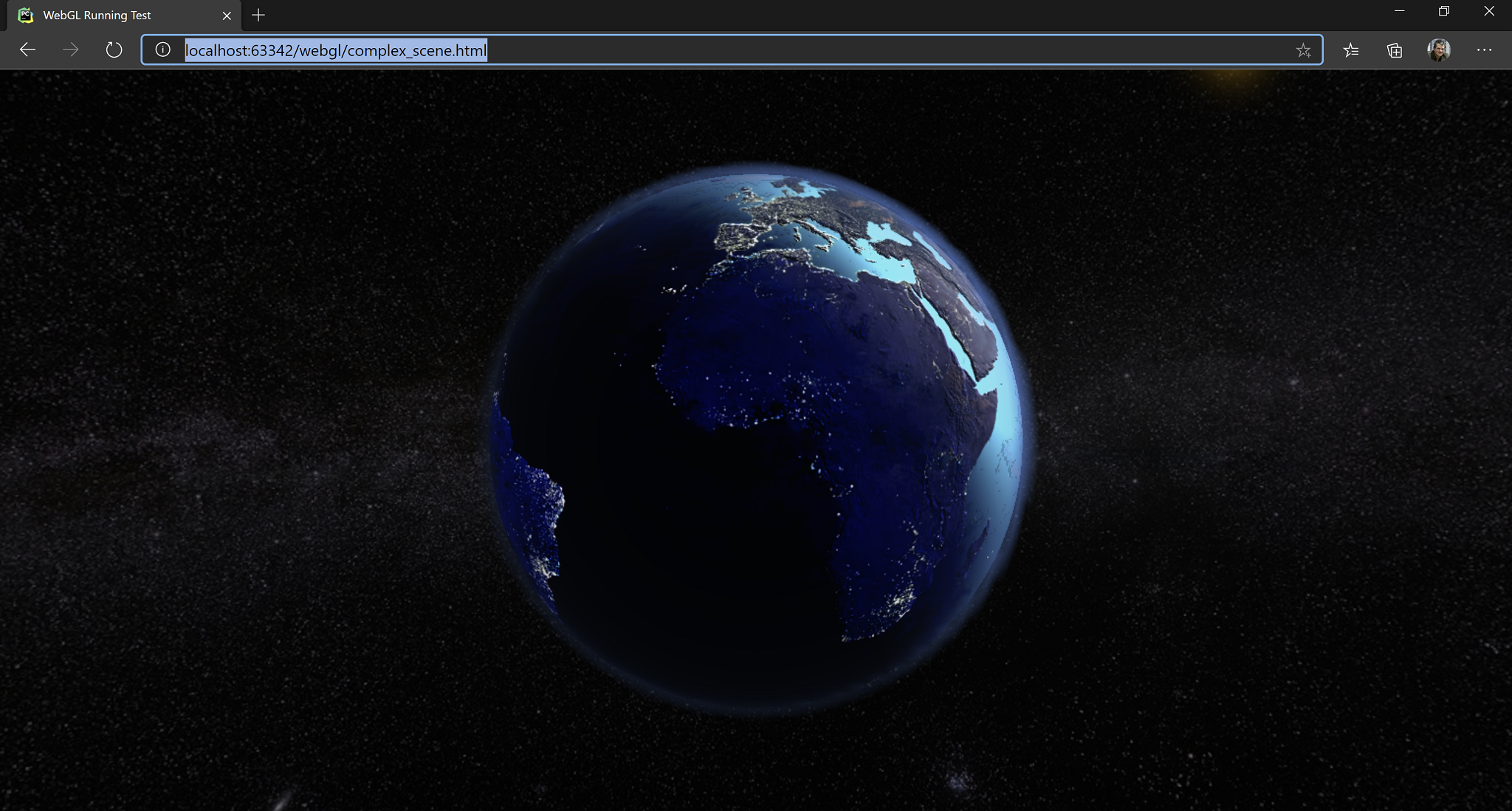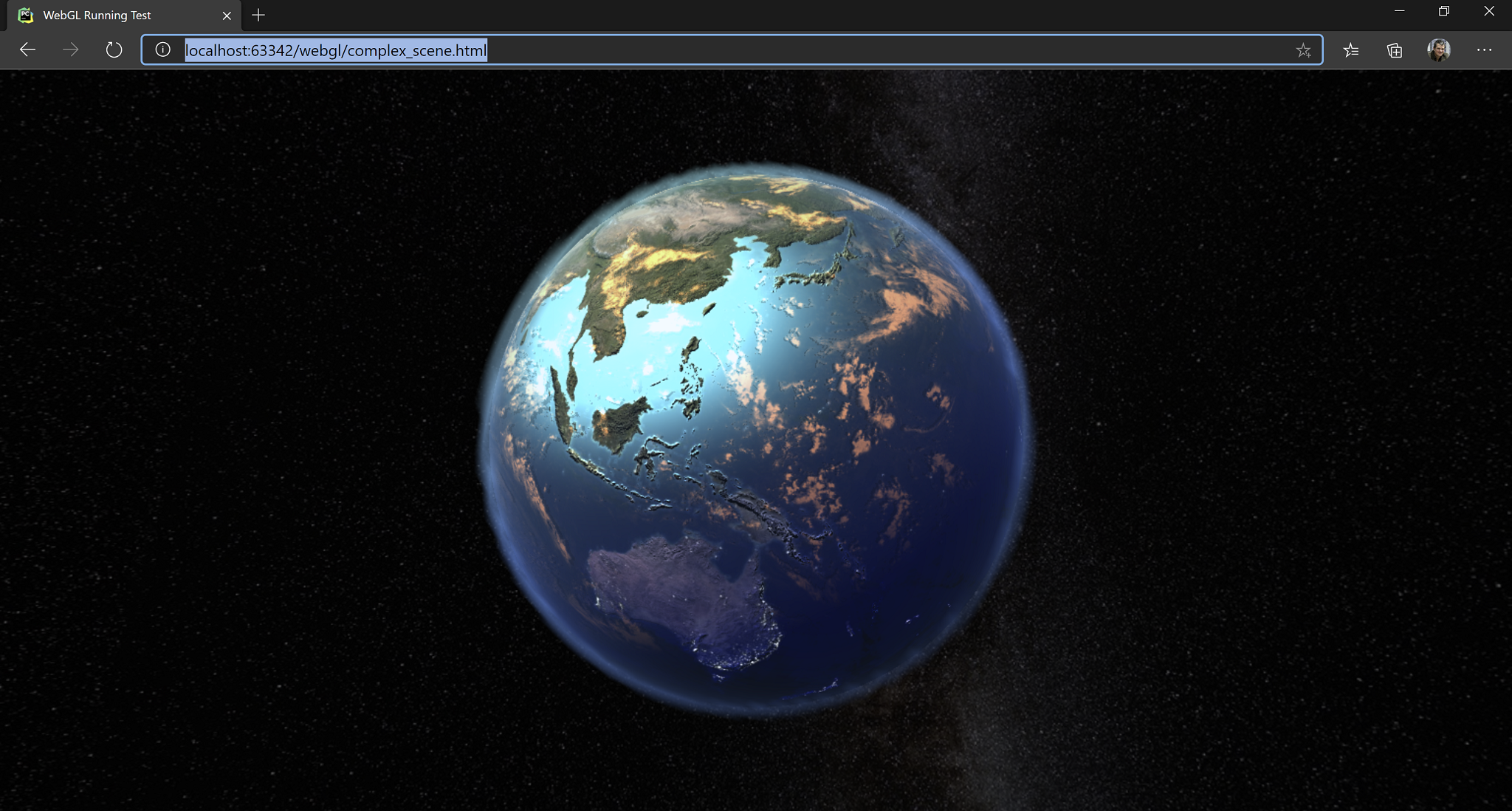]]>
3D From Scratch2020-04-15T09:15:16+02:002020-04-15T09:15:16+02:00https://alexandrugris.github.io/graphics/3d/2020/04/15/3d-from-scratchThis post is about implementing a 3D renderer from scratch, with no help from any graphics or maths library. It is implemented in pure JavaScript and it follows roughly the first half of the excellent tiny renderer tutorial.

### The End Result

We are going to build our software renderer to show this: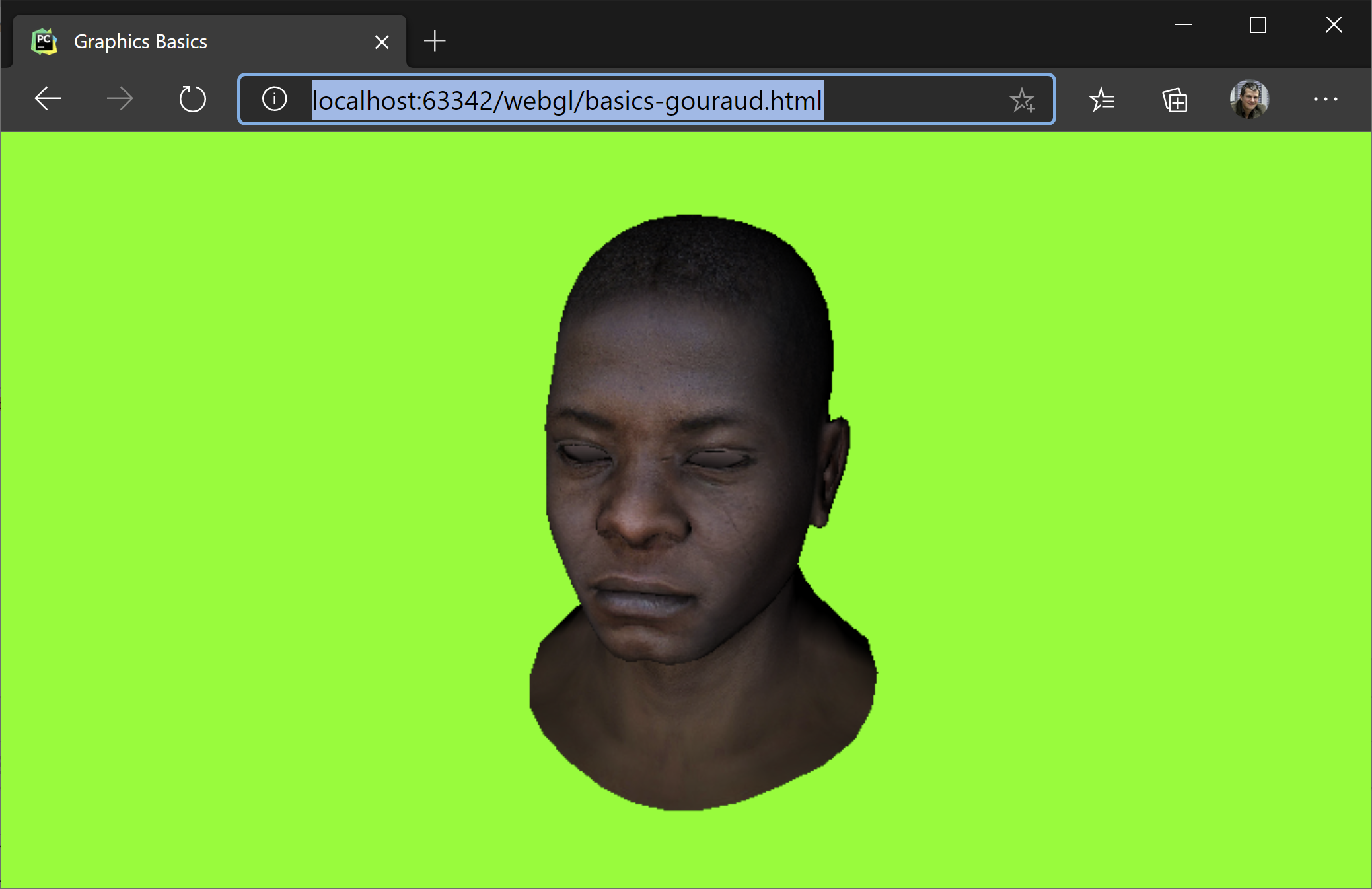The features our software renderer suppors are:

• Phong (per pixel) lighting
• Triangle rasterization
• Model, camera, viewport transformations
• Wireframe rendering
• Texturing
• Z-Buffer
• Hidden face removal (backface culling)

In addition to these, we will build a small and probably buggy maths library. All the code is included in this file: basics-phong

### Coordinate Systems

First and foremost, we will operate in the coordiate system with the z-axis pointing towards us, y-axis upwards and the x-axis rightwards.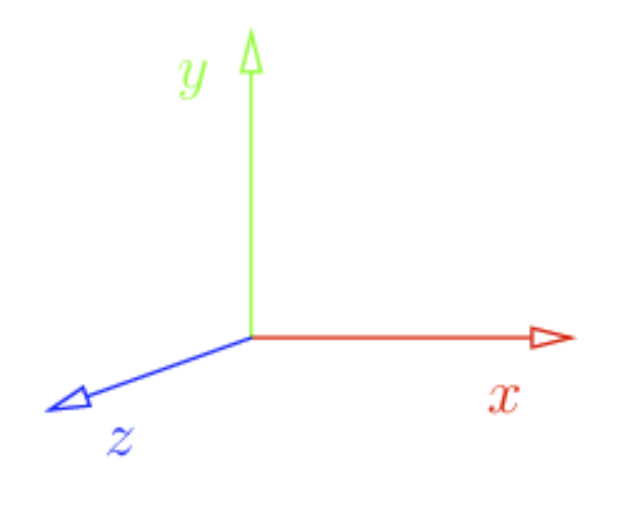In addition to that, the model we will load is oriented towards z-axis. Also, by convention, we will consider triangles defined as counter-clockwise. This will help us later determine what is the front and what is the the back face of the triangle.

The format for our model is standard: an indexed geometry, with a set of vertices, vertex normals and texture coordinates. Taking into consideration the counter-clockwise convention, here is how we define a quad.

``````function generateTexturedQuad(mesh){
mesh.vertices.push([-1, 1, 0])
mesh.vertices.push([-1, -1, 0])
mesh.vertices.push([1, 1, 0])
mesh.vertices.push([1, -1, 0])

mesh.faces.push([0, 0, 0, 1, 1, 1, 2, 2, 2], [2, 2, 2, 1, 1, 1, 3, 3, 3]);

mesh.txcoords.push([0, 1, 0]);
mesh.txcoords.push([0, 0, 0]);
mesh.txcoords.push([1, 1, 0]);
mesh.txcoords.push([1, 0, 0]);

// all normals pointing towards the camera
// in the case when 3d artists are not so kind,
// you can recompute the normal vectors as an average of normals to all
// facets incident to the vertex

mesh.vnormals.push([0, 0, 1]);
mesh.vnormals.push([0, 0, 1]);
mesh.vnormals.push([0, 0, 1]);
mesh.vnormals.push([0, 0, 1]);

if(mesh.worldTransform === undefined || mesh.worldTransform == null)
mesh.worldTransform = getIdentityMatrix(4);

}

myMesh = {
vertices: [],
txcoords: [],
vnormals: [],
faces: [],
diffuse: diffuse,
worldTransform: null,
};

}
``````

Rendering this image also assumes the following are set:

``````viewportTransform = makeViewportTransform(cvs.width, cvs.height);
projectionTransform = getIdentityMatrix(4); // no projection
cameraTransform = makeIdentityCamMatrix(); // no camera transformation
``````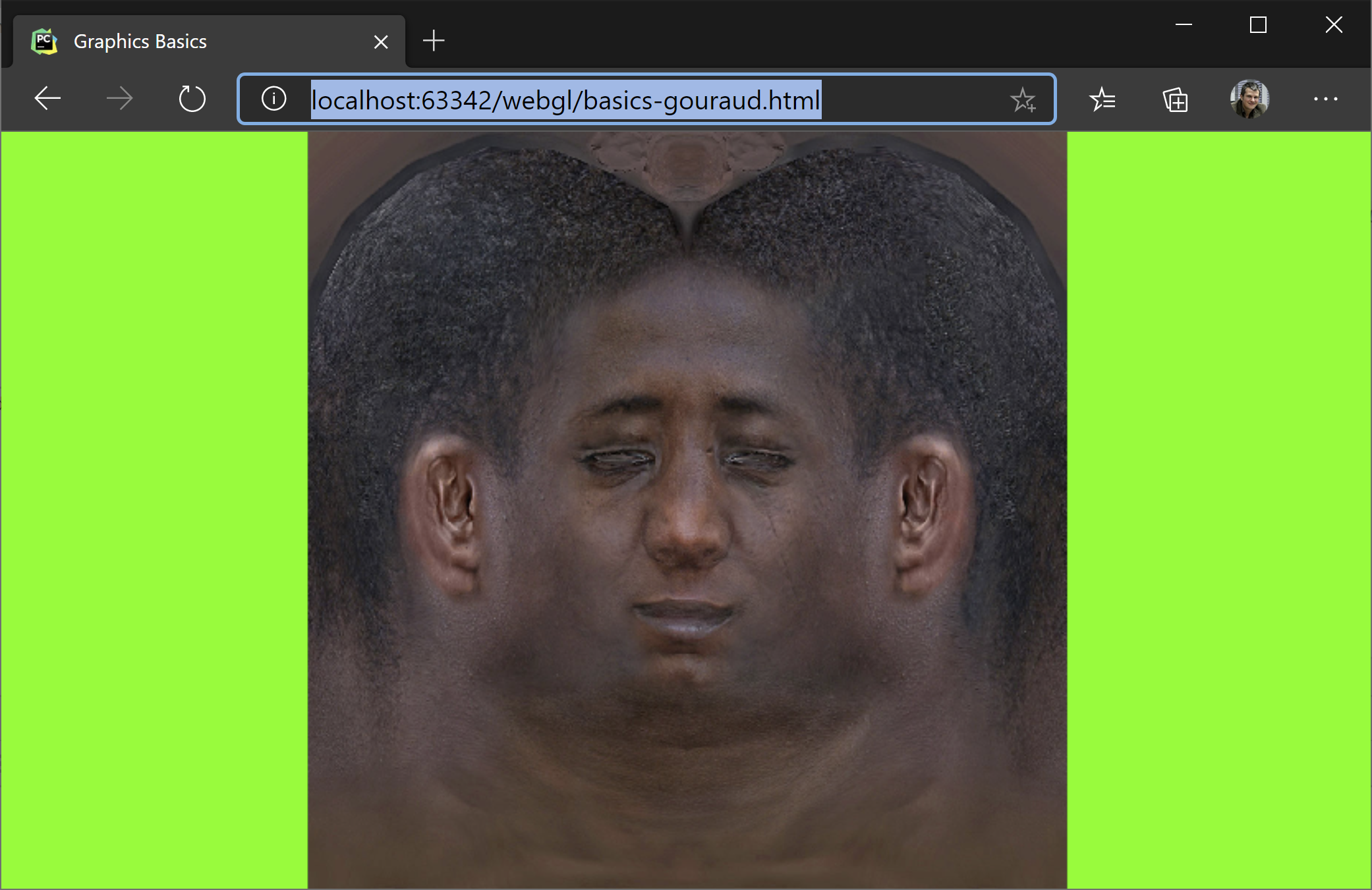Since `projectionTransform` and `cameraTransform` are identity matrices, it means that the only transformation in place is the `viewportTransform`. This transform takes a coordiante space defined by the rectangle `x, y = [-1, 1], [-1, 1]`, with `y` pointing upwards, and transforms it to pixel on the screen coordiantes.

``````/**
* Transforms from [-1..1] to [0, w] and [h, 0] respectively.
*/
function makeViewportTransform(viewportWidth, viewportHeight){
// maintain aspect ratio
return [
viewportHeight/2, 0, 0, viewportWidth/2,
0, -viewportHeight/2, 0, viewportHeight/2,
// spread a bit the numbers in the zbuffer (can be 1, but let's make it more discrete).
// This is useful it we want to store the zbuffer as an integer instead of a float.
// This would give the resolution of the depth buffer, mapping -1, 1]
0, 0, 1024, 1024,
0, 0, 0, 1
]
}
``````

It also transforms the z-buffer, but that is another chapter.

### Wireframe Rendering

Before we move to shading triangles, let’s first render our model in wireframe: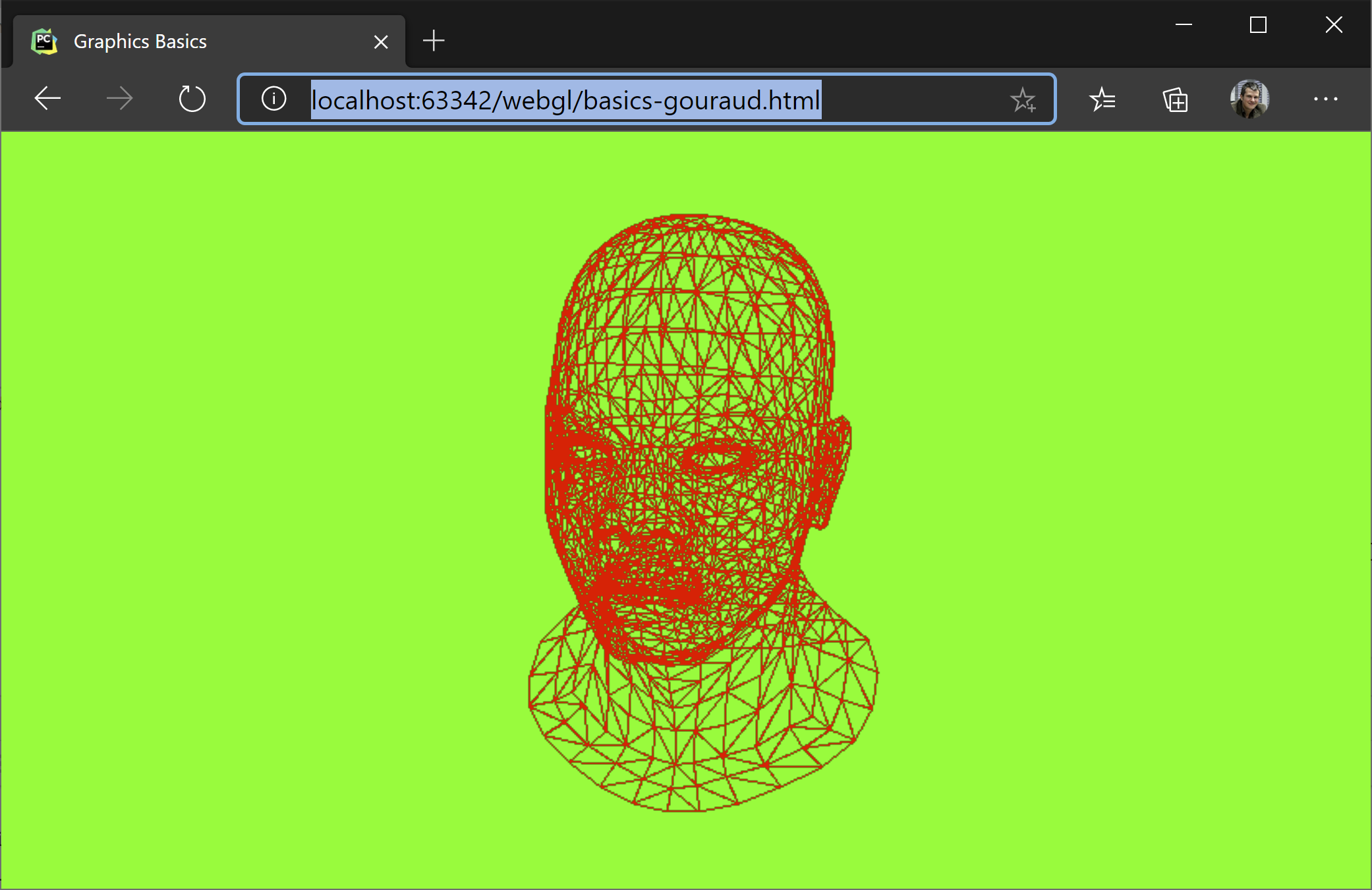For this, let’s look at our `generateImage` function and what it does if the `wireframe` parameter is set to `true`.

The first step is to clear the background and the z-buffer. For wireframe rendering we don’t care about the z-buffer, but we do care about not drawing on top of an older image. So we put all pixels to green.

Another thing we care about is transforming our vertices from their world coordinates to their corresponding screen coordinates. For this we have a chain of transformations (matrix multiplications) we apply to each vertex. Transform `transformsWordToSreen` matrix takes a position in world coordinates and transforms it to `[x, y, z]` in screen space. We will use the `x` and `y` to put the pixel on the screen and `z` to know if it is the topmost pixel and thus not hidden by another pixel. In `varrrayW` we keep the vertices in world coordinates, in `varray` in screen coordinates.

The loop that follows generates the faces, the triangles of our model. As mentioned before, this is an indexed geometry so for each face we need to lookup by index the coresponding vertex in vertex array. We do the same for the normals and for the texture coordinates. These are not relevant for the wireframe rendering, but they are relevant for the next chapter when we shade the triangle. In the last loop, we draw the line.

``````function generateImage(wireframe=true){

// clear background and Z buffer:
clear(0x00, 0xff, 0x00);

if(myMesh == null)
return;

let triangles = [];

/* the following two lines are equivalent to the matrix transformation applied next
let varray = myMesh.vertices.map(v=>homogeneousTransform(vectorMultiply(projectionTransform, v)));
varray = varray.map(v=>homogeneousTransform(vectorMultiply(viewportTransform, v)));
*/

//multiply first with transform because the vector appears later several times
let transformsWorldToScreen = chainMultiplyMatrix([viewportTransform, projectionTransform, cameraTransform])

// tranform the vertices to worldspace and then to screen
let varrayW = myMesh.vertices.map(v => homogeneousTransform(vectorMultiply(myMesh.worldTransform, v, true)));
let varray = varrayW.map(v => homogeneousTransform(vectorMultiply(transformsWorldToScreen, v, true)));

// transform the normals to world
// isPosition == false so we don't translate
let narrayW = myMesh.vnormals.map(v => normalize(vectorMultiply(myMesh.worldTransform, v, false)));

// each face has 9 indices, only the 0, 3, 6 are vertex index
for(let i = 0; i < myMesh.faces.length; i++){

// index in the vertex buffer
let v0 = myMesh.faces[i];
let v1 = myMesh.faces[i];
let v2 = myMesh.faces[i];

// texture vertex index
let tx0 = myMesh.txcoords[myMesh.faces[i]];
let tx1 = myMesh.txcoords[myMesh.faces[i]];
let tx2 = myMesh.txcoords[myMesh.faces[i]];

// vertex normal coords world space
let vn0 = narrayW[myMesh.faces[i]].slice(0, 3);
let vn1 = narrayW[myMesh.faces[i]].slice(0, 3);
let vn2 = narrayW[myMesh.faces[i]].slice(0, 3);

// world space backface culling
let faceNormal = normalize(crossProduct3(
subtractVector(varrayW[v2], varrayW[v0]),
subtractVector(varrayW[v1], varrayW[v0])
));

let visible = dot(cameraDir, faceNormal) >= 0;

if(visible || wireframe) {
triangles.push([varray[v0], varray[v1], varray[v2], vn0, vn1, vn2, tx0, tx1, tx2]);
}

}

if(wireframe) {
// TODO: remove duplicated lines, each line is drawn several times
for (let i = 0; i < triangles.length; i++) {
let t = triangles[i];
drawLineV(t, t, 0xff, 0, 0);
drawLineV(t, t, 0xff, 0, 0);
drawLineV(t, t, 0xff, 0, 0);
}
}
else{
for(let i=0; i<triangles.length; i++){
let t = triangles[i];
drawTriangle(...t);
}
}
}
``````

### PutPixel and Line Drawing

As mentioned before, we don’t use any library for this demo. So we will implement our `drawLine` from scratch. Here it is how it goes. `screenBuffer` is our pixel matrix, organized as `RGBA`, each one byte in length.

``````function putPixel(x, y, r=0xff, g=0x00, b=0x00) {

const idx = (Math.round(y) * screenBuffer.width + Math.round(x)) * 4;

screenBuffer.data[idx + 0] = r;
screenBuffer.data[idx + 1] = g;
screenBuffer.data[idx + 2] = b;
screenBuffer.data[idx + 3] = 0xff;

}

function drawLine(x0, y0, x1, y1, r, g, b) {

// no line
if (x0 === x1 && y1 === y0)
return;

// step
let step = 1.0 / Math.max(Math.abs(x0 - x1), Math.abs(y0 - y1));

for(let i = 0; i <= 1; i+= step){
let x = x0 + i * (x1-x0);
let y = y0 + i * (y1-y0);
putPixel(x, y, r, g, b);
}
}
``````

### Positions and Directions in Homogenous Coordinates

In order to be able to add a rotation, a translation and a projection in a single matrix multiplication step, we extend our `[x, y, z]` notion of a point in 3D space to `[x, y, z, w]`, which is congruent to the `[x/w, y/w, z/w, 1]`. This division is, in fact, a projection from the 4D space to the 3D space.

For orthogonal transformations, e.g. world-space transformations, vectors that represent points have `w == 1` and vectors that represent directions, defined as `p1 - p2`, have their `w == 0`.

### Rendering Full Triangles

The most exciting part of our blog post is about rendering full triangles. Before we dive into the actual shading, let’s say the obvious that we only care about the triangles that are facing us. So we do a simple test. This test is called back-face culling. This is why counter-clockwise convetion for defining faces is important. If we weren’t following it, we’d have the normals oriented in the opposite direction.

``````// world space backface culling
let faceNormal = normalize(crossProduct3(
subtractVector(varrayW[v2], varrayW[v0]),
subtractVector(varrayW[v1], varrayW[v0])
));
let visible = dot(cameraDir, faceNormal) <= 0;
``````

We compute the face normal using the `crossProduct3` function which, given a plane (3 points), computes a fourth vector perpendicular to the others. Then we check to see if the face normal and the `cameraDir` face in the opposite direction. This is what the `dot` product does.

The remaining part is covered in the `drawTriangle` function. The algorithm is very simple and it fits very well on massively parallel hardware as all triangles can be processed in parallel.

• Find a bounding box for our triangle
• Shade each point from the bounding box only if inside the triangle

The parameters for the function are:

• `v1, v2, v3` - triangle vertices transformed in screen space
• `vn1, vn2, vn3` - vertex normals
• `tx0, tx1, tx2` - texture coordinates for each vertex
``````function drawTriangle(v1, v2, v3, vn1, vn2, vn3, tx0, tx1, tx2){

// find the bounding box
let bb = [v1, v1, v1, v1];
let v = [v2, v3];
for (let i = 0; i < v.length; i++){
bb = Math.floor(Math.min(bb, v[i]));
bb = Math.floor(Math.min(bb, v[i]));
bb = Math.ceil(Math.max(bb, v[i]));
bb = Math.ceil(Math.max(bb, v[i]));
}

// check if the point is inside the triangle
for(let i = bb; i <= bb; i++)
for(let j = bb; j <= bb; j++){

const stu = toBarycentricCoords(i, j, v1, v2, v3);

if(insideTriangle(stu, stu, stu)) {

// interpolate over the z coord
const pixelZWorld = stu * v1 + stu * v2 + stu * v3;
const zBufferIndex = zBufferGetIdx(i, j);

if (pixelZWorld >= zBuffer[zBufferIndex]){
zBuffer[zBufferIndex] = pixelZWorld;

// use again the barycentric coords to interpolate in the texture
// matrix multiplication STU * [tx0, tx1, tx2]
const tX = dot(stu, [tx0, tx1, tx2]);
const tY = dot(stu, [tx0, tx1, tx2]);

[tr, tg, tb] = getTextureData(tX, tY);

// interpolate normals (all in world space)
const n0 = dot(stu, [vn1, vn2, vn3]);
const n1 = dot(stu, [vn1, vn2, vn3]);
const n2 = dot(stu, [vn1, vn2, vn3]);

let intensity = -dot(lightDir, [n0, n1, n2]);
let c = Math.max(0, intensity);

putPixel(i, j, c * tr, c * tg, c * tb);
//putPixel(i, j, 255 * c , 255 * c , 255 * c ); // draw only the light intensity
}
}
}
}
``````

The most interesting point of this function is transforming each pixel inside the triangle to its barycentric coordinates. These coordiantes are 3 numbers, `s, t, u`, which give weights to how close the point is to each vertex. That is, `v1` would have barycentric coordiantes of `1, 0, 0`, `v2` would have its barycentric coordinates at `0, 1, 0` and `v3` at `0, 0, 1`. Obviously, `s + t + u == 1` and they allow linear interpolation for each pixel based on values stored in the face vertices. If a pixel is outside of our triangle, at least of its barycentric coordinates is negative.

So what do we do if the pixel is inside the triangle:

• We check if the pixel is not under another pixel previously rendered (z-buffer check). We can simply interpolate the `z value` for the pixel and compare it with what is stored in the z-buffer. Since everything is already projected on the screen, we take the z directly without any other transformation.

• We interpolate between the texture coordiantes for each vertex and take the corresponding diffuse value.

• We interpolate between the normals of each vertex to compute a pixel normal and `dot` it with the light direction to see how much light falls on that point. This is called `Phong Shading`, as opposed to `Gouraud Shading` where the light is calculated per vertex and then interpolated over the surphace.

### What Else?

Building the camera matrix, which is similar to the `gluLookAt` from OpenGL. The two functions are interesting becasue they show two things:

• How to make the inverse of an homogenous orthogonal matrix based on the transposed rotation.
• How to extract the axes of abject. Axes are oriented on columns.
``````function inverseOrthogonalMatrix(mtx){

// inverse is the transpose of the rotation part and `-` the translation

let x = mtx.slice(0, 4);
let y = mtx.slice(4, 8);
let z = mtx.slice(8, 12);

let rotate = [
x, y, z, 0,
x, y, z, 0,
x, y, z, 0,
0,    0,    0,    1
];

let translate = [
1, 0, 0, -x,
0, 1, 0, -y,
0, 0, 1, -z,
0, 0, 0, 1
]

// inverse = a) -translate followed by b) -rotate
return matrixMultiply(rotate, translate);

}

function makeCameraTransform(camPos, camUp, camLookAt){

// camera looks towards -z, so here we need to inverse camCenter and camPos
let z = normalize(subtractVector(camPos, camLookAt))
let y = normalize(camUp);
let x = crossProduct3(y, z);
y = crossProduct3(z, x);

let camWorld = [
x, y, z, camPos,
x, y, z, camPos,
x, y, z, camPos,
0,    0,    0,    1,
]

let ret = inverseOrthogonalMatrix(camWorld);
//let identity = matrixMultiply(ret, camWorld); // debug
return ret;
}
``````

The depth buffer is initialized to the same size as the whole canvas, based on floats. For faster computations it can be initialized to integer numbers, but then care must be taken to defining the resolution in the viewport matrix.

``````zBuffer = new Float32Array(cvs.width * cvs.height);
``````

Making the render buffer is done as follows:

``````function makeFullScreenCanvas(){

const cvs = document.getElementById('myCanvas');
cvs.width = window.innerWidth;
cvs.height = window.innerHeight;

const ctx = cvs.getContext("2d");
screenBuffer = ctx.createImageData(cvs.width, cvs.height);
zBuffer = new Float32Array(cvs.width * cvs.height);

viewportTransform = makeViewportTransform(cvs.width, cvs.height);
projectionTransform = makeProjectionTransform(3);
cameraTransform = makeCameraTransform([0.2, 0.2, 0.8], [0, 1, 0], [0, 0, 0]);

render();
}
``````

And, before we go, let’s have a look once again at our model with all the transformations applied - this should be the output of running the code from github.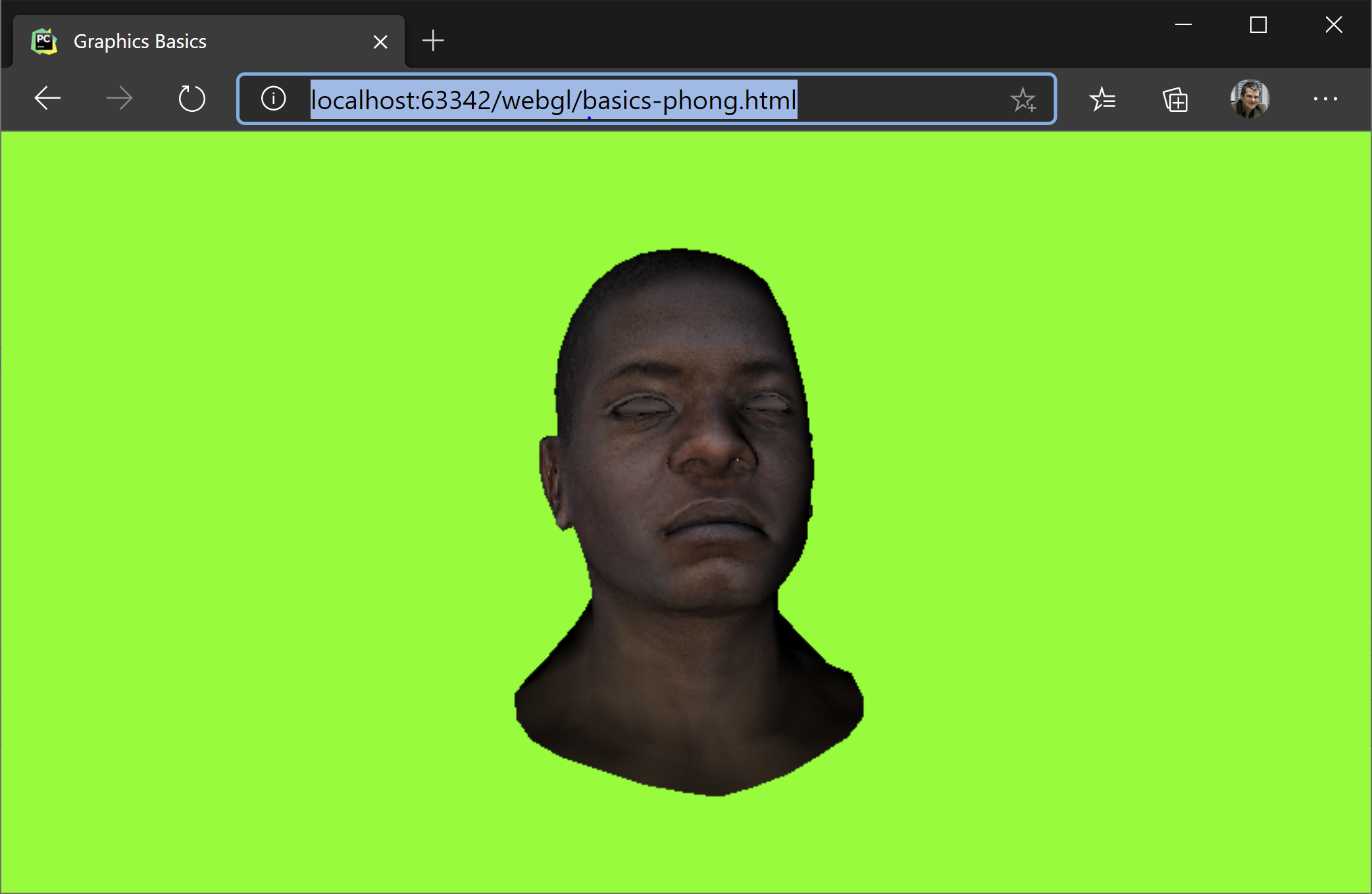]]>
Introduction to Cryptography (Part 3)2020-04-04T09:15:16+02:002020-04-04T09:15:16+02:00https://alexandrugris.github.io/cryptography/2020/04/04/intro-cryptography%203This is the third part of Introduction to Cryptography. The post covers the Java APIs that implement the same algorithms that we spoke about in the previous posts, symmetric and asymmetric encryption, as well as digital signatures. We also talk a little bit about password security and the principle behind rainbow tables.

### Java APIs, Encryption, Decryption, Signatures

I am going to exemplify here the concepts from the previous posts using the Java Cryptography Extensions (JCE). Most programming languages have similar cryptographic support. JCE revolves around the following classes:

• `KeyGenerator` - key generator for symmetric encryption
• `SecretKey` - the generated symmetric key
• `SecureRandom` - cryptographically secure random number generator
• `IvParameterSpec` - initialization vector for the algorithm (remember that the Cypher Block Chaining (CBC) requires an init vector)
• `KeyPairGenerator` - key generator for asymmetric encryption
• `PublicKey` - the public key
• `PrivateKey` - the private key
• `Cipher` - perform the work of the symmetric / asymmetric encryption
• `Signature` - performs the work of the signature algorithm
• `CipherInputStream` - input stream for decryption
• `CipherOutputStream` - output stream for encryption

The current Java implementation, Java 14, supports the following algorithms: link

``````/**
* Encrypting with symmetric encryption. Only the necessary information is shared with this method.
* In a production scenario, these would come from a secrets database
* @param msg - the message
* @param algorithm - the algorithm
* @param key - the secret key
* @param iv - the initialization vector
*/
static byte[] encryptAES(String msg, String algorithm, SecretKey key, IvParameterSpec iv)
NoSuchAlgorithmException,
InvalidAlgorithmParameterException,
InvalidKeyException {

Cipher c = Cipher.getInstance (algorithm);
c.init (Cipher.ENCRYPT_MODE, key, iv);
var output = new ByteArrayOutputStream ();
try(var cos = new CipherOutputStream (output, c)){
cos.write (msg.getBytes ());
}
catch (IOException exx){
exx.printStackTrace ();
}
return output.toByteArray ();
}
/**
* The decryption function
* @param encrypted - the text to be decrypted
* @param algorithm - the algorithm used
* @param sk - the secret key
* @param iv - the initialization vector
* @return
*/
static String decryptAES(byte[] encrypted, String algorithm, SecretKey sk, IvParameterSpec iv)throws
InvalidAlgorithmParameterException,
InvalidKeyException,
NoSuchAlgorithmException {

Cipher c = Cipher.getInstance (algorithm);
c.init (Cipher.DECRYPT_MODE, sk, iv);

try(var bais = new ByteArrayInputStream(encrypted);
var cis = new CipherInputStream (bais, c)){

} catch (IOException e) {
e.printStackTrace ();
}
return null;
}

/**
* Start here
*/
static void test_symmetricJCE()
throws NoSuchAlgorithmException,
InvalidAlgorithmParameterException,
InvalidKeyException {

// Generate the secret key
KeyGenerator keyGen = KeyGenerator.getInstance ("AES");
keyGen.init (256);

SecretKey sk = keyGen.generateKey ();
assert sk.getAlgorithm ().equals ("AES"); // algorithm
assert sk.getEncoded ().length == 32; // key size in bytes

// Create an instance of the AES cypher, with CBS and
// a padding to fill the missing bytes at the end of the message.
// Generate the initialization vector for our CBC.
// We use the block size from the algorithm for the size of our iv
SecureRandom sr = new SecureRandom ();
byte[] ivbytes = new byte[Cipher.getInstance ("AES/CBC/PKCS5Padding").getBlockSize ()];
sr.nextBytes (ivbytes);
IvParameterSpec iv = new IvParameterSpec (ivbytes);

// encrypt and decrypt
var msg = "This is my first long message encrypted with AES / CBC";
var encrypted = encryptAES(msg, "AES/CBC/PKCS5Padding", sk, iv);
var decrypted = decryptAES(encrypted, "AES/CBC/PKCS5Padding", sk, iv);

assert msg.equals (decrypted);
}
``````

In the picture below we can observe that the secret key is just an array of bytes, similar to what we have seen when we implemented the algorithm from scratch, in the previous post.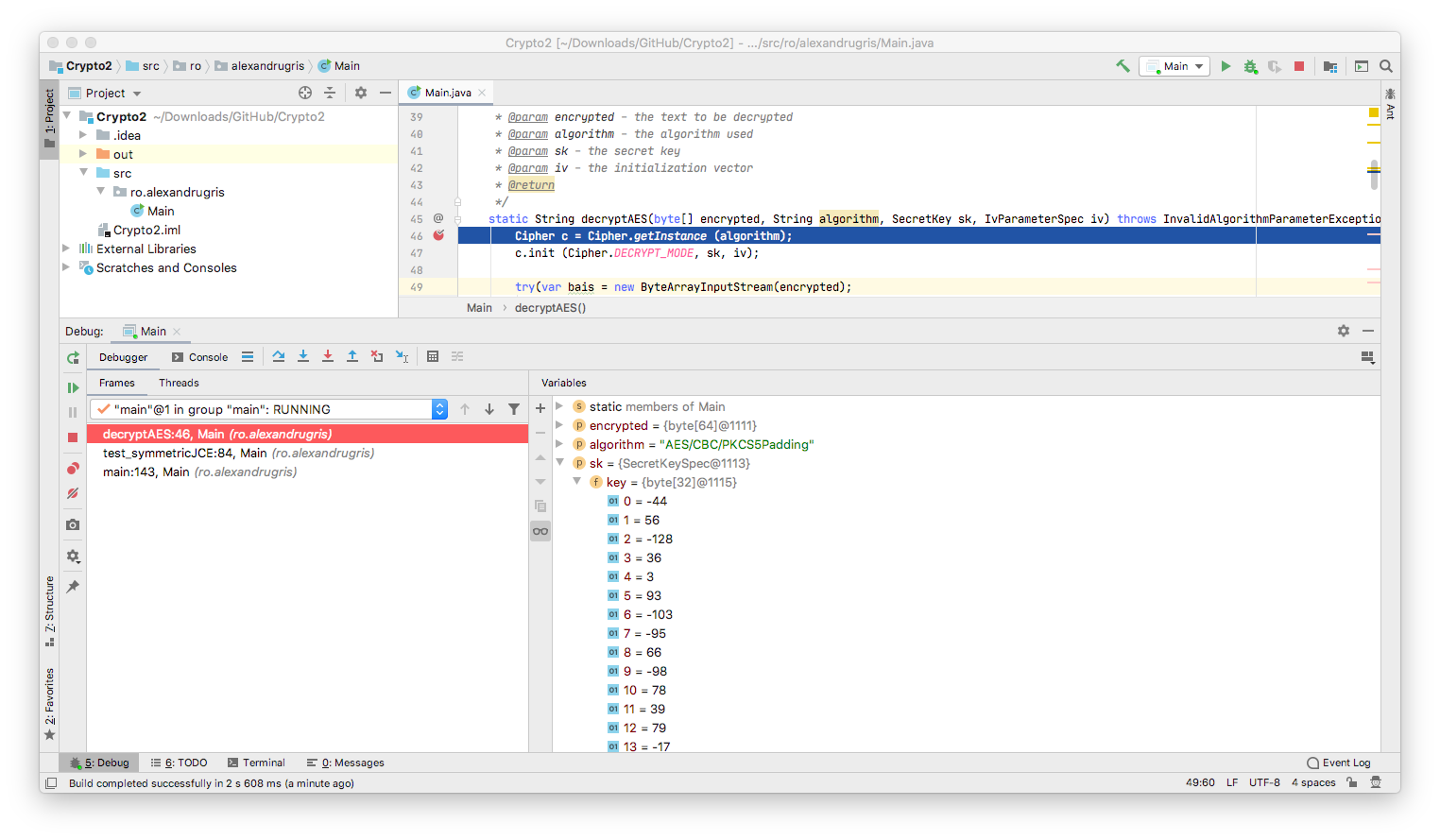It is important to note that, if two messages start with the same bytes, the first bytes in the encrypted string for both of them will be the same, if we use the same initialization vector. Therefore, it is good practice to change the initialization vector with each message.

For the asymmetric encryption, the process is very similar. The only differences are in the methods we call on the `Cipher` class. Since `Cipher` works iteratively on blocks, to encrypt / decrypt with `RSA` which is not a block cipher, we need to invoke `Cipher::doFinal()` on the cipher, as if the whole message is a single block. Example below.

``````private static void test_asymmetricJCE() throws
NoSuchAlgorithmException,
InvalidKeyException,
IllegalBlockSizeException {

var msg = "This is my first long message encrypted with RSA";
KeyPairGenerator kg = KeyPairGenerator.getInstance ("RSA");
kg.initialize (2048);

var kp = kg.generateKeyPair ();

// encrypt
c1.init (Cipher.ENCRYPT_MODE, kp.getPrivate ());
byte[] encrypt =  c1.doFinal (msg.getBytes ());

// decrypt
c2.init (Cipher.DECRYPT_MODE, kp.getPublic ());
var ret = new String(c2.doFinal (encrypt));
assert ret.equals (msg);
}
``````

In the picture below we can see the public / private key pair expanded. We observe the same elements that we spoke about when we implemented the algorithm from scratch, in the previous post:

• `p` and `q` my private two large prime numbers
• `n = p*q`, the modulo, shared
• `e`, the public exponent - shared (encrypting) - the requirement for this is to be relatively prime to `p-1` and `q-1`. A commonly used exponent is `65537` since it is a prime number all together .
• `d`, the private exponent - shared (decrypting) -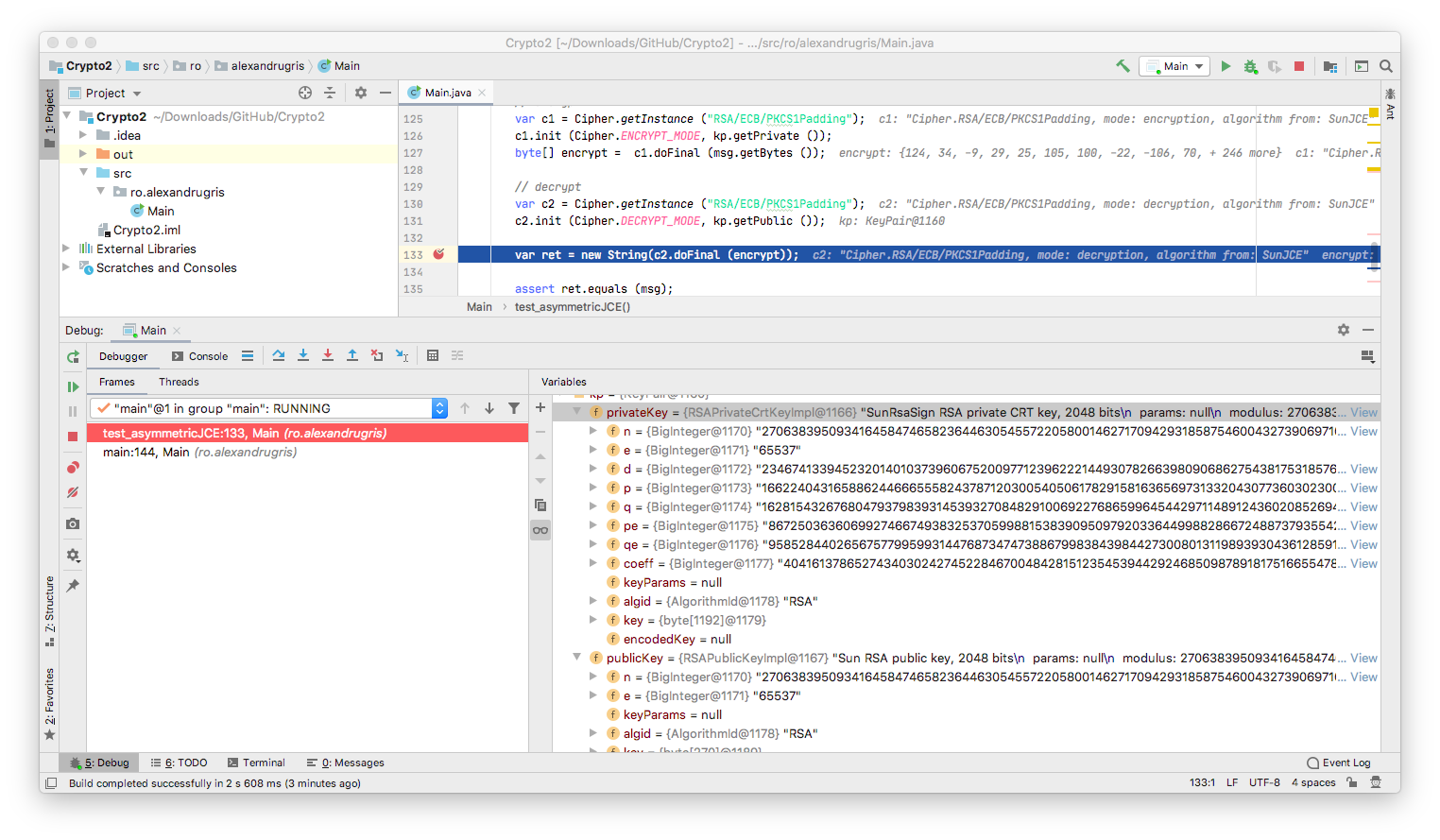Several important notes:

• `KeyPairGenerator::generateKeyPair()` might take several seconds. Therefore, it is better to store / read the keys from a secure key store.

• The RSA algorithm is generally slow so, in practice, it is used to `encrypt -> transmit -> decrypt` a key that will be used further with a symmetric encryption algorithm. In our case, we would have had encrypted the `SecretKey` from the first example, transmit it over the wire, then use that `SecretKey` to encrypt the rest of the communication.

Now, let’s use the private / public key pair to sign a message:

``````private static void test_signaturesJCE() throws NoSuchAlgorithmException, InvalidKeyException, SignatureException {

var msg = "This is my first long message signed with RSA";

KeyPairGenerator kg = KeyPairGenerator.getInstance ("RSA");
kg.initialize (2048);

var kp = kg.generateKeyPair ();

// sign
var sigSign = Signature.getInstance ("SHA256withRSA");
sigSign.initSign (kp.getPrivate ());
sigSign.update (msg.getBytes ());
var sig = sigSign.sign ();

// verify
var sigVerify = Signature.getInstance ("SHA256withRSA");
sigVerify.initVerify (kp.getPublic ());
sigVerify.update (msg.getBytes ());
var ret = sigVerify.verify (sig);

assert ret;
}
``````

### Authentication and Authorization

The first thing to know about passwords is that you never store them in clear text. More precisely you don’t even need to store the full password in any form. Since the verification is just one way, it is enough to store a password hash that is checked against every time the password is entered. The most basic form for checking whether a site keeps passwords in clear text is so see if they offer a password retrieval function. If they do, better close the account and never use that password again.

A more common form of attack are leaked password hashes. We could use dictionary attacks to match hashes to known passwords and that would lead to dictionaries being extremely large. A method that trades the size of the dictionary for a bit of additional computation is the rainbow table. The principle is to compute a series of chains, pairs of `(starting password, ending hash)`. Each chain is, in fact, like (starting password -> hash -> new password -> hash … -> ending hash), but, since we know the transform function from password to hash and then from hash to a new potential password, we don’t need to store the intermediate results. We don’t want to reverse the hash, but to try to find a collision. What is needed for rainbow table to work are (a) a leaked the password hash and (b) the algorithm used for obtaining that hash. The algorithm starts by identifying which chain the leaked hash belongs to and then, iterating through the chain, find a password that generates that very same hash.

Here is a very basic example of the principle, written in Java. The code is based on this excellent article.

``````package ro.alexandrugris;

import java.nio.charset.StandardCharsets;
import java.util.HashMap;

public class Main {

// for simplicity, in our case, hash -> password function is just the identity function
static String hash(String s){

byte[] str = s.toUpperCase ().getBytes (StandardCharsets.US_ASCII);
byte[] n_pass = new byte[str.length];

// a very basic and a very bad hash function
for(int i = 0; i < str.length; i++){
var x = str[i] - 'A';
var hash = (int)(2000 * (x * 1.618 % 1));
n_pass[i] = (byte)(hash % ('A' - 'Z') + 'A');
}

return new String (n_pass);
}

static String computeChain(String start){

// chains of 4, because our hash function is very weak and it loops very quickly.
for (int i = 0; i < 4; i++){
start = hash (start);
}
return start;
}

static String guessPassword(String initial, HashMap<String, String> map){

String hash = initial;

// N = 100 tries
for(int i = 0; i < 100; i ++){
// 3. try to find the hash in the rainbow table
var chain = map.get (hash);

if(chain == null){
hash = computeChain (hash);
}
else{
// 5. the hash was found, which means I found the chain
// start from the beginning of the chain,
// compute the hash.
// When the hash is equal to the hash I want to break,
// that is a working password!
while(true){
var next = computeChain (chain);
if(next.equals (initial))
return chain;
else
chain = next;
}
}
}
}

public static void main(String[] args) {

// 1. compute rainbow table, a hashmap of <hash, starting point>
HashMap<String, String> myRainbowTable = new HashMap<> ();

String[] startingPoints = {
"HELL",
"BUBU",
"FUFU",
"ROCK"
};

for (var s : startingPoints){
myRainbowTable.put (computeChain (s), s);
}

// 2. obtain the password hash we want to reverse
var passHash = "WJGG";
}
}
``````

The interesting thing to observe is how an increased password complexity increases exponentially the complexity of generating and searching the rainbow table. It also shows that for salted passwords an attacker will have a harder time reversing it as it has to start by generating the rainbow table for those specific salts. The salt itself, a string pre-pended or appended to the password, does not need to be protected. It can be stored in plain text in the passwords table, but, for good protection, it should different for every user.

To make it unfeasible for an attacker to brute force our passwords, the algorithm used to compute the hash should be (a) irreversible (b) take a long time. The application only runs this algorithm for each login, but the attacker would have to run it for every password retry. The recommended approach is called `PBKDF` and the general concept is called key stretching.

``````static String passwordHash(String password, String salt, int iterations, int keyLength)
throws NoSuchAlgorithmException, InvalidKeySpecException {

SecretKeyFactory f = SecretKeyFactory.getInstance ("PBKDF2WithHmacSHA1");

// iterations should be minimum 1000, preferably 10000
// should be increased as computers become more powerful
// the idea is to have a time-consuming operation
// that makes it computationally hard for the attacker to brute force the password
KeySpec ks = new PBEKeySpec (password.toCharArray (), salt.getBytes (), iterations, keyLength);
SecretKey s = f.generateSecret (ks);

return new String(Base64.getEncoder ().encode (s.getEncoded ()));
}
``````
]]>
Introduction to Cryptography (Part 2)2020-03-29T09:15:16+02:002020-03-29T09:15:16+02:00https://alexandrugris.github.io/cryptography/2020/03/29/intro-cryptography%202This is the second part of Introduction to Cryptography. The post covers symmetric encryption with cypher block chaining, the principles behind DES and AES, asymmetric encryption with RSA and a little bit on validating authenticity.

### Symmetric Encryption with Cipher Block Chaining (CBC)

Block cipher is a very simple concept. It acknowledges the practical impossibility of sending a purely random, equal in size to the message, secret of the one-time pads and addresses it. It splits the message into predefined size blocks, then encrypts each block individually. Cipher Block Chaining takes the concept even further by taking into account the ciphertext generated for the previous blocks when encrypting the current block.

For Block Ciphers, Shannon introduced two measures:

• Confusion: how much a change in the key leads to a change in the ciphertext
• Diffusion: how much a change in the text leads to a change in the ciphertext

We want both confusion and diffusion to be as high as possible.

To increase confusion, we want for a small change in the key to lead to a large change in the text. If we were to apply a simple `text XOR key` on each block independently, one bit of change in the key leads to one bit of change in the ciphertext, thus a very low confusion. Therefore, a good CBC algorithm work in several encryption rounds on each block.

Similarly, we want a small change in the text to lead to a large change in the ciphertext. Therefore, we cannot simply apply the same key over and over the encrypted message, independently, block from block. That would encrypt the block itself, but patterns in the text would still be apparent when the same group of characters reappears. Therefore, we use the ciphertext from the previous block as a part of encryption of the current block. This ensures that every block in the message depends on all the previous blocks. Thus, a small change in the message early in the stream would lead to a large change of the ciphertext, spread all the way to the end, hence increasing diffusion.

Below is a very basic implementation of this concept, with a single XOR round and with block chaining.

``````private static byte[] initVector(int blockSize){
byte[] initVector = new byte[blockSize];
(new Random (42)).nextBytes (initVector); // given key
return initVector;
}

private static byte[] encryptDecryptCBC(byte[] msg, byte[]key, boolean encrypt){
int blockSize  = key.length;
byte[] initVector = initVector (blockSize);
// for each block the encryption should be done as a series of rounds,
// but we are going to use a single XOR round for this example
byte[] currentBlock = new byte[blockSize];
for (int i = 0, j = 0; i<msg.length; i++){
// one simple XOR round and diffusion with previous block
byte v = (byte) (msg[i] ^ key[j] ^ initVector[j]);
currentBlock[j] = encrypt? v : msg[i];
msg[i] = v;
if (++j == blockSize){
initVector = currentBlock;
currentBlock = new byte[blockSize];
j = 0;
}
}
return msg;
}

private static byte[] encryptCBC(String s, String password){
byte[] msg = s.getBytes (StandardCharsets.US_ASCII);
return encryptDecryptCBC (msg, key, true);
}

private static String decryptCBC(byte[] msg, String password){
return new String(encryptDecryptCBC (msg, key, false));
}

/***
* Here it starts
*/
public static void test_CypherBlockChaining() throws Exception {
String myMsg = "This is a message which is long";
byte[] msg = encryptCBC (myMsg, "Hello World");
String ret = decryptCBC (msg, "Hello World");
if(!ret.equals (myMsg))
throw new Exception ("Wrong Algorithm");
}
``````

One of the early implementations was `DES`, currently considered obsolete due to the short key length. A newer one is `AES`, which is run with keys 128, 192 or 256 bits. `AES` runs on blocks of 16 bytes and applies a series of rounds to to confuse the key, including operations like shifting rows, mixing rows, XOR-ing, side lookups. `AES-256` is considered strong enough to encrypt government secrets.

When sending a message, the following order of operations must be kept:

1. Compression
2. Encryption
3. Error correction

Compression reduces redundancy in the message. If encryption is applied before compression, due to diffusion which is aimed at masking redundant patterns, compression becomes ineffective. Error correction adds redundancy to the message. Thus, if applied it the same step as the encryption, as the `DES` algorithm did, it decreases the encryption strength. If applied before, it loses its meaning as accidental information loss can occur during transmission, not at encryption time.

### Asymmetric Algorithms

The principle of asymmetric algorithms is simple. The emitter keeps a function `ciphertext = f(text)` to himself while giving the recipient its inverse `text = f-1(ciphertext)`. In more practical terms, I want to give you a an encrypting exponent `e` and a modulus `m` so that you can `message^e % m = ciphertext` while I can `ciphertext^d % m = message`, where `d` is the decrypting exponent.

The principle of exponentiation in the modulo has been discussed in the previous post. In short, exponentiation in the modulo has the following interesting properties:

• the result “jumps around”, so it is very hard to predict the root
• the exponentiation operation can be performed fast
• its inverse, the logarithm problem, is very time consuming

The code below exemplifies the algorithm. The modulo exponentiation function is described in the previous post. Important to note that each chunk of message we can encrypt with this process must be lower than `modulo`.

But how do we get the encrypting/decrypting exponent pairs and the modulo? The maths goes like this:

1. We select the modulo `m = p * q` where `p` and `q` are two (large) prime numbers.
2. The formula for inverse assumes that `(message^e)^d % m = message` which is equivalent to `message ^ (e * d) % m = message`
3. Because we selected `m = p * q`, we obtain `message^(e * d) % p = message^(e * d) % q = message`, which is true when `message < p` and `message < q`.
4. This is equivalent to saying `message^(e * d - 1) * message % p = message` which looks very much like the Fermat’s Little Theorem which tells us that `m^(p-1) % p = 1` when `p` is prime and `m` is not a multiple of `p`
5. If we select `e * d = k * (p-1)` where `k` is a constant and plug it in point 4, we obtain `(message^(p-1))^k * message % p = message` which is equivalent to `1^k * message % p = message` which is equivalent to `message % p = message`.
6. If we proceed in an identical manner on the `q` side, we get `e * d - 1 = k * (p-1) * (q-1)`. So we need to find `k` and `d`, both integers, which satisfy the above equation.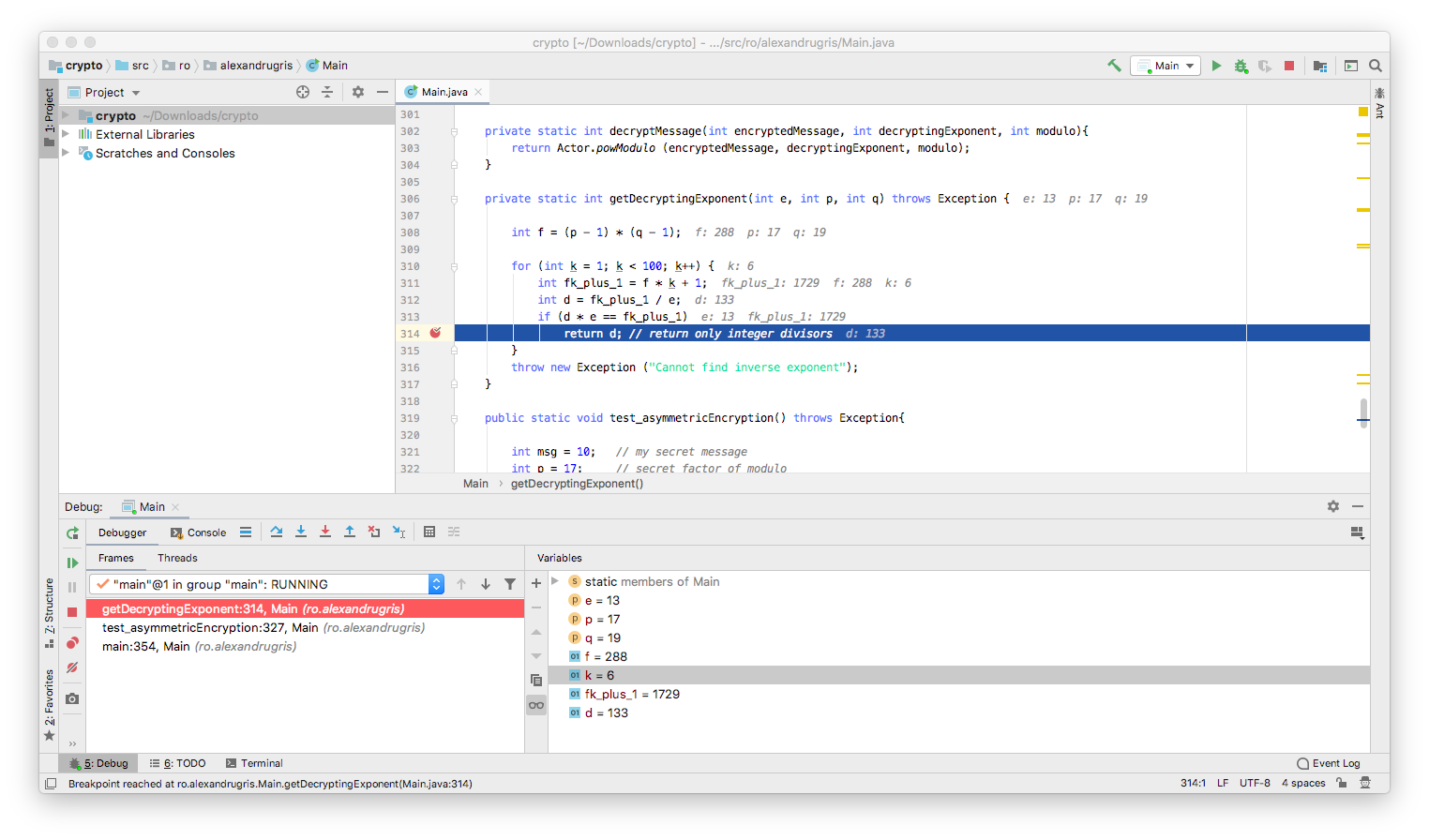Let’s do just that:

``````private static int encryptMessage(int msg, int encryptingExponent, int modulo) throws Exception {
// if msg == modulo, exponentiation returns 0 => message is compromised
// from there on, it loops again through the same values => impossible to construct the msg
if(msg >= modulo)
throw new Exception ("Cannot encrypt messages larger than modulo");
return Actor.powModulo (msg, encryptingExponent, modulo);
}

private static int decryptMessage(int encryptedMessage, int decryptingExponent, int modulo){
return Actor.powModulo (encryptedMessage, decryptingExponent, modulo);
}

private static int getDecryptingExponent(int e, int p, int q) throws Exception {

// here we search blindly for this k,
// a better algorithm can be found in wikipedia
// (RSA and Extended Euclidean Algorithm)
int f = (p - 1) * (q - 1);
for (int k = 1; k < 100; k++) {
int fk_plus_1 = f * k + 1;
int d = fk_plus_1 / e;
if (d * e == fk_plus_1)
return d; // return only integer divisors
}
throw new Exception ("Cannot find inverse exponent");
}

public static void test_asymmetricEncryption() throws Exception{
int msg = 10;   // [SECRET] my secret message
int p = 17;     // factor of modulo, large prime - [SECRET]
int q = 19;     // factor of modulo, large prime - [SECRET]
int modulo = p * q; // msg should be less than modulo for encryption to work [PUBLIC]
int encryptingExponent = 13; // my public key [PUBLIC]
// my private key [SECRET]
int decryptingExponent = getDecryptingExponent(encryptingExponent, p, q); /
// [PUBLIC]
int encryptedMsg = encryptMessage (msg, encryptingExponent, modulo);
// [SECRET]
int decryptedMsg = decryptMessage(encryptedMsg, decryptingExponent, modulo);
if (msg != decryptedMsg)
throw new Exception ("Error decrypting the message");
}
``````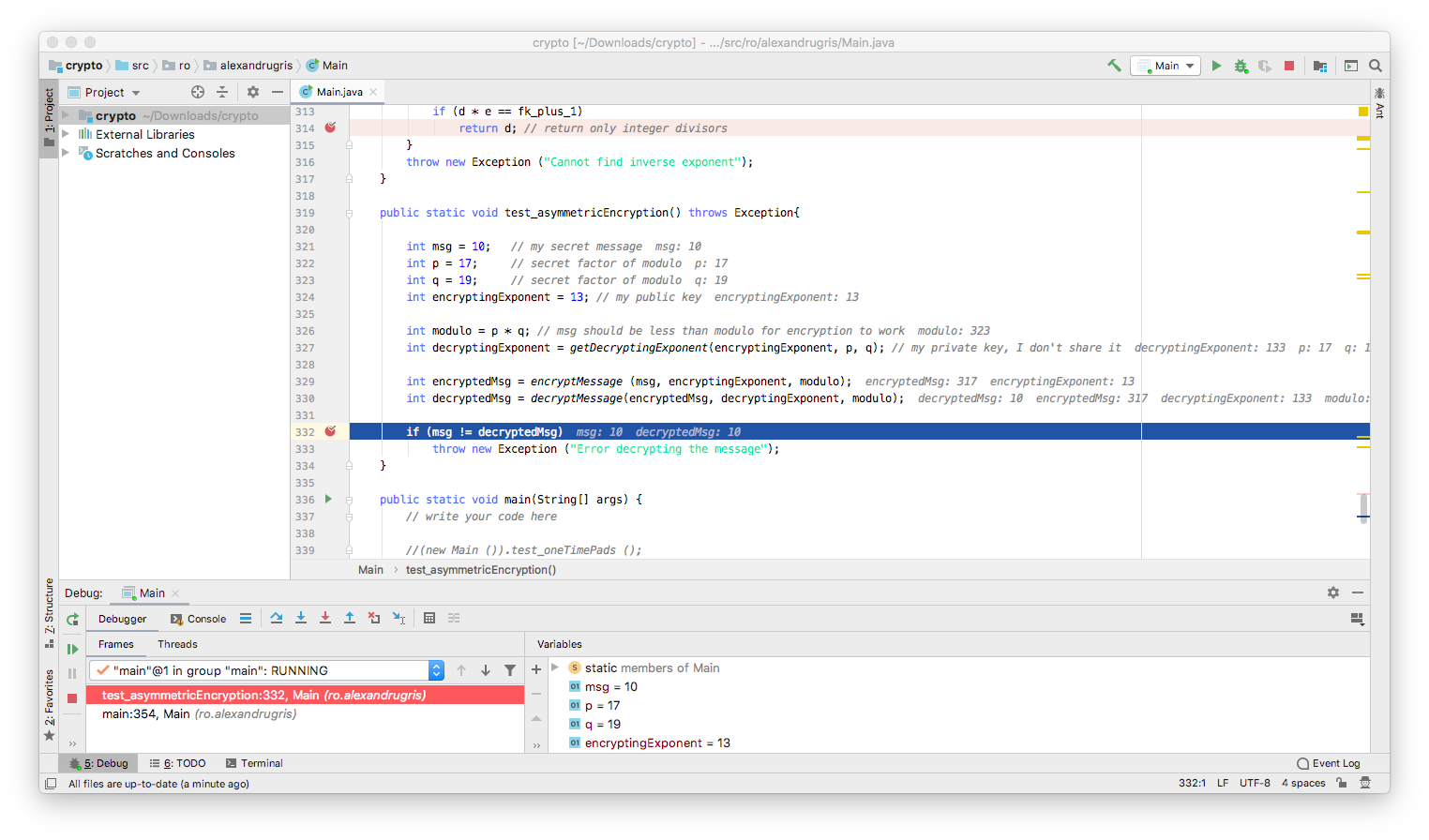The algorithm above is a very rudimentary implementation of the RSA algorithm.

We’ve see in the previous post that, in order to solve the discrete logarithm problem and crack the Diffie-Hellman encryption, we need to perform a scan over the whole set of possible values, from `0` to `modulo` (variable `COUNT` is the `modulo` in our case):

``````for (int j = 0; j < COUNT; j++) {
if (Actor.initialPowModulo (j) == actor1PublicMessage[i]) {
crackedInternalRandoms[i] = j;
break;
}
}
``````

Each extra bit added to the `COUNT` variable doubles the amount of work the algorithm is required to perform. Therefore, the complexity of cracking the code is `O(2^no_of_bits_in_modulo)`.

In 2014 a paper has been published with an algorithm that would bring the discrete logarithm problem to significantly sub-exponential complexity. However, since in `Diffie-Hellman` the modulo is prime and in `RSA` it is a product of two prime numbers, the suggested heuristics from the paper does not (yet) apply.

### Authenticity

If we want to validate that indeed a message comes from a specific person, we do the following:

Person A, originator:

1. Hash the message with a hash function.
2. Encrypt the hash with the hash with his / her private key
3. Send the encrypted hash along with the message

Person B, recipient:

1. Hash the message with the same hash function
2. Decrypt the received message with Person A’s public key
3. Compare the two hashes - they should be identical.

The weakness is the hash function. If the hash function is easily reversible, a potential attacker could craft a message that has the same hash as the original message and pass it along with the originally encrypted hash. The message, at the recipient, would pass the validation.

Because of this, we need cryptographically strong hash functions. These functions work on the same principles as the CBC described earlier in this chapter. `MD5`, the first designed hash function, is no longer recommended for use, same for `SHA-1` which came later. `SHA-2` (`SHA-256` and `SHA-512`) and `SHA-3` are currently the gold standard in cryptography.

There is still an attack vector open - an attacker can generate a large number of messages that I am likely to sign and a similarly large number of messages that I would not likely sign. This increases the probability that he/she will find a pair of (likely-to-sign, unlikely-to-sign) and thus use my signature to validate his malicious message. Because of this, the protocol for using signatures requires that we never sign a message received from a 3rd party without altering it a little bit before, to generate another hash.

]]>